# Python简介

Python是著名的“龟叔”Guido van Rossum在1989年圣诞节期间，为了打发无聊的圣诞节而编写的一个编程语言。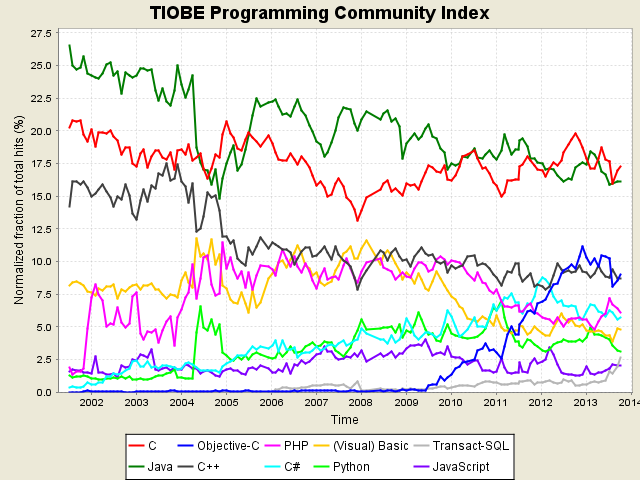Python就为我们提供了非常完善的基础代码库，覆盖了网络、文件、GUI、数据库、文本等大量内容，被形象地称作“内置电池（batteries included）”。用Python开发，许多功能不必从零编写，直接使用现成的即可。# 安装Python

#### 在Windows上安装Python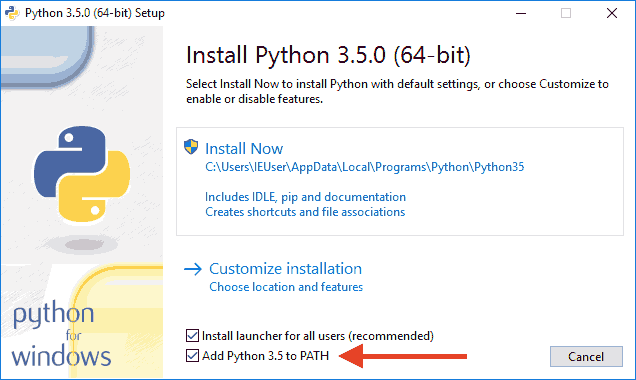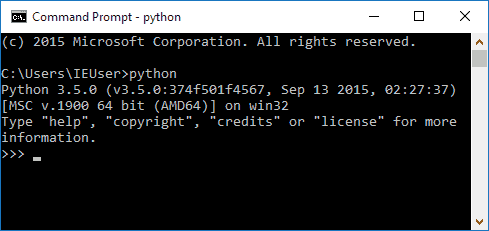``````‘python’ 不是内部或外部命令，也不是可运行的程序或批处理文件。
``````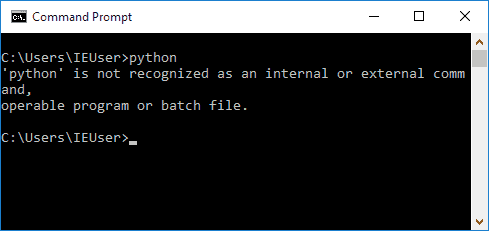## Python解释器

#### CPython

CPython是使用最广的Python解释器。教程的所有代码也都在CPython下执行。

#### IPython

IPython是基于CPython之上的一个交互式解释器，也就是说，IPython只是在交互方式上有所增强，但是执行Python代码的功能和CPython是完全一样的。好比很多国产浏览器虽然外观不同，但内核其实都是调用了IE。

CPython用`>>>`作为提示符，而IPython用`In [序号]:`作为提示符。

#### PyPy

PyPy是另一个Python解释器，它的目标是执行速度。PyPy采用JIT技术，对Python代码进行动态编译（注意不是解释），所以可以显著提高Python代码的执行速度。

#### Jython

Jython是运行在Java平台上的Python解释器，可以直接把Python代码编译成Java字节码执行。

#### IronPython

IronPython和Jython类似，只不过IronPython是运行在微软.Net平台上的Python解释器，可以直接把Python代码编译成.Net的字节码。

#### 小结

Python的解释器很多，但使用最广泛的还是CPython。如果要和Java或.Net平台交互，最好的办法不是用Jython或IronPython，而是通过网络调用来交互，确保各程序之间的独立性。

# 第一个Python程序``````>>> 100+200
300
``````

``````>>> print('hello, world')
hello, world
``````

## 使用文本编辑器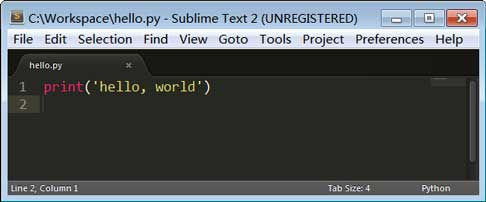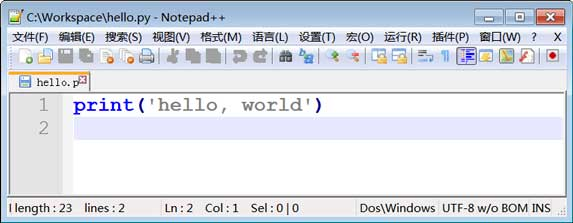``````print('hello, world')
``````

``````C:\work>python hello.py
hello, world
``````

``````C:\Users\IEUser>python hello.py
python: can't open file 'hello.py': [Errno 2] No such file or directory
``````

#### 命令行模式和Python交互模式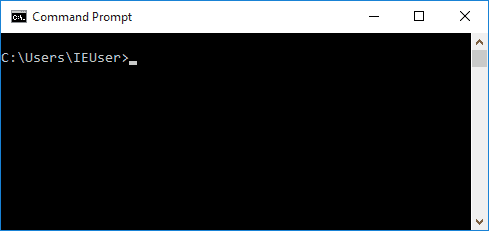``````>>> 100 + 200 + 300
600
``````

``````100 + 200 + 300
``````

``````C:\work>python calc.py
``````

``````print(100 + 200 + 300)
``````

``````C:\work>python calc.py
600
``````

#### 直接运行py文件

``````#!/usr/bin/env python3

print('hello, world')
``````

``````\$ chmod a+x hello.py
``````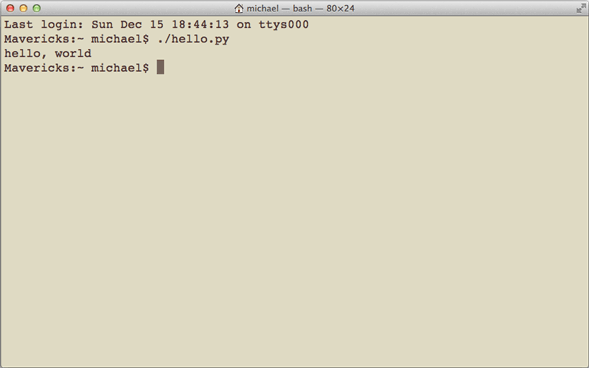#### 小结

Python的交互模式和直接运行`.py`文件有什么区别呢？

hello.py

## Python代码运行助手

Python代码运行助手可以让你在线输入Python代码，然后通过本机运行的一个Python脚本来执行代码。原理如下：

• 在网页输入代码：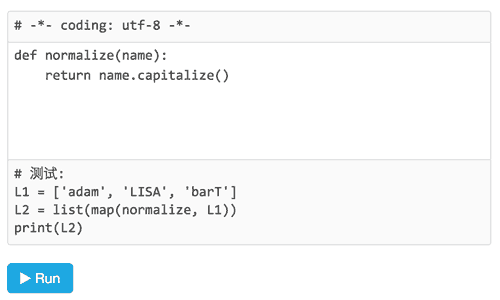• 点击`Run`按钮，代码被发送到本机正在运行的Python代码运行助手；

• Python代码运行助手将代码保存为临时文件，然后调用Python解释器执行代码；

• 网页显示代码执行结果：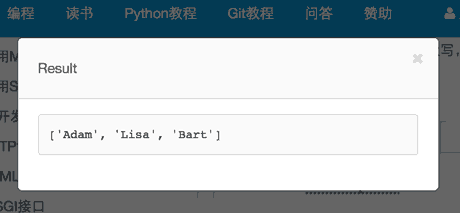#### 运行

``````C:\Users\michael\Downloads> python learning.py
``````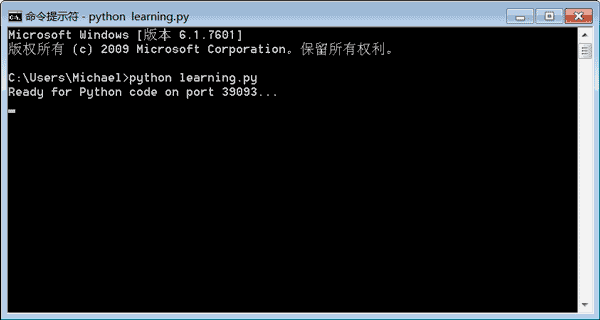#### 试试效果

• IE >= 9
• Firefox
• Chrome
• Sarafi
```# 测试代码:
----
print('Hello, world')
```

## 输入和输出

#### 输出

`print()`在括号中加上字符串，就可以向屏幕上输出指定的文字。比如输出`'hello, world'`，用代码实现如下：

``````>>> print('hello, world')
``````

`print()`函数也可以接受多个字符串，用逗号“,”隔开，就可以连成一串输出：

``````>>> print('The quick brown fox', 'jumps over', 'the lazy dog')
The quick brown fox jumps over the lazy dog
``````

`print()`会依次打印每个字符串，遇到逗号“,”会输出一个空格，因此，输出的字符串是这样拼起来的：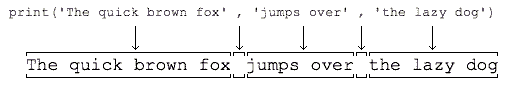`print()`也可以打印整数，或者计算结果：

``````>>> print(300)
300
>>> print(100 + 200)
300
``````

``````>>> print('100 + 200 =', 100 + 200)
100 + 200 = 300
``````

#### 输入

``````>>> name = input()
Michael
``````

``````>>> name
'Michael'
``````

``````>>> print(name)
Michael
``````

``````name = input()
print('hello,', name)
``````

``````C:\Workspace> python hello.py
Michael
hello, Michael
``````

``````name = input('please enter your name: ')
print('hello,', name)
``````

``````C:\Workspace> python hello.py
hello, Michael
``````

#### 小结

`input()``print()`是在命令行下面最基本的输入和输出，但是，用户也可以通过其他更高级的图形界面完成输入和输出，比如，在网页上的一个文本框输入自己的名字，点击“确定”后在网页上看到输出信息。

#### 练习

```# -*- coding: utf-8 -*-
----
print(???)
```

do_input.py

# Python基础

Python是一种计算机编程语言。计算机编程语言和我们日常使用的自然语言有所不同，最大的区别就是，自然语言在不同的语境下有不同的理解，而计算机要根据编程语言执行任务，就必须保证编程语言写出的程序决不能有歧义，所以，任何一种编程语言都有自己的一套语法，编译器或者解释器就是负责把符合语法的程序代码转换成CPU能够执行的机器码，然后执行。Python也不例外。

Python的语法比较简单，采用缩进方式，写出来的代码就像下面的样子：

``````# print absolute value of an integer:
a = 100
if a >= 0:
print(a)
else:
print(-a)
``````

`#`开头的语句是注释，注释是给人看的，可以是任意内容，解释器会忽略掉注释。其他每一行都是一个语句，当语句以冒号`:`结尾时，缩进的语句视为代码块。

#### 小结

Python使用缩进来组织代码块，请务必遵守约定俗成的习惯，坚持使用4个空格的缩进。

## 数据类型和变量

#### 数据类型

##### 整数

Python可以处理任意大小的整数，当然包括负整数，在程序中的表示方法和数学上的写法一模一样，例如：`1``100``-8080``0`，等等。

##### 字符串

``````'I\'m \"OK\"!'
``````

``````I'm "OK"!
``````

``````>>> print('I\'m ok.')
I'm ok.
>>> print('I\'m learning\nPython.')
I'm learning
Python.
>>> print('\\\n\\')
\
\
``````

``````>>> print('\\\t\\')
\       \
>>> print(r'\\\t\\')
\\\t\\
``````

``````>>> print('''line1
... line2
... line3''')
line1
line2
line3
``````

``````print('''line1
line2
line3''')
``````

##### 布尔值

``````>>> True
True
>>> False
False
>>> 3 > 2
True
>>> 3 > 5
False
``````

`and`运算是与运算，只有所有都为`True``and`运算结果才是`True`

``````>>> True and True
True
>>> True and False
False
>>> False and False
False
>>> 5 > 3 and 3 > 1
True
``````

`or`运算是或运算，只要其中有一个为`True``or`运算结果就是`True`

``````>>> True or True
True
>>> True or False
True
>>> False or False
False
>>> 5 > 3 or 1 > 3
True
``````

`not`运算是非运算，它是一个单目运算符，把`True`变成`False``False`变成`True`

``````>>> not True
False
>>> not False
True
>>> not 1 > 2
True
``````

``````if age >= 18:
else:
print('teenager')
``````

#### 变量

``````a = 1
``````

``````t_007 = 'T007'
``````

``````Answer = True
``````

``````a = 123 # a是整数
print(a)
a = 'ABC' # a变为字符串
print(a)
``````

``````int a = 123; // a是整数类型变量
a = "ABC"; // 错误：不能把字符串赋给整型变量
``````

``````x = 10
x = x + 2
``````

``````a = 'ABC'
``````

1. 在内存中创建了一个`'ABC'`的字符串；

2. 在内存中创建了一个名为`a`的变量，并把它指向`'ABC'`

``````a = 'ABC'
b = a
a = 'XYZ'
print(b)
``````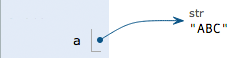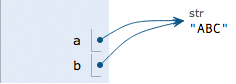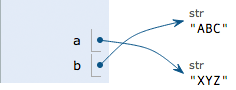#### 常量

``````PI = 3.14159265359
``````

``````>>> 10 / 3
3.3333333333333335
``````

`/`除法计算结果是浮点数，即使是两个整数恰好整除，结果也是浮点数：

``````>>> 9 / 3
3.0
``````

``````>>> 10 // 3
3
``````

``````>>> 10 % 3
1
``````

#### 练习

```n = 123
f = 456.789
s1 = 'Hello, world'
s3 = r'Hello, "Bart"'
s4 = r'''Hello,
Lisa!'''
```

#### 小结

Python支持多种数据类型，在计算机内部，可以把任何数据都看成一个“对象”，而变量就是在程序中用来指向这些数据对象的，对变量赋值就是把数据和变量给关联起来。

Python的浮点数也没有大小限制，但是超出一定范围就直接表示为`inf`（无限大）。

## 字符串和编码

#### 字符编码Unicode标准也在不断发展，但最常用的是用两个字节表示一个字符（如果要用到非常偏僻的字符，就需要4个字节）。现代操作系统和大多数编程语言都直接支持Unicode。

A0100000100000000 0100000101000001
x01001110 0010110111100100 10111000 10101101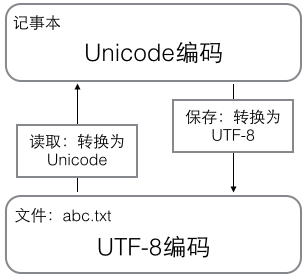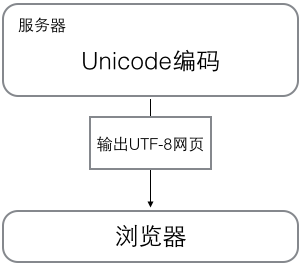#### Python的字符串

``````>>> print('包含中文的str')

``````

``````>>> ord('A')
65
>>> ord('中')
20013
>>> chr(66)
'B'
>>> chr(25991)
'文'
``````

``````>>> '\u4e2d\u6587'
'中文'
``````

Python对`bytes`类型的数据用带`b`前缀的单引号或双引号表示：

``````x = b'ABC'
``````

``````>>> 'ABC'.encode('ascii')
b'ABC'
>>> '中文'.encode('utf-8')
>>> '中文'.encode('ascii')
Traceback (most recent call last):
File "<stdin>", line 1, in <module>
UnicodeEncodeError: 'ascii' codec can't encode characters in position 0-1: ordinal not in range(128)
``````

`bytes`中，无法显示为ASCII字符的字节，用`\x##`显示。

``````>>> b'ABC'.decode('ascii')
'ABC'
'中文'
``````

``````>>> len('ABC')
3
>>> len('中文')
2
``````

`len()`函数计算的是`str`的字符数，如果换成`bytes``len()`函数就计算字节数：

``````>>> len(b'ABC')
3
6
>>> len('中文'.encode('utf-8'))
6
``````

``````#!/usr/bin/env python3
# -*- coding: utf-8 -*-
``````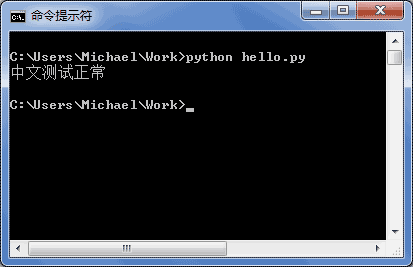#### 格式化``````>>> 'Hello, %s' % 'world'
'Hello, world'
>>> 'Hi, %s, you have \$%d.' % ('Michael', 1000000)
'Hi, Michael, you have \$1000000.'
``````

 %d 整数 %f 浮点数 %s 字符串 %x 十六进制整数

``````>>> '%2d-%02d' % (3, 1)
' 3-01'
>>> '%.2f' % 3.1415926
'3.14'
``````

``````>>> 'Age: %s. Gender: %s' % (25, True)
'Age: 25. Gender: True'
``````

``````>>> 'growth rate: %d %%' % 7
'growth rate: 7 %'
``````

#### 练习

```# -*- coding: utf-8 -*-

s1 = 72
s2 = 85
----
r = ???
print('???' % r)
```

#### 小结

Python 3的字符串使用Unicode，直接支持多语言。

str和bytes互相转换时，需要指定编码。最常用的编码是UTF-8。Python当然也支持其他编码方式，比如把Unicode编码成GB2312：

``````>>> '中文'.encode('gb2312')
'\xd6\xd0\xce\xc4'
``````

the_string.py

## 使用list和tuple

#### list

Python内置的一种数据类型是列表：list。list是一种有序的集合，可以随时添加和删除其中的元素。

``````>>> classmates = ['Michael', 'Bob', 'Tracy']
>>> classmates
['Michael', 'Bob', 'Tracy']
``````

``````>>> len(classmates)
3
``````

``````>>> classmates
'Michael'
>>> classmates
'Bob'
>>> classmates
'Tracy'
>>> classmates
Traceback (most recent call last):
File "<stdin>", line 1, in <module>
IndexError: list index out of range
``````

``````>>> classmates[-1]
'Tracy'
``````

``````>>> classmates[-2]
'Bob'
>>> classmates[-3]
'Michael'
>>> classmates[-4]
Traceback (most recent call last):
File "<stdin>", line 1, in <module>
IndexError: list index out of range
``````

list是一个可变的有序表，所以，可以往list中追加元素到末尾：

``````>>> classmates.append('Adam')
>>> classmates
``````

``````>>> classmates.insert(1, 'Jack')
>>> classmates
``````

``````>>> classmates.pop()
>>> classmates
['Michael', 'Jack', 'Bob', 'Tracy']
``````

``````>>> classmates.pop(1)
'Jack'
>>> classmates
['Michael', 'Bob', 'Tracy']
``````

``````>>> classmates = 'Sarah'
>>> classmates
['Michael', 'Sarah', 'Tracy']
``````

list里面的元素的数据类型也可以不同，比如：

``````>>> L = ['Apple', 123, True]
``````

list元素也可以是另一个list，比如：

``````>>> s = ['python', 'java', ['asp', 'php'], 'scheme']
>>> len(s)
4
``````

``````>>> p = ['asp', 'php']
>>> s = ['python', 'java', p, 'scheme']
``````

``````>>> L = []
>>> len(L)
0
``````

#### tuple

``````>>> classmates = ('Michael', 'Bob', 'Tracy')
``````

tuple的陷阱：当你定义一个tuple时，在定义的时候，tuple的元素就必须被确定下来，比如：

``````>>> t = (1, 2)
>>> t
(1, 2)
``````

``````>>> t = ()
>>> t
()
``````

``````>>> t = (1)
>>> t
1
``````

``````>>> t = (1,)
>>> t
(1,)
``````

Python在显示只有1个元素的tuple时，也会加一个逗号`,`，以免你误解成数学计算意义上的括号。

``````>>> t = ('a', 'b', ['A', 'B'])
>>> t = 'X'
>>> t = 'Y'
>>> t
('a', 'b', ['X', 'Y'])
``````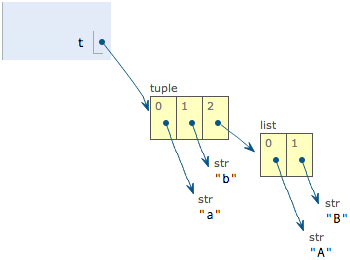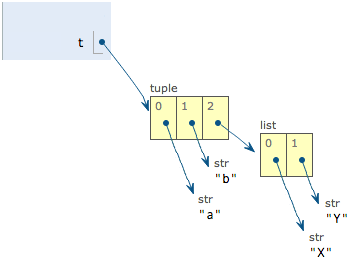#### 练习

```# -*- coding: utf-8 -*-

L = [
['Java', 'Python', 'Ruby', 'PHP'],
]
----
# 打印Apple:
print(?)
# 打印Python:
print(?)
# 打印Lisa:
print(?)
```

#### 小结

list和tuple是Python内置的有序集合，一个可变，一个不可变。根据需要来选择使用它们。

the_list.py

the_tuple.py

## 条件判断

#### 条件判断

``````age = 20
if age >= 18:
``````

``````age = 3
if age >= 18:
else:
print('teenager')
``````

``````age = 3
if age >= 18:
elif age >= 6:
print('teenager')
else:
print('kid')
``````

`elif``else if`的缩写，完全可以有多个`elif`，所以`if`语句的完整形式就是：

``````if <条件判断1>:
<执行1>
elif <条件判断2>:
<执行2>
elif <条件判断3>:
<执行3>
else:
<执行4>
``````

`if`语句执行有个特点，它是从上往下判断，如果在某个判断上是`True`，把该判断对应的语句执行后，就忽略掉剩下的`elif``else`，所以，请测试并解释为什么下面的程序打印的是`teenager`

``````age = 20
if age >= 6:
print('teenager')
elif age >= 18:
else:
print('kid')
``````

`if`判断条件还可以简写，比如写：

``````if x:
print('True')
``````

#### 再议 input

``````birth = input('birth: ')
if birth < 2000:
print('00前')
else:
print('00后')
``````

``````Traceback (most recent call last):
File "<stdin>", line 1, in <module>
TypeError: unorderable types: str() > int()
``````

``````s = input('birth: ')
birth = int(s)
if birth < 2000:
print('00前')
else:
print('00后')
``````

``````Traceback (most recent call last):
File "<stdin>", line 1, in <module>
ValueError: invalid literal for int() with base 10: 'abc'
``````

#### 练习

• 低于18.5：过轻
• 18.5-25：正常
• 25-28：过重
• 28-32：肥胖
• 高于32：严重肥胖

`if-elif`判断并打印结果：

```# -*- coding: utf-8 -*-

height = 1.75
weight = 80.5
----
bmi = ???
if ???:
pass
```

#### 小结do_if.py

## 循环

#### 循环

``````>>> 1 + 2 + 3
6
``````

Python的循环有两种，一种是for...in循环，依次把list或tuple中的每个元素迭代出来，看例子：

``````names = ['Michael', 'Bob', 'Tracy']
for name in names:
print(name)
``````

``````Michael
Bob
Tracy
``````

``````sum = 0
for x in [1, 2, 3, 4, 5, 6, 7, 8, 9, 10]:
sum = sum + x
print(sum)
``````

``````>>> list(range(5))
[0, 1, 2, 3, 4]
``````

`range(101)`就可以生成0-100的整数序列，计算如下：

``````sum = 0
for x in range(101):
sum = sum + x
print(sum)
``````

``````sum = 0
n = 99
while n > 0:
sum = sum + n
n = n - 2
print(sum)
``````

#### 练习

```# -*- coding: utf-8 -*-
```

do_for.py

do_while.py

## 使用dict和set

#### dict

Python内置了字典：dict的支持，dict全称dictionary，在其他语言中也称为map，使用键-值（key-value）存储，具有极快的查找速度。

``````names = ['Michael', 'Bob', 'Tracy']
scores = [95, 75, 85]
``````

``````>>> d = {'Michael': 95, 'Bob': 75, 'Tracy': 85}
>>> d['Michael']
95
``````

dict就是第二种实现方式，给定一个名字，比如`'Michael'`，dict在内部就可以直接计算出`Michael`对应的存放成绩的“页码”，也就是`95`这个数字存放的内存地址，直接取出来，所以速度非常快。

``````>>> d['Adam'] = 67
67
``````

``````>>> d['Jack'] = 90
>>> d['Jack']
90
>>> d['Jack'] = 88
>>> d['Jack']
88
``````

``````>>> d['Thomas']
Traceback (most recent call last):
File "<stdin>", line 1, in <module>
KeyError: 'Thomas'
``````

``````>>> 'Thomas' in d
False
``````

``````>>> d.get('Thomas')
>>> d.get('Thomas', -1)
-1
``````

``````>>> d.pop('Bob')
75
>>> d
{'Michael': 95, 'Tracy': 85}
``````

1. 查找和插入的速度极快，不会随着key的增加而增加；
2. 需要占用大量的内存，内存浪费多。

1. 查找和插入的时间随着元素的增加而增加；
2. 占用空间小，浪费内存很少。

dict可以用在需要高速查找的很多地方，在Python代码中几乎无处不在，正确使用dict非常重要，需要牢记的第一条就是dict的key必须是不可变对象

``````>>> key = [1, 2, 3]
>>> d[key] = 'a list'
Traceback (most recent call last):
File "<stdin>", line 1, in <module>
TypeError: unhashable type: 'list'
``````

#### set

set和dict类似，也是一组key的集合，但不存储value。由于key不能重复，所以，在set中，没有重复的key。

``````>>> s = set([1, 2, 3])
>>> s
{1, 2, 3}
``````

``````>>> s = set([1, 1, 2, 2, 3, 3])
>>> s
{1, 2, 3}
``````

``````>>> s.add(4)
>>> s
{1, 2, 3, 4}
>>> s
{1, 2, 3, 4}
``````

``````>>> s.remove(4)
>>> s
{1, 2, 3}
``````

set可以看成数学意义上的无序和无重复元素的集合，因此，两个set可以做数学意义上的交集、并集等操作：

``````>>> s1 = set([1, 2, 3])
>>> s2 = set([2, 3, 4])
>>> s1 & s2
{2, 3}
>>> s1 | s2
{1, 2, 3, 4}
``````

set和dict的唯一区别仅在于没有存储对应的value，但是，set的原理和dict一样，所以，同样不可以放入可变对象，因为无法判断两个可变对象是否相等，也就无法保证set内部“不会有重复元素”。试试把list放入set，看看是否会报错。

#### 再议不可变对象

``````>>> a = ['c', 'b', 'a']
>>> a.sort()
>>> a
['a', 'b', 'c']
``````

``````>>> a = 'abc'
>>> a.replace('a', 'A')
'Abc'
>>> a
'abc'
``````

``````>>> a = 'abc'
>>> b = a.replace('a', 'A')
>>> b
'Abc'
>>> a
'abc'
``````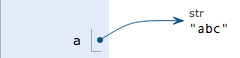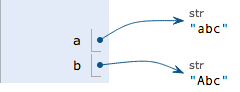#### 小结

tuple虽然是不变对象，但试试把`(1, 2, 3)``(1, [2, 3])`放入dict或set中，并解释结果。

the_dict.py

the_set.py

# 函数

S = πr2

``````r1 = 12.34
r2 = 9.08
r3 = 73.1
s1 = 3.14 * r1 * r1
s2 = 3.14 * r2 * r2
s3 = 3.14 * r3 * r3
``````

#### 抽象

100

n

n=1

100

(n2+1)

n=1

(1 x 1 + 1) + (2 x 2 + 1) + (3 x 3 + 1) + ... + (100 x 100 + 1)

## 调用函数

Python内置了很多有用的函数，我们可以直接调用。

http://docs.python.org/3/library/functions.html#abs

``````>>> abs(100)
100
>>> abs(-20)
20
>>> abs(12.34)
12.34
``````

``````>>> abs(1, 2)
Traceback (most recent call last):
File "<stdin>", line 1, in <module>
TypeError: abs() takes exactly one argument (2 given)
``````

``````>>> abs('a')
Traceback (most recent call last):
File "<stdin>", line 1, in <module>
TypeError: bad operand type for abs(): 'str'
``````

`max`函数`max()`可以接收任意多个参数，并返回最大的那个：

``````>>> max(1, 2)
2
>>> max(2, 3, 1, -5)
3
``````

#### 数据类型转换

Python内置的常用函数还包括数据类型转换函数，比如`int()`函数可以把其他数据类型转换为整数：

``````>>> int('123')
123
>>> int(12.34)
12
>>> float('12.34')
12.34
>>> str(1.23)
'1.23'
>>> str(100)
'100'
>>> bool(1)
True
>>> bool('')
False
``````

``````>>> a = abs # 变量a指向abs函数
>>> a(-1) # 所以也可以通过a调用abs函数
1
``````

#### 练习

```# -*- coding: utf-8 -*-

n1 = 255
n2 = 1000
----
print(???)
```

call_func.py

## 定义函数

``````def my_abs(x):
if x >= 0:
return x
else:
return -x
``````

`return None`可以简写为`return`

`import`的用法在后续模块一节中会详细介绍。

#### 空函数

``````def nop():
pass
``````

`pass`语句什么都不做，那有什么用？实际上`pass`可以用来作为占位符，比如现在还没想好怎么写函数的代码，就可以先放一个`pass`，让代码能运行起来。

`pass`还可以用在其他语句里，比如：

``````if age >= 18:
pass
``````

#### 参数检查

``````>>> my_abs(1, 2)
Traceback (most recent call last):
File "<stdin>", line 1, in <module>
TypeError: my_abs() takes 1 positional argument but 2 were given
``````

``````>>> my_abs('A')
Traceback (most recent call last):
File "<stdin>", line 1, in <module>
File "<stdin>", line 2, in my_abs
TypeError: unorderable types: str() >= int()
>>> abs('A')
Traceback (most recent call last):
File "<stdin>", line 1, in <module>
TypeError: bad operand type for abs(): 'str'
``````

``````def my_abs(x):
if not isinstance(x, (int, float)):
if x >= 0:
return x
else:
return -x
``````

``````>>> my_abs('A')
Traceback (most recent call last):
File "<stdin>", line 1, in <module>
File "<stdin>", line 3, in my_abs
``````

#### 返回多个值

``````import math

def move(x, y, step, angle=0):
nx = x + step * math.cos(angle)
ny = y - step * math.sin(angle)
return nx, ny
``````

`import math`语句表示导入`math`包，并允许后续代码引用`math`包里的`sin``cos`等函数。

``````>>> x, y = move(100, 100, 60, math.pi / 6)
>>> print(x, y)
151.96152422706632 70.0
``````

``````>>> r = move(100, 100, 60, math.pi / 6)
>>> print(r)
(151.96152422706632, 70.0)
``````

#### 练习

ax2 + bx + c = 0

``````>>> import math
>>> math.sqrt(2)
1.4142135623730951
``````
```# -*- coding: utf-8 -*-

import math

----
pass
----
# 测试:
print(quadratic(2, 3, 1)) # => (-0.5, -1.0)
print(quadratic(1, 3, -4)) # => (1.0, -4.0)
```

def_func.py

## 函数的参数

Python的函数定义非常简单，但灵活度却非常大。除了正常定义的必选参数外，还可以使用默认参数、可变参数和关键字参数，使得函数定义出来的接口，不但能处理复杂的参数，还可以简化调用者的代码。

#### 位置参数

``````def power(x):
return x * x
``````

``````>>> power(5)
25
>>> power(15)
225
``````

``````def power(x, n):
s = 1
while n > 0:
n = n - 1
s = s * x
return s
``````

``````>>> power(5, 2)
25
>>> power(5, 3)
125
``````

#### 默认参数

``````>>> power(5)
Traceback (most recent call last):
File "<stdin>", line 1, in <module>
TypeError: power() missing 1 required positional argument: 'n'
``````

Python的错误信息很明确：调用函数`power()`缺少了一个位置参数`n`

``````def power(x, n=2):
s = 1
while n > 0:
n = n - 1
s = s * x
return s
``````

``````>>> power(5)
25
>>> power(5, 2)
25
``````

``````def enroll(name, gender):
print('name:', name)
print('gender:', gender)
``````

``````>>> enroll('Sarah', 'F')
name: Sarah
gender: F
``````

``````def enroll(name, gender, age=6, city='Beijing'):
print('name:', name)
print('gender:', gender)
print('age:', age)
print('city:', city)
``````

``````>>> enroll('Sarah', 'F')
name: Sarah
gender: F
age: 6
city: Beijing
``````

``````enroll('Bob', 'M', 7)
``````

``````def add_end(L=[]):
L.append('END')
return L
``````

``````>>> add_end([1, 2, 3])
[1, 2, 3, 'END']
['x', 'y', 'z', 'END']
``````

``````>>> add_end()
['END']
``````

``````>>> add_end()
['END', 'END']
['END', 'END', 'END']
``````

Python函数在定义的时候，默认参数`L`的值就被计算出来了，即`[]`，因为默认参数`L`也是一个变量，它指向对象`[]`，每次调用该函数，如果改变了`L`的内容，则下次调用时，默认参数的内容就变了，不再是函数定义时的`[]`了。

``````def add_end(L=None):
if L is None:
L = []
L.append('END')
return L
``````

``````>>> add_end()
['END']
['END']
``````

#### 可变参数

``````def calc(numbers):
sum = 0
for n in numbers:
sum = sum + n * n
return sum
``````

``````>>> calc([1, 2, 3])
14
>>> calc((1, 3, 5, 7))
84
``````

``````>>> calc(1, 2, 3)
14
>>> calc(1, 3, 5, 7)
84
``````

``````def calc(*numbers):
sum = 0
for n in numbers:
sum = sum + n * n
return sum
``````

``````>>> calc(1, 2)
5
>>> calc()
0
``````

``````>>> nums = [1, 2, 3]
>>> calc(nums, nums, nums)
14
``````

``````>>> nums = [1, 2, 3]
>>> calc(*nums)
14
``````

`*nums`表示把`nums`这个list的所有元素作为可变参数传进去。这种写法相当有用，而且很常见。

#### 关键字参数

``````def person(name, age, **kw):
print('name:', name, 'age:', age, 'other:', kw)
``````

``````>>> person('Michael', 30)
name: Michael age: 30 other: {}
``````

``````>>> person('Bob', 35, city='Beijing')
name: Bob age: 35 other: {'city': 'Beijing'}
name: Adam age: 45 other: {'gender': 'M', 'job': 'Engineer'}
``````

``````>>> extra = {'city': 'Beijing', 'job': 'Engineer'}
>>> person('Jack', 24, city=extra['city'], job=extra['job'])
name: Jack age: 24 other: {'city': 'Beijing', 'job': 'Engineer'}
``````

``````>>> extra = {'city': 'Beijing', 'job': 'Engineer'}
>>> person('Jack', 24, **extra)
name: Jack age: 24 other: {'city': 'Beijing', 'job': 'Engineer'}
``````

`**extra`表示把`extra`这个dict的所有key-value用关键字参数传入到函数的`**kw`参数，`kw`将获得一个dict，注意`kw`获得的dict是`extra`的一份拷贝，对`kw`的改动不会影响到函数外的`extra`

#### 命名关键字参数

``````def person(name, age, **kw):
if 'city' in kw:
# 有city参数
pass
if 'job' in kw:
# 有job参数
pass
print('name:', name, 'age:', age, 'other:', kw)
``````

``````>>> person('Jack', 24, city='Beijing', addr='Chaoyang', zipcode=123456)
``````

``````def person(name, age, *, city, job):
print(name, age, city, job)
``````

``````>>> person('Jack', 24, city='Beijing', job='Engineer')
Jack 24 Beijing Engineer
``````

``````>>> person('Jack', 24, 'Beijing', 'Engineer')
Traceback (most recent call last):
File "<stdin>", line 1, in <module>
TypeError: person() takes 2 positional arguments but 4 were given
``````

``````def person(name, age, *, city='Beijing', job):
print(name, age, city, job)
``````

``````>>> person('Jack', 24, job='Engineer')
Jack 24 Beijing Engineer
``````

``````def person(name, age, city, job):
# 缺少 *，city和job被视为位置参数
pass
``````

#### 参数组合

``````def f1(a, b, c=0, *args, **kw):
print('a =', a, 'b =', b, 'c =', c, 'args =', args, 'kw =', kw)

def f2(a, b, c=0, *, d, **kw):
print('a =', a, 'b =', b, 'c =', c, 'd =', d, 'kw =', kw)
``````

``````>>> f1(1, 2)
a = 1 b = 2 c = 0 args = () kw = {}
>>> f1(1, 2, c=3)
a = 1 b = 2 c = 3 args = () kw = {}
>>> f1(1, 2, 3, 'a', 'b')
a = 1 b = 2 c = 3 args = ('a', 'b') kw = {}
>>> f1(1, 2, 3, 'a', 'b', x=99)
a = 1 b = 2 c = 3 args = ('a', 'b') kw = {'x': 99}
>>> f2(1, 2, d=99, ext=None)
a = 1 b = 2 c = 0 d = 99 kw = {'ext': None}
``````

``````>>> args = (1, 2, 3, 4)
>>> kw = {'d': 99, 'x': '#'}
>>> f1(*args, **kw)
a = 1 b = 2 c = 3 args = (4,) kw = {'d': 99, 'x': '#'}
>>> args = (1, 2, 3)
>>> kw = {'d': 88, 'x': '#'}
>>> f2(*args, **kw)
a = 1 b = 2 c = 3 d = 88 kw = {'x': '#'}
``````

#### 小结

Python的函数具有非常灵活的参数形态，既可以实现简单的调用，又可以传入非常复杂的参数。

`*args`是可变参数，args接收的是一个tuple；

`**kw`是关键字参数，kw接收的是一个dict。

var_args.py

kw_args.py

## 递归函数

fact(n) = n! = 1 x 2 x 3 x ... x (n-1) x n = (n-1)! x n = fact(n-1) x n

``````def fact(n):
if n==1:
return 1
return n * fact(n - 1)
``````

``````>>> fact(1)
1
>>> fact(5)
120
>>> fact(100)
93326215443944152681699238856266700490715968264381621468592963895217599993229915608941463976156518286253697920827223758251185210916864000000000000000000000000
``````

``````===> fact(5)
===> 5 * fact(4)
===> 5 * (4 * fact(3))
===> 5 * (4 * (3 * fact(2)))
===> 5 * (4 * (3 * (2 * fact(1))))
===> 5 * (4 * (3 * (2 * 1)))
===> 5 * (4 * (3 * 2))
===> 5 * (4 * 6)
===> 5 * 24
===> 120
``````

``````>>> fact(1000)
Traceback (most recent call last):
File "<stdin>", line 1, in <module>
File "<stdin>", line 4, in fact
...
File "<stdin>", line 4, in fact
RuntimeError: maximum recursion depth exceeded in comparison
``````

``````def fact(n):
return fact_iter(n, 1)

def fact_iter(num, product):
if num == 1:
return product
return fact_iter(num - 1, num * product)
``````

`fact(5)`对应的`fact_iter(5, 1)`的调用如下：

``````===> fact_iter(5, 1)
===> fact_iter(4, 5)
===> fact_iter(3, 20)
===> fact_iter(2, 60)
===> fact_iter(1, 120)
===> 120
``````

#### 小结

Python标准的解释器没有针对尾递归做优化，任何递归函数都存在栈溢出的问题。

#### 练习

```def move(n, a, b, c):
----
pass
----
# 期待输出:
# A --> C
# A --> B
# C --> B
# A --> C
# B --> A
# B --> C
# A --> C
move(3, 'A', 'B', 'C')
```

recur.py

# 高级特性

``````L = []
n = 1
while n <= 99:
L.append(n)
n = n + 2
``````

## 切片

``````>>> L = ['Michael', 'Sarah', 'Tracy', 'Bob', 'Jack']
``````

``````>>> [L, L, L]
['Michael', 'Sarah', 'Tracy']
``````

``````>>> r = []
>>> n = 3
>>> for i in range(n):
...     r.append(L[i])
...
>>> r
['Michael', 'Sarah', 'Tracy']
``````

``````>>> L[0:3]
['Michael', 'Sarah', 'Tracy']
``````

`L[0:3]`表示，从索引`0`开始取，直到索引`3`为止，但不包括索引`3`。即索引`0``1``2`，正好是3个元素。

``````>>> L[:3]
['Michael', 'Sarah', 'Tracy']
``````

``````>>> L[1:3]
['Sarah', 'Tracy']
``````

``````>>> L[-2:]
['Bob', 'Jack']
>>> L[-2:-1]
['Bob']
``````

``````>>> L = list(range(100))
>>> L
[0, 1, 2, 3, ..., 99]
``````

``````>>> L[:10]
[0, 1, 2, 3, 4, 5, 6, 7, 8, 9]
``````

``````>>> L[-10:]
[90, 91, 92, 93, 94, 95, 96, 97, 98, 99]
``````

``````>>> L[10:20]
[10, 11, 12, 13, 14, 15, 16, 17, 18, 19]
``````

``````>>> L[:10:2]
[0, 2, 4, 6, 8]
``````

``````>>> L[::5]
[0, 5, 10, 15, 20, 25, 30, 35, 40, 45, 50, 55, 60, 65, 70, 75, 80, 85, 90, 95]
``````

``````>>> L[:]
[0, 1, 2, 3, ..., 99]
``````

tuple也是一种list，唯一区别是tuple不可变。因此，tuple也可以用切片操作，只是操作的结果仍是tuple：

``````>>> (0, 1, 2, 3, 4, 5)[:3]
(0, 1, 2)
``````

``````>>> 'ABCDEFG'[:3]
'ABC'
>>> 'ABCDEFG'[::2]
'ACEG'
``````

do_slice.py

## 迭代

``````for (i=0; i<list.length; i++) {
n = list[i];
}
``````

list这种数据类型虽然有下标，但很多其他数据类型是没有下标的，但是，只要是可迭代对象，无论有无下标，都可以迭代，比如dict就可以迭代：

``````>>> d = {'a': 1, 'b': 2, 'c': 3}
>>> for key in d:
...     print(key)
...
a
c
b
``````

``````>>> for ch in 'ABC':
...     print(ch)
...
A
B
C
``````

``````>>> from collections import Iterable
>>> isinstance('abc', Iterable) # str是否可迭代
True
>>> isinstance([1,2,3], Iterable) # list是否可迭代
True
>>> isinstance(123, Iterable) # 整数是否可迭代
False
``````

``````>>> for i, value in enumerate(['A', 'B', 'C']):
...     print(i, value)
...
0 A
1 B
2 C
``````

``````>>> for x, y in [(1, 1), (2, 4), (3, 9)]:
...     print(x, y)
...
1 1
2 4
3 9
``````

do_iter.py

## 列表生成式

``````>>> list(range(1, 11))
[1, 2, 3, 4, 5, 6, 7, 8, 9, 10]
``````

``````>>> L = []
>>> for x in range(1, 11):
...    L.append(x * x)
...
>>> L
[1, 4, 9, 16, 25, 36, 49, 64, 81, 100]
``````

``````>>> [x * x for x in range(1, 11)]
[1, 4, 9, 16, 25, 36, 49, 64, 81, 100]
``````

for循环后面还可以加上if判断，这样我们就可以筛选出仅偶数的平方：

``````>>> [x * x for x in range(1, 11) if x % 2 == 0]
[4, 16, 36, 64, 100]
``````

``````>>> [m + n for m in 'ABC' for n in 'XYZ']
['AX', 'AY', 'AZ', 'BX', 'BY', 'BZ', 'CX', 'CY', 'CZ']
``````

``````>>> import os # 导入os模块，模块的概念后面讲到
>>> [d for d in os.listdir('.')] # os.listdir可以列出文件和目录
['.emacs.d', '.ssh', '.Trash', 'Adlm', 'Applications', 'Desktop', 'Documents', 'Downloads', 'Library', 'Movies', 'Music', 'Pictures', 'Public', 'VirtualBox VMs', 'Workspace', 'XCode']
``````

`for`循环其实可以同时使用两个甚至多个变量，比如`dict``items()`可以同时迭代key和value：

``````>>> d = {'x': 'A', 'y': 'B', 'z': 'C' }
>>> for k, v in d.items():
...     print(k, '=', v)
...
y = B
x = A
z = C
``````

``````>>> d = {'x': 'A', 'y': 'B', 'z': 'C' }
>>> [k + '=' + v for k, v in d.items()]
['y=B', 'x=A', 'z=C']
``````

``````>>> L = ['Hello', 'World', 'IBM', 'Apple']
>>> [s.lower() for s in L]
['hello', 'world', 'ibm', 'apple']
``````

#### 练习

``````>>> L = ['Hello', 'World', 18, 'Apple', None]
>>> [s.lower() for s in L]
Traceback (most recent call last):
File "<stdin>", line 1, in <module>
File "<stdin>", line 1, in <listcomp>
AttributeError: 'int' object has no attribute 'lower'
``````

``````>>> x = 'abc'
>>> y = 123
>>> isinstance(x, str)
True
>>> isinstance(y, str)
False
``````

```# -*- coding: utf-8 -*-

L1 = ['Hello', 'World', 18, 'Apple', None]
----
L2 = ???
----
# 期待输出: ['hello', 'world', 'apple']
print(L2)
```

do_listcompr.py

## 生成器

``````>>> L = [x * x for x in range(10)]
>>> L
[0, 1, 4, 9, 16, 25, 36, 49, 64, 81]
>>> g = (x * x for x in range(10))
>>> g
<generator object <genexpr> at 0x1022ef630>
``````

``````>>> next(g)
0
>>> next(g)
1
>>> next(g)
4
>>> next(g)
9
>>> next(g)
16
>>> next(g)
25
>>> next(g)
36
>>> next(g)
49
>>> next(g)
64
>>> next(g)
81
>>> next(g)
Traceback (most recent call last):
File "<stdin>", line 1, in <module>
StopIteration
``````

``````>>> g = (x * x for x in range(10))
>>> for n in g:
...     print(n)
...
0
1
4
9
16
25
36
49
64
81
``````

generator非常强大。如果推算的算法比较复杂，用类似列表生成式的`for`循环无法实现的时候，还可以用函数来实现。

1, 1, 2, 3, 5, 8, 13, 21, 34, ...

``````def fib(max):
n, a, b = 0, 0, 1
while n < max:
print(b)
a, b = b, a + b
n = n + 1
return 'done'
``````

``````>>> fib(6)
1
1
2
3
5
8
'done'
``````

``````def fib(max):
n, a, b = 0, 0, 1
while n < max:
yield b
a, b = b, a + b
n = n + 1
return 'done'
``````

``````>>> f = fib(6)
>>> f
<generator object fib at 0x104feaaa0>
``````

``````def odd():
print('step 1')
yield 1
print('step 2')
yield(3)
print('step 3')
yield(5)
``````

``````>>> o = odd()
>>> next(o)
step 1
1
>>> next(o)
step 2
3
>>> next(o)
step 3
5
>>> next(o)
Traceback (most recent call last):
File "<stdin>", line 1, in <module>
StopIteration
``````

``````>>> for n in fib(6):
...     print(n)
...
1
1
2
3
5
8
``````

``````>>> g = fib(6)
>>> while True:
...     try:
...         x = next(g)
...         print('g:', x)
...     except StopIteration as e:
...         print('Generator return value:', e.value)
...         break
...
g: 1
g: 1
g: 2
g: 3
g: 5
g: 8
Generator return value: done
``````

#### 练习

``````          1
1   1
1   2   1
1   3   3   1
1   4   6   4   1
1   5   10  10  5   1
``````

```# -*- coding: utf-8 -*-

def triangles():
----
pass
----
# 期待输出:
# 
# [1, 1]
# [1, 2, 1]
# [1, 3, 3, 1]
# [1, 4, 6, 4, 1]
# [1, 5, 10, 10, 5, 1]
# [1, 6, 15, 20, 15, 6, 1]
# [1, 7, 21, 35, 35, 21, 7, 1]
# [1, 8, 28, 56, 70, 56, 28, 8, 1]
# [1, 9, 36, 84, 126, 126, 84, 36, 9, 1]
n = 0
for t in triangles():
print(t)
n = n + 1
if n == 10:
break
```

#### 小结

generator是非常强大的工具，在Python中，可以简单地把列表生成式改成generator，也可以通过函数实现复杂逻辑的generator。

``````>>> r = abs(6)
>>> r
6
``````

generator函数的“调用”实际返回一个generator对象：

``````>>> g = fib(6)
>>> g
<generator object fib at 0x1022ef948>
``````

do_generator.py

## 迭代器

``````>>> from collections import Iterable
>>> isinstance([], Iterable)
True
>>> isinstance({}, Iterable)
True
>>> isinstance('abc', Iterable)
True
>>> isinstance((x for x in range(10)), Iterable)
True
>>> isinstance(100, Iterable)
False
``````

``````>>> from collections import Iterator
>>> isinstance((x for x in range(10)), Iterator)
True
>>> isinstance([], Iterator)
False
>>> isinstance({}, Iterator)
False
>>> isinstance('abc', Iterator)
False
``````

`list``dict``str``Iterable`变成`Iterator`可以使用`iter()`函数：

``````>>> isinstance(iter([]), Iterator)
True
>>> isinstance(iter('abc'), Iterator)
True
``````

`Iterator`甚至可以表示一个无限大的数据流，例如全体自然数。而使用list是永远不可能存储全体自然数的。

#### 小结

Python的`for`循环本质上就是通过不断调用`next()`函数实现的，例如：

``````for x in [1, 2, 3, 4, 5]:
pass
``````

``````# 首先获得Iterator对象:
it = iter([1, 2, 3, 4, 5])
# 循环:
while True:
try:
# 获得下一个值:
x = next(it)
except StopIteration:
# 遇到StopIteration就退出循环
break
``````

do_iter.py

# 函数式编程

Python对函数式编程提供部分支持。由于Python允许使用变量，因此，Python不是纯函数式编程语言。

## 高阶函数

#### 变量可以指向函数

``````>>> abs(-10)
10
``````

``````>>> abs
<built-in function abs>
``````

``````>>> x = abs(-10)
>>> x
10
``````

``````>>> f = abs
>>> f
<built-in function abs>
``````

``````>>> f = abs
>>> f(-10)
10
``````

#### 函数名也是变量

``````>>> abs = 10
>>> abs(-10)
Traceback (most recent call last):
File "<stdin>", line 1, in <module>
TypeError: 'int' object is not callable
``````

`abs`指向`10`后，就无法通过`abs(-10)`调用该函数了！因为`abs`这个变量已经不指向求绝对值函数而是指向一个整数`10`

#### 传入函数

``````def add(x, y, f):
return f(x) + f(y)
``````

``````x = -5
y = 6
f = abs
f(x) + f(y) ==> abs(-5) + abs(6) ==> 11
return 11
``````

``````>>> add(-5, 6, abs)
11
``````

### map/reduce

Python内建了`map()``reduce()`函数。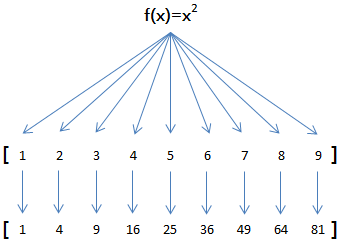``````>>> def f(x):
...     return x * x
...
>>> r = map(f, [1, 2, 3, 4, 5, 6, 7, 8, 9])
>>> list(r)
[1, 4, 9, 16, 25, 36, 49, 64, 81]
``````

`map()`传入的第一个参数是`f`，即函数对象本身。由于结果`r`是一个`Iterator``Iterator`是惰性序列，因此通过`list()`函数让它把整个序列都计算出来并返回一个list。

``````L = []
for n in [1, 2, 3, 4, 5, 6, 7, 8, 9]:
L.append(f(n))
print(L)
``````

``````>>> list(map(str, [1, 2, 3, 4, 5, 6, 7, 8, 9]))
['1', '2', '3', '4', '5', '6', '7', '8', '9']
``````

``````reduce(f, [x1, x2, x3, x4]) = f(f(f(x1, x2), x3), x4)
``````

``````>>> from functools import reduce
...     return x + y
...
>>> reduce(add, [1, 3, 5, 7, 9])
25
``````

``````>>> from functools import reduce
>>> def fn(x, y):
...     return x * 10 + y
...
>>> reduce(fn, [1, 3, 5, 7, 9])
13579
``````

``````>>> from functools import reduce
>>> def fn(x, y):
...     return x * 10 + y
...
>>> def char2num(s):
...     return {'0': 0, '1': 1, '2': 2, '3': 3, '4': 4, '5': 5, '6': 6, '7': 7, '8': 8, '9': 9}[s]
...
>>> reduce(fn, map(char2num, '13579'))
13579
``````

``````from functools import reduce

def str2int(s):
def fn(x, y):
return x * 10 + y
def char2num(s):
return {'0': 0, '1': 1, '2': 2, '3': 3, '4': 4, '5': 5, '6': 6, '7': 7, '8': 8, '9': 9}[s]
return reduce(fn, map(char2num, s))
``````

``````from functools import reduce

def char2num(s):
return {'0': 0, '1': 1, '2': 2, '3': 3, '4': 4, '5': 5, '6': 6, '7': 7, '8': 8, '9': 9}[s]

def str2int(s):
return reduce(lambda x, y: x * 10 + y, map(char2num, s))
``````

lambda函数的用法在后面介绍。

#### 练习

```# -*- coding: utf-8 -*-
----
def normalize(name):
pass
----
# 测试:
L2 = list(map(normalize, L1))
print(L2)
```

Python提供的`sum()`函数可以接受一个list并求和，请编写一个`prod()`函数，可以接受一个list并利用`reduce()`求积：

```# -*- coding: utf-8 -*-

from functools import reduce

def prod(L):
----
pass
----
print('3 * 5 * 7 * 9 =', prod([3, 5, 7, 9]))
```

```# -*- coding: utf-8 -*-

from functools import reduce

def str2float(s):
----
pass
----
print('str2float(\'123.456\') =', str2float('123.456'))
```

do_map.py

do_reduce.py

### filter

Python内建的`filter()`函数用于过滤序列。

`map()`类似，`filter()`也接收一个函数和一个序列。和`map()`不同的时，`filter()`把传入的函数依次作用于每个元素，然后根据返回值是`True`还是`False`决定保留还是丢弃该元素。

``````def is_odd(n):
return n % 2 == 1

list(filter(is_odd, [1, 2, 4, 5, 6, 9, 10, 15]))
# 结果: [1, 5, 9, 15]
``````

``````def not_empty(s):
return s and s.strip()

list(filter(not_empty, ['A', '', 'B', None, 'C', '  ']))
# 结果: ['A', 'B', 'C']
``````

#### 用filter求素数

2, 3, 4, 5, 6, 7, 8, 9, 10, 11, 12, 13, 14, 15, 16, 17, 18, 19, 20, ...

3, 4, 5, 6, 7, 8, 9, 10, 11, 12, 13, 14, 15, 16, 17, 18, 19, 20, ...

5, 6, 7, 8, 9, 10, 11, 12, 13, 14, 15, 16, 17, 18, 19, 20, ...

7, 8, 9, 10, 11, 12, 13, 14, 15, 16, 17, 18, 19, 20, ...

``````def _odd_iter():
n = 1
while True:
n = n + 2
yield n
``````

``````def _not_divisible(n):
return lambda x: x % n > 0
``````

``````def primes():
yield 2
it = _odd_iter() # 初始序列
while True:
n = next(it) # 返回序列的第一个数
yield n
it = filter(_not_divisible(n), it) # 构造新序列
``````

``````# 打印1000以内的素数:
for n in primes():
if n < 1000:
print(n)
else:
break
``````

#### 练习

```# -*- coding: utf-8 -*-

def is_palindrome(n):
----
pass
----
# 测试:
output = filter(is_palindrome, range(1, 1000))
print(list(output))
```

#### 小结

`filter()`的作用是从一个序列中筛出符合条件的元素。由于`filter()`使用了惰性计算，所以只有在取`filter()`结果的时候，才会真正筛选并每次返回下一个筛出的元素。

do_filter.py

prime_numbers.py

### sorted

#### 排序算法

Python内置的`sorted()`函数就可以对list进行排序：

``````>>> sorted([36, 5, -12, 9, -21])
[-21, -12, 5, 9, 36]
``````

``````>>> sorted([36, 5, -12, 9, -21], key=abs)
[5, 9, -12, -21, 36]
``````

key指定的函数将作用于list的每一个元素上，并根据key函数返回的结果进行排序。对比原始的list和经过`key=abs`处理过的list：

``````list = [36, 5, -12, 9, -21]

keys = [36, 5,  12, 9,  21]
``````

``````keys排序结果 => [5, 9,  12,  21, 36]
|  |    |    |   |

``````

``````>>> sorted(['bob', 'about', 'Zoo', 'Credit'])
``````

``````>>> sorted(['bob', 'about', 'Zoo', 'Credit'], key=str.lower)
``````

``````>>> sorted(['bob', 'about', 'Zoo', 'Credit'], key=str.lower, reverse=True)
``````

#### 小结

`sorted()`也是一个高阶函数。用`sorted()`排序的关键在于实现一个映射函数。

#### 练习

``````L = [('Bob', 75), ('Adam', 92), ('Bart', 66), ('Lisa', 88)]
``````

```# -*- coding: utf-8 -*-

L = [('Bob', 75), ('Adam', 92), ('Bart', 66), ('Lisa', 88)]

def by_name(t):
----
pass
----
L2 = sorted(L, key=by_name)
print(L2)
```

```# -*- coding: utf-8 -*-

L = [('Bob', 75), ('Adam', 92), ('Bart', 66), ('Lisa', 88)]

----
def by_score(t):
pass

L2 = ???
----
print(L2)
```

do_sorted.py

## 返回函数

#### 函数作为返回值

``````def calc_sum(*args):
ax = 0
for n in args:
ax = ax + n
return ax
``````

``````def lazy_sum(*args):
def sum():
ax = 0
for n in args:
ax = ax + n
return ax
return sum
``````

``````>>> f = lazy_sum(1, 3, 5, 7, 9)
>>> f
<function lazy_sum.<locals>.sum at 0x101c6ed90>
``````

``````>>> f()
25
``````

``````>>> f1 = lazy_sum(1, 3, 5, 7, 9)
>>> f2 = lazy_sum(1, 3, 5, 7, 9)
>>> f1==f2
False
``````

`f1()``f2()`的调用结果互不影响。

#### 闭包

``````def count():
fs = []
for i in range(1, 4):
def f():
return i*i
fs.append(f)
return fs

f1, f2, f3 = count()
``````

``````>>> f1()
9
>>> f2()
9
>>> f3()
9
``````

``````def count():
def f(j):
def g():
return j*j
return g
fs = []
for i in range(1, 4):
fs.append(f(i)) # f(i)立刻被执行，因此i的当前值被传入f()
return fs
``````

``````>>> f1, f2, f3 = count()
>>> f1()
1
>>> f2()
4
>>> f3()
9
``````

return_func.py

## 匿名函数

``````>>> list(map(lambda x: x * x, [1, 2, 3, 4, 5, 6, 7, 8, 9]))
[1, 4, 9, 16, 25, 36, 49, 64, 81]
``````

``````def f(x):
return x * x
``````

``````>>> f = lambda x: x * x
>>> f
<function <lambda> at 0x101c6ef28>
>>> f(5)
25
``````

``````def build(x, y):
return lambda: x * x + y * y
``````

#### 小结

Python对匿名函数的支持有限，只有一些简单的情况下可以使用匿名函数。

## 装饰器

``````>>> def now():
...     print('2015-3-25')
...
>>> f = now
>>> f()
2015-3-25
``````

``````>>> now.__name__
'now'
>>> f.__name__
'now'
``````

``````def log(func):
def wrapper(*args, **kw):
print('call %s():' % func.__name__)
return func(*args, **kw)
return wrapper
``````

``````@log
def now():
print('2015-3-25')
``````

``````>>> now()
call now():
2015-3-25
``````

`@log`放到`now()`函数的定义处，相当于执行了语句：

``````now = log(now)
``````

`wrapper()`函数的参数定义是`(*args, **kw)`，因此，`wrapper()`函数可以接受任意参数的调用。在`wrapper()`函数内，首先打印日志，再紧接着调用原始函数。

``````def log(text):
def decorator(func):
def wrapper(*args, **kw):
print('%s %s():' % (text, func.__name__))
return func(*args, **kw)
return wrapper
return decorator
``````

``````@log('execute')
def now():
print('2015-3-25')
``````

``````>>> now()
execute now():
2015-3-25
``````

``````>>> now = log('execute')(now)
``````

``````>>> now.__name__
'wrapper'
``````

``````import functools

def log(func):
@functools.wraps(func)
def wrapper(*args, **kw):
print('call %s():' % func.__name__)
return func(*args, **kw)
return wrapper
``````

``````import functools

def log(text):
def decorator(func):
@functools.wraps(func)
def wrapper(*args, **kw):
print('%s %s():' % (text, func.__name__))
return func(*args, **kw)
return wrapper
return decorator
``````

`import functools`是导入`functools`模块。模块的概念稍候讲解。现在，只需记住在定义`wrapper()`的前面加上`@functools.wraps(func)`即可。

#### 小结

decorator可以增强函数的功能，定义起来虽然有点复杂，但使用起来非常灵活和方便。

``````@log
def f():
pass
``````

``````@log('execute')
def f():
pass
``````

decorator.py

## 偏函数

Python的`functools`模块提供了很多有用的功能，其中一个就是偏函数（Partial function）。要注意，这里的偏函数和数学意义上的偏函数不一样。

`int()`函数可以把字符串转换为整数，当仅传入字符串时，`int()`函数默认按十进制转换：

``````>>> int('12345')
12345
``````

`int()`函数还提供额外的`base`参数，默认值为`10`。如果传入`base`参数，就可以做N进制的转换：

``````>>> int('12345', base=8)
5349
>>> int('12345', 16)
74565
``````

``````def int2(x, base=2):
return int(x, base)
``````

``````>>> int2('1000000')
64
>>> int2('1010101')
85
``````

`functools.partial`就是帮助我们创建一个偏函数的，不需要我们自己定义`int2()`，可以直接使用下面的代码创建一个新的函数`int2`

``````>>> import functools
>>> int2 = functools.partial(int, base=2)
>>> int2('1000000')
64
>>> int2('1010101')
85
``````

``````>>> int2('1000000', base=10)
1000000
``````

``````int2 = functools.partial(int, base=2)
``````

``````int2('10010')
``````

``````kw = { 'base': 2 }
int('10010', **kw)
``````

``````max2 = functools.partial(max, 10)
``````

``````max2(5, 6, 7)
``````

``````args = (10, 5, 6, 7)
max(*args)
``````

do_partial.py

# 模块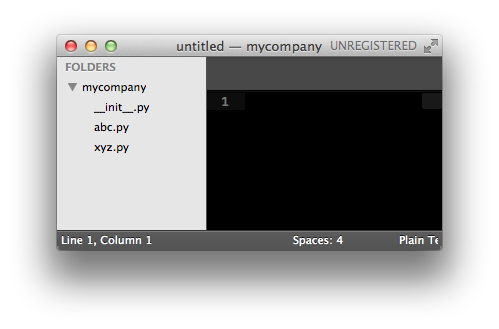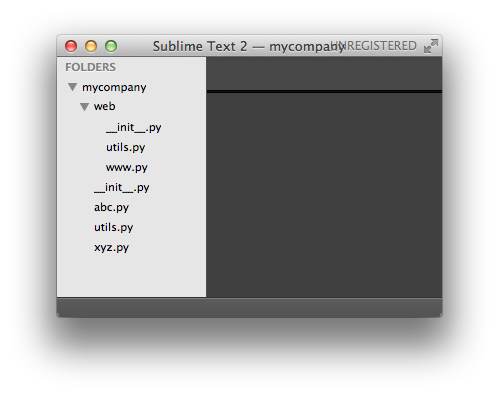`mycompany.web`也是一个模块，请指出该模块对应的.py文件。

## 使用模块

Python本身就内置了很多非常有用的模块，只要安装完毕，这些模块就可以立刻使用。

``````#!/usr/bin/env python3
# -*- coding: utf-8 -*-

' a test module '

__author__ = 'Michael Liao'

import sys

def test():
args = sys.argv
if len(args)==1:
print('Hello, world!')
elif len(args)==2:
print('Hello, %s!' % args)
else:
print('Too many arguments!')

if __name__=='__main__':
test()
``````

``````import sys
``````

`sys`模块有一个`argv`变量，用list存储了命令行的所有参数。`argv`至少有一个元素，因为第一个参数永远是该.py文件的名称，例如：

``````if __name__=='__main__':
test()
``````

``````\$ python3 hello.py
Hello, world!
\$ python hello.py Michael
Hello, Michael!
``````

``````\$ python3
Python 3.4.3 (v3.4.3:9b73f1c3e601, Feb 23 2015, 02:52:03)
[GCC 4.2.1 (Apple Inc. build 5666) (dot 3)] on darwin
>>> import hello
>>>
``````

``````>>> hello.test()
Hello, world!
``````

#### 作用域

private函数或变量不应该被别人引用，那它们有什么用呢？请看例子：

``````def _private_1(name):
return 'Hello, %s' % name

def _private_2(name):
return 'Hi, %s' % name

def greeting(name):
if len(name) > 3:
return _private_1(name)
else:
return _private_2(name)
``````

## 安装第三方模块

``````pip install Pillow
``````

``````>>> from PIL import Image
>>> im = Image.open('test.png')
>>> print(im.format, im.size, im.mode)
PNG (400, 300) RGB
>>> im.thumbnail((200, 100))
>>> im.save('thumb.jpg', 'JPEG')
``````

#### 模块搜索路径

``````>>> import mymodule
Traceback (most recent call last):
File "<stdin>", line 1, in <module>
ImportError: No module named mymodule
``````

``````>>> import sys
>>> sys.path
['', '/Library/Frameworks/Python.framework/Versions/3.4/lib/python34.zip', '/Library/Frameworks/Python.framework/Versions/3.4/lib/python3.4', '/Library/Frameworks/Python.framework/Versions/3.4/lib/python3.4/plat-darwin', '/Library/Frameworks/Python.framework/Versions/3.4/lib/python3.4/lib-dynload', '/Library/Frameworks/Python.framework/Versions/3.4/lib/python3.4/site-packages']
``````

``````>>> import sys
>>> sys.path.append('/Users/michael/my_py_scripts')
``````

# 面向对象编程

``````std1 = { 'name': 'Michael', 'score': 98 }
std2 = { 'name': 'Bob', 'score': 81 }
``````

``````def print_score(std):
print('%s: %s' % (std['name'], std['score']))
``````

``````class Student(object):

def __init__(self, name, score):
self.name = name
self.score = score

def print_score(self):
print('%s: %s' % (self.name, self.score))
``````

``````bart = Student('Bart Simpson', 59)
lisa = Student('Lisa Simpson', 87)
bart.print_score()
lisa.print_score()
``````

## 类和实例

``````class Student(object):
pass
``````

`class`后面紧接着是类名，即`Student`，类名通常是大写开头的单词，紧接着是`(object)`，表示该类是从哪个类继承下来的，继承的概念我们后面再讲，通常，如果没有合适的继承类，就使用`object`类，这是所有类最终都会继承的类。

``````>>> bart = Student()
>>> bart
<__main__.Student object at 0x10a67a590>
>>> Student
<class '__main__.Student'>
``````

``````>>> bart.name = 'Bart Simpson'
>>> bart.name
'Bart Simpson'
``````

``````class Student(object):

def __init__(self, name, score):
self.name = name
self.score = score
``````

``````>>> bart = Student('Bart Simpson', 59)
>>> bart.name
'Bart Simpson'
>>> bart.score
59
``````

#### 数据封装

``````>>> def print_score(std):
...     print('%s: %s' % (std.name, std.score))
...
>>> print_score(bart)
Bart Simpson: 59
``````

``````class Student(object):

def __init__(self, name, score):
self.name = name
self.score = score

def print_score(self):
print('%s: %s' % (self.name, self.score))
``````

``````>>> bart.print_score()
Bart Simpson: 59
``````

``````class Student(object):
...

if self.score >= 90:
return 'A'
elif self.score >= 60:
return 'B'
else:
return 'C'
``````

``````>>> bart.get_grade()
'C'
``````

#### 小结

``````>>> bart = Student('Bart Simpson', 59)
>>> lisa = Student('Lisa Simpson', 87)
>>> bart.age = 8
>>> bart.age
8
>>> lisa.age
Traceback (most recent call last):
File "<stdin>", line 1, in <module>
AttributeError: 'Student' object has no attribute 'age'
``````

student.py

## 访问限制

``````>>> bart = Student('Bart Simpson', 98)
>>> bart.score
98
>>> bart.score = 59
>>> bart.score
59
``````

``````class Student(object):

def __init__(self, name, score):
self.__name = name
self.__score = score

def print_score(self):
print('%s: %s' % (self.__name, self.__score))
``````

``````>>> bart = Student('Bart Simpson', 98)
>>> bart.__name
Traceback (most recent call last):
File "<stdin>", line 1, in <module>
AttributeError: 'Student' object has no attribute '__name'
``````

``````class Student(object):
...

def get_name(self):
return self.__name

def get_score(self):
return self.__score
``````

``````class Student(object):
...

def set_score(self, score):
self.__score = score
``````

``````class Student(object):
...

def set_score(self, score):
if 0 <= score <= 100:
self.__score = score
else:
``````

``````>>> bart._Student__name
'Bart Simpson'
``````

#### 参考源码

protected_student.py

## 继承和多态

``````class Animal(object):
def run(self):
print('Animal is running...')
``````

``````class Dog(Animal):
pass

class Cat(Animal):
pass
``````

``````dog = Dog()
dog.run()

cat = Cat()
cat.run()
``````

``````Animal is running...
Animal is running...
``````

``````class Dog(Animal):

def run(self):
print('Dog is running...')

def eat(self):
print('Eating meat...')
``````

``````class Dog(Animal):

def run(self):
print('Dog is running...')

class Cat(Animal):

def run(self):
print('Cat is running...')
``````

``````Dog is running...
Cat is running...
``````

``````a = list() # a是list类型
b = Animal() # b是Animal类型
c = Dog() # c是Dog类型
``````

``````>>> isinstance(a, list)
True
>>> isinstance(b, Animal)
True
>>> isinstance(c, Dog)
True
``````

``````>>> isinstance(c, Animal)
True
``````

``````>>> b = Animal()
>>> isinstance(b, Dog)
False
``````

`Dog`可以看成`Animal`，但`Animal`不可以看成`Dog`

``````def run_twice(animal):
animal.run()
animal.run()
``````

``````>>> run_twice(Animal())
Animal is running...
Animal is running...
``````

``````>>> run_twice(Dog())
Dog is running...
Dog is running...
``````

``````>>> run_twice(Cat())
Cat is running...
Cat is running...
``````

``````class Tortoise(Animal):
def run(self):
print('Tortoise is running slowly...')
``````

``````>>> run_twice(Tortoise())
Tortoise is running slowly...
Tortoise is running slowly...
``````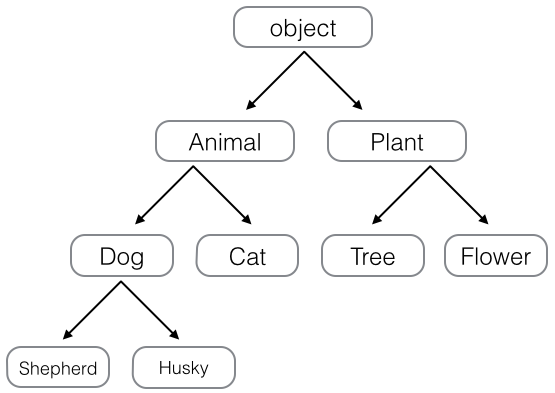#### 静态语言 vs 动态语言

``````class Timer(object):
def run(self):
print('Start...')
``````

Python的“file-like object“就是一种鸭子类型。对真正的文件对象，它有一个`read()`方法，返回其内容。但是，许多对象，只要有`read()`方法，都被视为“file-like object“。许多函数接收的参数就是“file-like object“，你不一定要传入真正的文件对象，完全可以传入任何实现了`read()`方法的对象。

animals.py

## 获取对象信息

#### 使用type()

``````>>> type(123)
<class 'int'>
>>> type('str')
<class 'str'>
>>> type(None)
<type(None) 'NoneType'>
``````

``````>>> type(abs)
<class 'builtin_function_or_method'>
>>> type(a)
<class '__main__.Animal'>
``````

``````>>> type(123)==type(456)
True
>>> type(123)==int
True
>>> type('abc')==type('123')
True
>>> type('abc')==str
True
>>> type('abc')==type(123)
False
``````

``````>>> import types
>>> def fn():
...     pass
...
>>> type(fn)==types.FunctionType
True
>>> type(abs)==types.BuiltinFunctionType
True
>>> type(lambda x: x)==types.LambdaType
True
>>> type((x for x in range(10)))==types.GeneratorType
True
``````

#### 使用isinstance()

``````object -> Animal -> Dog -> Husky
``````

``````>>> a = Animal()
>>> d = Dog()
>>> h = Husky()
``````

``````>>> isinstance(h, Husky)
True
``````

``````>>> isinstance(h, Dog)
True
``````

`h`虽然自身是Husky类型，但由于Husky是从Dog继承下来的，所以，`h`也还是Dog类型。换句话说，`isinstance()`判断的是一个对象是否是该类型本身，或者位于该类型的父继承链上。

``````>>> isinstance(h, Animal)
True
``````

``````>>> isinstance(d, Dog) and isinstance(d, Animal)
True
``````

``````>>> isinstance(d, Husky)
False
``````

``````>>> isinstance('a', str)
True
>>> isinstance(123, int)
True
>>> isinstance(b'a', bytes)
True
``````

``````>>> isinstance([1, 2, 3], (list, tuple))
True
>>> isinstance((1, 2, 3), (list, tuple))
True
``````

#### 使用dir()

``````>>> dir('ABC')
['__add__', '__class__', '__contains__', '__delattr__', '__dir__', '__doc__', '__eq__', '__format__', '__ge__', '__getattribute__', '__getitem__', '__getnewargs__', '__gt__', '__hash__', '__init__', '__iter__', '__le__', '__len__', '__lt__', '__mod__', '__mul__', '__ne__', '__new__', '__reduce__', '__reduce_ex__', '__repr__', '__rmod__', '__rmul__', '__setattr__', '__sizeof__', '__str__', '__subclasshook__', 'capitalize', 'casefold', 'center', 'count', 'encode', 'endswith', 'expandtabs', 'find', 'format', 'format_map', 'index', 'isalnum', 'isalpha', 'isdecimal', 'isdigit', 'isidentifier', 'islower', 'isnumeric', 'isprintable', 'isspace', 'istitle', 'isupper', 'join', 'ljust', 'lower', 'lstrip', 'maketrans', 'partition', 'replace', 'rfind', 'rindex', 'rjust', 'rpartition', 'rsplit', 'rstrip', 'split', 'splitlines', 'startswith', 'strip', 'swapcase', 'title', 'translate', 'upper', 'zfill']
``````

``````>>> len('ABC')
3
>>> 'ABC'.__len__()
3
``````

``````>>> class MyDog(object):
...     def __len__(self):
...         return 100
...
>>> dog = MyDog()
>>> len(dog)
100
``````

``````>>> 'ABC'.lower()
'abc'
``````

``````>>> class MyObject(object):
...     def __init__(self):
...         self.x = 9
...     def power(self):
...         return self.x * self.x
...
>>> obj = MyObject()
``````

``````>>> hasattr(obj, 'x') # 有属性'x'吗？
True
>>> obj.x
9
>>> hasattr(obj, 'y') # 有属性'y'吗？
False
>>> setattr(obj, 'y', 19) # 设置一个属性'y'
>>> hasattr(obj, 'y') # 有属性'y'吗？
True
>>> getattr(obj, 'y') # 获取属性'y'
19
>>> obj.y # 获取属性'y'
19
``````

``````>>> getattr(obj, 'z') # 获取属性'z'
Traceback (most recent call last):
File "<stdin>", line 1, in <module>
AttributeError: 'MyObject' object has no attribute 'z'
``````

``````>>> getattr(obj, 'z', 404) # 获取属性'z'，如果不存在，返回默认值404
404
``````

``````>>> hasattr(obj, 'power') # 有属性'power'吗？
True
>>> getattr(obj, 'power') # 获取属性'power'
<bound method MyObject.power of <__main__.MyObject object at 0x10077a6a0>>
>>> fn = getattr(obj, 'power') # 获取属性'power'并赋值到变量fn
>>> fn # fn指向obj.power
<bound method MyObject.power of <__main__.MyObject object at 0x10077a6a0>>
>>> fn() # 调用fn()与调用obj.power()是一样的
81
``````

#### 小结

``````sum = obj.x + obj.y
``````

``````sum = getattr(obj, 'x') + getattr(obj, 'y')
``````

``````def readImage(fp):
return None
``````

get_type.py

attrs.py

## 实例属性和类属性

``````class Student(object):
def __init__(self, name):
self.name = name

s = Student('Bob')
s.score = 90
``````

``````class Student(object):
name = 'Student'
``````

``````>>> class Student(object):
...     name = 'Student'
...
>>> s = Student() # 创建实例s
>>> print(s.name) # 打印name属性，因为实例并没有name属性，所以会继续查找class的name属性
Student
>>> print(Student.name) # 打印类的name属性
Student
>>> s.name = 'Michael' # 给实例绑定name属性
>>> print(s.name) # 由于实例属性优先级比类属性高，因此，它会屏蔽掉类的name属性
Michael
>>> print(Student.name) # 但是类属性并未消失，用Student.name仍然可以访问
Student
>>> del s.name # 如果删除实例的name属性
>>> print(s.name) # 再次调用s.name，由于实例的name属性没有找到，类的name属性就显示出来了
Student
``````

# 面向对象高级编程

## 使用__slots__

``````class Student(object):
pass
``````

``````>>> s = Student()
>>> s.name = 'Michael' # 动态给实例绑定一个属性
>>> print(s.name)
Michael
``````

``````>>> def set_age(self, age): # 定义一个函数作为实例方法
...     self.age = age
...
>>> from types import MethodType
>>> s.set_age = MethodType(set_age, s) # 给实例绑定一个方法
>>> s.set_age(25) # 调用实例方法
>>> s.age # 测试结果
25
``````

``````>>> s2 = Student() # 创建新的实例
>>> s2.set_age(25) # 尝试调用方法
Traceback (most recent call last):
File "<stdin>", line 1, in <module>
AttributeError: 'Student' object has no attribute 'set_age'
``````

``````>>> def set_score(self, score):
...     self.score = score
...
>>> Student.set_score = MethodType(set_score, Student)
``````

``````>>> s.set_score(100)
>>> s.score
100
>>> s2.set_score(99)
>>> s2.score
99
``````

#### 使用__slots__

``````class Student(object):
__slots__ = ('name', 'age') # 用tuple定义允许绑定的属性名称
``````

``````>>> s = Student() # 创建新的实例
>>> s.name = 'Michael' # 绑定属性'name'
>>> s.age = 25 # 绑定属性'age'
>>> s.score = 99 # 绑定属性'score'
Traceback (most recent call last):
File "<stdin>", line 1, in <module>
AttributeError: 'Student' object has no attribute 'score'
``````

``````>>> class GraduateStudent(Student):
...     pass
...
>>> g.score = 9999
``````

use_slots.py

## 使用@property

``````s = Student()
s.score = 9999
``````

``````class Student(object):

def get_score(self):
return self._score

def set_score(self, value):
if not isinstance(value, int):
raise ValueError('score must be an integer!')
if value < 0 or value > 100:
raise ValueError('score must between 0 ~ 100!')
self._score = value
``````

``````>>> s = Student()
>>> s.set_score(60) # ok!
>>> s.get_score()
60
>>> s.set_score(9999)
Traceback (most recent call last):
...
ValueError: score must between 0 ~ 100!
``````

``````class Student(object):

@property
def score(self):
return self._score

@score.setter
def score(self, value):
if not isinstance(value, int):
raise ValueError('score must be an integer!')
if value < 0 or value > 100:
raise ValueError('score must between 0 ~ 100!')
self._score = value
``````

`@property`的实现比较复杂，我们先考察如何使用。把一个getter方法变成属性，只需要加上`@property`就可以了，此时，`@property`本身又创建了另一个装饰器`@score.setter`，负责把一个setter方法变成属性赋值，于是，我们就拥有一个可控的属性操作：

``````>>> s = Student()
>>> s.score = 60 # OK，实际转化为s.set_score(60)
>>> s.score # OK，实际转化为s.get_score()
60
>>> s.score = 9999
Traceback (most recent call last):
...
ValueError: score must between 0 ~ 100!
``````

``````class Student(object):

@property
def birth(self):
return self._birth

@birth.setter
def birth(self, value):
self._birth = value

@property
def age(self):
return 2015 - self._birth
``````

#### 小结

`@property`广泛应用在类的定义中，可以让调用者写出简短的代码，同时保证对参数进行必要的检查，这样，程序运行时就减少了出错的可能性。

#### 练习

```# -*- coding: utf-8 -*-

class Screen(object):
----
pass
----
# test:
s = Screen()
s.width = 1024
s.height = 768
print(s.resolution)
assert s.resolution == 786432, '1024 * 768 = %d ?' % s.resolution
```

use_property.py

## 多重继承

• Dog - 狗狗；
• Bat - 蝙蝠；
• Parrot - 鹦鹉；
• Ostrich - 鸵鸟。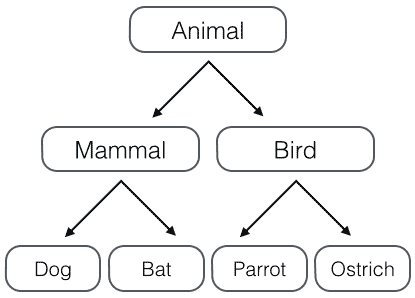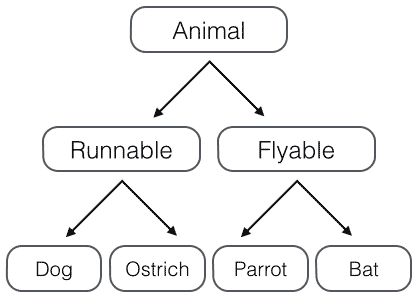• 哺乳类：能跑的哺乳类，能飞的哺乳类；
• 鸟类：能跑的鸟类，能飞的鸟类。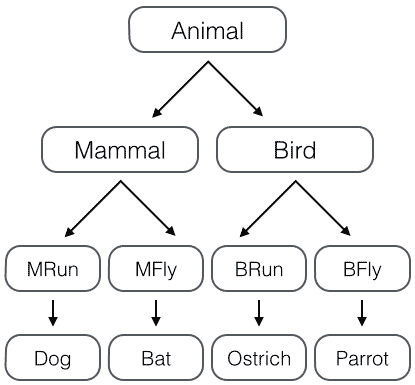``````class Animal(object):
pass

# 大类:
class Mammal(Animal):
pass

class Bird(Animal):
pass

# 各种动物:
class Dog(Mammal):
pass

class Bat(Mammal):
pass

class Parrot(Bird):
pass

class Ostrich(Bird):
pass
``````

``````class Runnable(object):
def run(self):
print('Running...')

class Flyable(object):
def fly(self):
print('Flying...')
``````

``````class Dog(Mammal, Runnable):
pass
``````

``````class Bat(Mammal, Flyable):
pass
``````

#### MixIn

``````class Dog(Mammal, RunnableMixIn, CarnivorousMixIn):
pass
``````

MixIn的目的就是给一个类增加多个功能，这样，在设计类的时候，我们优先考虑通过多重继承来组合多个MixIn的功能，而不是设计多层次的复杂的继承关系。

Python自带的很多库也使用了MixIn。举个例子，Python自带了`TCPServer``UDPServer`这两类网络服务，而要同时服务多个用户就必须使用多进程或多线程模型，这两种模型由`ForkingMixIn``ThreadingMixIn`提供。通过组合，我们就可以创造出合适的服务来。

``````class MyTCPServer(TCPServer, ForkingMixIn):
pass
``````

``````class MyUDPServer(UDPServer, ThreadingMixIn):
pass
``````

``````class MyTCPServer(TCPServer, CoroutineMixIn):
pass
``````

## 定制类

`__slots__`我们已经知道怎么用了，`__len__()`方法我们也知道是为了能让class作用于`len()`函数。

#### __str__

``````>>> class Student(object):
...     def __init__(self, name):
...         self.name = name
...
>>> print(Student('Michael'))
<__main__.Student object at 0x109afb190>
``````

``````>>> class Student(object):
...     def __init__(self, name):
...         self.name = name
...     def __str__(self):
...         return 'Student object (name: %s)' % self.name
...
>>> print(Student('Michael'))
Student object (name: Michael)
``````

``````>>> s = Student('Michael')
>>> s
<__main__.Student object at 0x109afb310>
``````

``````class Student(object):
def __init__(self, name):
self.name = name
def __str__(self):
return 'Student object (name=%s)' % self.name
__repr__ = __str__
``````

#### __iter__

``````class Fib(object):
def __init__(self):
self.a, self.b = 0, 1 # 初始化两个计数器a，b

def __iter__(self):
return self # 实例本身就是迭代对象，故返回自己

def __next__(self):
self.a, self.b = self.b, self.a + self.b # 计算下一个值
if self.a > 100000: # 退出循环的条件
raise StopIteration();
return self.a # 返回下一个值
``````

``````>>> for n in Fib():
...     print(n)
...
1
1
2
3
5
...
46368
75025
``````

#### __getitem__

Fib实例虽然能作用于for循环，看起来和list有点像，但是，把它当成list来使用还是不行，比如，取第5个元素：

``````>>> Fib()
Traceback (most recent call last):
File "<stdin>", line 1, in <module>
TypeError: 'Fib' object does not support indexing
``````

``````class Fib(object):
def __getitem__(self, n):
a, b = 1, 1
for x in range(n):
a, b = b, a + b
return a
``````

``````>>> f = Fib()
>>> f
1
>>> f
1
>>> f
2
>>> f
3
>>> f
89
>>> f
573147844013817084101
``````

``````>>> list(range(100))[5:10]
[5, 6, 7, 8, 9]
``````

``````class Fib(object):
def __getitem__(self, n):
if isinstance(n, int): # n是索引
a, b = 1, 1
for x in range(n):
a, b = b, a + b
return a
if isinstance(n, slice): # n是切片
start = n.start
stop = n.stop
if start is None:
start = 0
a, b = 1, 1
L = []
for x in range(stop):
if x >= start:
L.append(a)
a, b = b, a + b
return L
``````

``````>>> f = Fib()
>>> f[0:5]
[1, 1, 2, 3, 5]
>>> f[:10]
[1, 1, 2, 3, 5, 8, 13, 21, 34, 55]
``````

``````>>> f[:10:2]
[1, 1, 2, 3, 5, 8, 13, 21, 34, 55, 89]
``````

#### __getattr__

``````class Student(object):

def __init__(self):
self.name = 'Michael'
``````

``````>>> s = Student()
>>> print(s.name)
Michael
>>> print(s.score)
Traceback (most recent call last):
...
AttributeError: 'Student' object has no attribute 'score'
``````

``````class Student(object):

def __init__(self):
self.name = 'Michael'

def __getattr__(self, attr):
if attr=='score':
return 99
``````

``````>>> s = Student()
>>> s.name
'Michael'
>>> s.score
99
``````

``````class Student(object):

def __getattr__(self, attr):
if attr=='age':
return lambda: 25
``````

``````>>> s.age()
25
``````

``````class Student(object):

def __getattr__(self, attr):
if attr=='age':
return lambda: 25
raise AttributeError('\'Student\' object has no attribute \'%s\'' % attr)
``````

``````class Chain(object):

def __init__(self, path=''):
self._path = path

def __getattr__(self, path):
return Chain('%s/%s' % (self._path, path))

def __str__(self):
return self._path

__repr__ = __str__
``````

``````>>> Chain().status.user.timeline.list
'/status/user/timeline/list'
``````

``````GET /users/:user/repos
``````

``````Chain().users('michael').repos
``````

#### __call__

``````class Student(object):
def __init__(self, name):
self.name = name

def __call__(self):
print('My name is %s.' % self.name)
``````

``````>>> s = Student('Michael')
>>> s() # self参数不要传入
My name is Michael.
``````

`__call__()`还可以定义参数。对实例进行直接调用就好比对一个函数进行调用一样，所以你完全可以把对象看成函数，把函数看成对象，因为这两者之间本来就没啥根本的区别。

``````>>> callable(Student())
True
>>> callable(max)
True
>>> callable([1, 2, 3])
False
>>> callable(None)
False
>>> callable('str')
False
``````

#### 小结

Python的class允许定义许多定制方法，可以让我们非常方便地生成特定的类。

#### 参考源码

special_str.py

special_iter.py

special_getitem.py

special_getattr.py

special_call.py

## 使用枚举类

``````JAN = 1
FEB = 2
MAR = 3
...
NOV = 11
DEC = 12
``````

``````from enum import Enum

Month = Enum('Month', ('Jan', 'Feb', 'Mar', 'Apr', 'May', 'Jun', 'Jul', 'Aug', 'Sep', 'Oct', 'Nov', 'Dec'))
``````

``````for name, member in Month.__members__.items():
print(name, '=>', member, ',', member.value)
``````

`value`属性则是自动赋给成员的`int`常量，默认从`1`开始计数。

``````from enum import Enum, unique

@unique
class Weekday(Enum):
Sun = 0 # Sun的value被设定为0
Mon = 1
Tue = 2
Wed = 3
Thu = 4
Fri = 5
Sat = 6
``````

`@unique`装饰器可以帮助我们检查保证没有重复值。

``````>>> day1 = Weekday.Mon
>>> print(day1)
Weekday.Mon
>>> print(Weekday.Tue)
Weekday.Tue
>>> print(Weekday['Tue'])
Weekday.Tue
>>> print(Weekday.Tue.value)
2
>>> print(day1 == Weekday.Mon)
True
>>> print(day1 == Weekday.Tue)
False
>>> print(Weekday(1))
Weekday.Mon
>>> print(day1 == Weekday(1))
True
>>> Weekday(7)
Traceback (most recent call last):
...
ValueError: 7 is not a valid Weekday
>>> for name, member in Weekday.__members__.items():
...     print(name, '=>', member)
...
Sun => Weekday.Sun
Mon => Weekday.Mon
Tue => Weekday.Tue
Wed => Weekday.Wed
Thu => Weekday.Thu
Fri => Weekday.Fri
Sat => Weekday.Sat
``````

#### 小结

`Enum`可以把一组相关常量定义在一个class中，且class不可变，而且成员可以直接比较。

use_enum.py

## 使用元类

#### type()

``````class Hello(object):
def hello(self, name='world'):
print('Hello, %s.' % name)
``````

``````>>> from hello import Hello
>>> h = Hello()
>>> h.hello()
Hello, world.
>>> print(type(Hello))
<class 'type'>
>>> print(type(h))
<class 'hello.Hello'>
``````

`type()`函数可以查看一个类型或变量的类型，`Hello`是一个class，它的类型就是`type`，而`h`是一个实例，它的类型就是class `Hello`

`type()`函数既可以返回一个对象的类型，又可以创建出新的类型，比如，我们可以通过`type()`函数创建出`Hello`类，而无需通过`class Hello(object)...`的定义：

``````>>> def fn(self, name='world'): # 先定义函数
...     print('Hello, %s.' % name)
...
>>> Hello = type('Hello', (object,), dict(hello=fn)) # 创建Hello class
>>> h = Hello()
>>> h.hello()
Hello, world.
>>> print(type(Hello))
<class 'type'>
>>> print(type(h))
<class '__main__.Hello'>
``````

1. class的名称；
2. 继承的父类集合，注意Python支持多重继承，如果只有一个父类，别忘了tuple的单元素写法；
3. class的方法名称与函数绑定，这里我们把函数`fn`绑定到方法名`hello`上。

#### metaclass

metaclass，直译为元类，简单的解释就是：

metaclass是Python面向对象里最难理解，也是最难使用的魔术代码。正常情况下，你不会碰到需要使用metaclass的情况，所以，以下内容看不懂也没关系，因为基本上你不会用到。

``````# metaclass是类的模板，所以必须从`type`类型派生：
class ListMetaclass(type):
def __new__(cls, name, bases, attrs):
attrs['add'] = lambda self, value: self.append(value)
return type.__new__(cls, name, bases, attrs)
``````

``````class MyList(list, metaclass=ListMetaclass):
pass
``````

`__new__()`方法接收到的参数依次是：

1. 当前准备创建的类的对象；

2. 类的名字；

3. 类继承的父类集合；

4. 类的方法集合。

``````>>> L = MyList()
>> L

``````

``````>>> L2 = list()
Traceback (most recent call last):
File "<stdin>", line 1, in <module>
AttributeError: 'list' object has no attribute 'add'
``````

ORM全称“Object Relational Mapping”，即对象-关系映射，就是把关系数据库的一行映射为一个对象，也就是一个类对应一个表，这样，写代码更简单，不用直接操作SQL语句。

``````class User(Model):
# 定义类的属性到列的映射：
id = IntegerField('id')
email = StringField('email')

# 创建一个实例：
u = User(id=12345, name='Michael', email='test@orm.org', password='my-pwd')
# 保存到数据库：
u.save()
``````

``````class Field(object):

def __init__(self, name, column_type):
self.name = name
self.column_type = column_type

def __str__(self):
return '<%s:%s>' % (self.__class__.__name__, self.name)
``````

`Field`的基础上，进一步定义各种类型的`Field`，比如`StringField``IntegerField`等等：

``````class StringField(Field):

def __init__(self, name):
super(StringField, self).__init__(name, 'varchar(100)')

class IntegerField(Field):

def __init__(self, name):
super(IntegerField, self).__init__(name, 'bigint')
``````

``````class ModelMetaclass(type):

def __new__(cls, name, bases, attrs):
if name=='Model':
return type.__new__(cls, name, bases, attrs)
print('Found model: %s' % name)
mappings = dict()
for k, v in attrs.items():
if isinstance(v, Field):
print('Found mapping: %s ==> %s' % (k, v))
mappings[k] = v
for k in mappings.keys():
attrs.pop(k)
attrs['__mappings__'] = mappings # 保存属性和列的映射关系
attrs['__table__'] = name # 假设表名和类名一致
return type.__new__(cls, name, bases, attrs)
``````

``````class Model(dict, metaclass=ModelMetaclass):

def __init__(self, **kw):
super(Model, self).__init__(**kw)

def __getattr__(self, key):
try:
return self[key]
except KeyError:
raise AttributeError(r"'Model' object has no attribute '%s'" % key)

def __setattr__(self, key, value):
self[key] = value

def save(self):
fields = []
params = []
args = []
for k, v in self.__mappings__.items():
fields.append(v.name)
params.append('?')
args.append(getattr(self, k, None))
sql = 'insert into %s (%s) values (%s)' % (self.__table__, ','.join(fields), ','.join(params))
print('SQL: %s' % sql)
print('ARGS: %s' % str(args))
``````

`ModelMetaclass`中，一共做了几件事情：

1. 排除掉对`Model`类的修改；

2. 在当前类（比如`User`）中查找定义的类的所有属性，如果找到一个Field属性，就把它保存到一个`__mappings__`的dict中，同时从类属性中删除该Field属性，否则，容易造成运行时错误（实例的属性会遮盖类的同名属性）；

3. 把表名保存到`__table__`中，这里简化为表名默认为类名。

`Model`类中，就可以定义各种操作数据库的方法，比如`save()``delete()``find()``update`等等。

``````u = User(id=12345, name='Michael', email='test@orm.org', password='my-pwd')
u.save()
``````

``````Found model: User
Found mapping: email ==> <StringField:email>
Found mapping: id ==> <IntegerField:uid>
ARGS: ['my-pwd', 'test@orm.org', 'Michael', 12345]
``````

#### 小结

metaclass是Python中非常具有魔术性的对象，它可以改变类创建时的行为。这种强大的功能使用起来务必小心。

#### 参考源码

create_class_on_the_fly.py

use_metaclass.py

orm.py

# 错误、调试和测试

Python内置了一套异常处理机制，来帮助我们进行错误处理。

## 错误处理

``````def foo():
r = some_function()
if r==(-1):
return (-1)
# do something
return r

def bar():
r = foo()
if r==(-1):
print('Error')
else:
pass
``````

#### try

``````try:
print('try...')
r = 10 / 0
print('result:', r)
except ZeroDivisionError as e:
print('except:', e)
finally:
print('finally...')
print('END')
``````

``````try...
except: division by zero
finally...
END
``````

``````try...
result: 5
finally...
END
``````

``````try:
print('try...')
r = 10 / int('a')
print('result:', r)
except ValueError as e:
print('ValueError:', e)
except ZeroDivisionError as e:
print('ZeroDivisionError:', e)
finally:
print('finally...')
print('END')
``````

`int()`函数可能会抛出`ValueError`，所以我们用一个`except`捕获`ValueError`，用另一个`except`捕获`ZeroDivisionError`

``````try:
print('try...')
r = 10 / int('2')
print('result:', r)
except ValueError as e:
print('ValueError:', e)
except ZeroDivisionError as e:
print('ZeroDivisionError:', e)
else:
print('no error!')
finally:
print('finally...')
print('END')
``````

Python的错误其实也是class，所有的错误类型都继承自`BaseException`，所以在使用`except`时需要注意的是，它不但捕获该类型的错误，还把其子类也“一网打尽”。比如：

``````try:
foo()
except ValueError as e:
print('ValueError')
except UnicodeError as e:
print('UnicodeError')
``````

Python所有的错误都是从`BaseException`类派生的，常见的错误类型和继承关系看这里：

https://docs.python.org/3/library/exceptions.html#exception-hierarchy

``````def foo(s):
return 10 / int(s)

def bar(s):
return foo(s) * 2

def main():
try:
bar('0')
except Exception as e:
print('Error:', e)
finally:
print('finally...')
``````

#### 调用堆栈

``````# err.py:
def foo(s):
return 10 / int(s)

def bar(s):
return foo(s) * 2

def main():
bar('0')

main()
``````

``````\$ python3 err.py
Traceback (most recent call last):
File "err.py", line 11, in <module>
main()
File "err.py", line 9, in main
bar('0')
File "err.py", line 6, in bar
return foo(s) * 2
File "err.py", line 3, in foo
return 10 / int(s)
ZeroDivisionError: division by zero
``````

``````Traceback (most recent call last):
``````

``````  File "err.py", line 11, in <module>
main()
``````

``````  File "err.py", line 9, in main
bar('0')
``````

``````  File "err.py", line 6, in bar
return foo(s) * 2
``````

``````  File "err.py", line 3, in foo
return 10 / int(s)
``````

``````ZeroDivisionError: integer division or modulo by zero
``````

#### 记录错误

Python内置的`logging`模块可以非常容易地记录错误信息：

``````# err_logging.py

import logging

def foo(s):
return 10 / int(s)

def bar(s):
return foo(s) * 2

def main():
try:
bar('0')
except Exception as e:
logging.exception(e)

main()
print('END')
``````

``````\$ python3 err_logging.py
ERROR:root:division by zero
Traceback (most recent call last):
File "err_logging.py", line 13, in main
bar('0')
File "err_logging.py", line 9, in bar
return foo(s) * 2
File "err_logging.py", line 6, in foo
return 10 / int(s)
ZeroDivisionError: division by zero
END
``````

#### 抛出错误

``````# err_raise.py
class FooError(ValueError):
pass

def foo(s):
n = int(s)
if n==0:
raise FooError('invalid value: %s' % s)
return 10 / n

foo('0')
``````

``````\$ python3 err_raise.py
Traceback (most recent call last):
File "err_throw.py", line 11, in <module>
foo('0')
File "err_throw.py", line 8, in foo
raise FooError('invalid value: %s' % s)
__main__.FooError: invalid value: 0
``````

``````# err_reraise.py

def foo(s):
n = int(s)
if n==0:
raise ValueError('invalid value: %s' % s)
return 10 / n

def bar():
try:
foo('0')
except ValueError as e:
print('ValueError!')
raise

bar()
``````

`bar()`函数中，我们明明已经捕获了错误，但是，打印一个`ValueError!`后，又把错误通过`raise`语句抛出去了，这不有病么？

`raise`语句如果不带参数，就会把当前错误原样抛出。此外，在`except``raise`一个Error，还可以把一种类型的错误转化成另一种类型：

``````try:
10 / 0
except ZeroDivisionError:
raise ValueError('input error!')
``````

#### 小结

Python内置的`try...except...finally`用来处理错误十分方便。出错时，会分析错误信息并定位错误发生的代码位置才是最关键的。

do_try.py

err.py

err_logging.py

err_raise.py

err_reraise.py

## 调试

``````def foo(s):
n = int(s)
print('>>> n = %d' % n)
return 10 / n

def main():
foo('0')

main()
``````

``````\$ python3 err.py
>>> n = 0
Traceback (most recent call last):
...
ZeroDivisionError: integer division or modulo by zero
``````

`print()`最大的坏处是将来还得删掉它，想想程序里到处都是`print()`，运行结果也会包含很多垃圾信息。所以，我们又有第二种方法。

#### 断言

``````def foo(s):
n = int(s)
assert n != 0, 'n is zero!'
return 10 / n

def main():
foo('0')
``````

`assert`的意思是，表达式`n != 0`应该是`True`，否则，根据程序运行的逻辑，后面的代码肯定会出错。

``````\$ python3 err.py
Traceback (most recent call last):
...
AssertionError: n is zero!
``````

``````\$ python3 -O err.py
Traceback (most recent call last):
...
ZeroDivisionError: division by zero
``````

#### logging

`print()`替换为`logging`是第3种方式，和`assert`比，`logging`不会抛出错误，而且可以输出到文件：

``````import logging

s = '0'
n = int(s)
logging.info('n = %d' % n)
print(10 / n)
``````

`logging.info()`就可以输出一段文本。运行，发现除了`ZeroDivisionError`，没有任何信息。怎么回事？

``````import logging
logging.basicConfig(level=logging.INFO)
``````

``````\$ python3 err.py
INFO:root:n = 0
Traceback (most recent call last):
File "err.py", line 8, in <module>
print(10 / n)
ZeroDivisionError: division by zero
``````

`logging`的另一个好处是通过简单的配置，一条语句可以同时输出到不同的地方，比如console和文件。

#### pdb

``````# err.py
s = '0'
n = int(s)
print(10 / n)
``````

``````\$ python3 -m pdb err.py
> /Users/michael/Github/learn-python3/samples/debug/err.py(2)<module>()
-> s = '0'
``````

``````(Pdb) l
1     # err.py
2  -> s = '0'
3     n = int(s)
4     print(10 / n)
``````

``````(Pdb) n
> /Users/michael/Github/learn-python3/samples/debug/err.py(3)<module>()
-> n = int(s)
(Pdb) n
> /Users/michael/Github/learn-python3/samples/debug/err.py(4)<module>()
-> print(10 / n)
``````

``````(Pdb) p s
'0'
(Pdb) p n
0
``````

``````(Pdb) q
``````

#### pdb.set_trace()

``````# err.py
import pdb

s = '0'
n = int(s)
pdb.set_trace() # 运行到这里会自动暂停
print(10 / n)
``````

``````\$ python3 err.py
> /Users/michael/Github/learn-python3/samples/debug/err.py(7)<module>()
-> print(10 / n)
(Pdb) p n
0
(Pdb) c
Traceback (most recent call last):
File "err.py", line 7, in <module>
print(10 / n)
ZeroDivisionError: division by zero
``````

#### IDE

http://www.jetbrains.com/pycharm/

do_assert.py

do_logging.py

do_pdb.py

## 单元测试

1. 输入正数，比如`1``1.2``0.99`，期待返回值与输入相同；

2. 输入负数，比如`-1``-1.2``-0.99`，期待返回值与输入相反；

3. 输入`0`，期待返回`0`

4. 输入非数值类型，比如`None``[]``{}`，期待抛出`TypeError`

``````>>> d = Dict(a=1, b=2)
>>> d['a']
1
>>> d.a
1
``````

`mydict.py`代码如下：

``````class Dict(dict):

def __init__(self, **kw):
super().__init__(**kw)

def __getattr__(self, key):
try:
return self[key]
except KeyError:
raise AttributeError(r"'Dict' object has no attribute '%s'" % key)

def __setattr__(self, key, value):
self[key] = value
``````

``````import unittest

from mydict import Dict

class TestDict(unittest.TestCase):

def test_init(self):
d = Dict(a=1, b='test')
self.assertEqual(d.a, 1)
self.assertEqual(d.b, 'test')
self.assertTrue(isinstance(d, dict))

def test_key(self):
d = Dict()
d['key'] = 'value'
self.assertEqual(d.key, 'value')

def test_attr(self):
d = Dict()
d.key = 'value'
self.assertTrue('key' in d)
self.assertEqual(d['key'], 'value')

def test_keyerror(self):
d = Dict()
with self.assertRaises(KeyError):
value = d['empty']

def test_attrerror(self):
d = Dict()
with self.assertRaises(AttributeError):
value = d.empty
``````

`test`开头的方法就是测试方法，不以`test`开头的方法不被认为是测试方法，测试的时候不会被执行。

``````self.assertEqual(abs(-1), 1) # 断言函数返回的结果与1相等
``````

``````with self.assertRaises(KeyError):
value = d['empty']
``````

``````with self.assertRaises(AttributeError):
value = d.empty
``````

#### 运行单元测试

``````if __name__ == '__main__':
unittest.main()
``````

``````\$ python3 mydict_test.py
``````

``````\$ python3 -m unittest mydict_test
.....
----------------------------------------------------------------------
Ran 5 tests in 0.000s

OK
``````

#### setUp与tearDown

`setUp()``tearDown()`方法有什么用呢？设想你的测试需要启动一个数据库，这时，就可以在`setUp()`方法中连接数据库，在`tearDown()`方法中关闭数据库，这样，不必在每个测试方法中重复相同的代码：

``````class TestDict(unittest.TestCase):

def setUp(self):
print('setUp...')

def tearDown(self):
print('tearDown...')
``````

mydict.py

mydict_test.py

## 文档测试

``````>>> import re
>>> m = re.search('(?<=abc)def', 'abcdef')
>>> m.group(0)
'def'
``````

``````def abs(n):
'''
Function to get absolute value of number.

Example:

>>> abs(1)
1
>>> abs(-1)
1
>>> abs(0)
0
'''
return n if n >= 0 else (-n)
``````

doctest严格按照Python交互式命令行的输入和输出来判断测试结果是否正确。只有测试异常的时候，可以用`...`表示中间一大段烦人的输出。

``````# mydict2.py
class Dict(dict):
'''
Simple dict but also support access as x.y style.

>>> d1 = Dict()
>>> d1['x'] = 100
>>> d1.x
100
>>> d1.y = 200
>>> d1['y']
200
>>> d2 = Dict(a=1, b=2, c='3')
>>> d2.c
'3'
>>> d2['empty']
Traceback (most recent call last):
...
KeyError: 'empty'
>>> d2.empty
Traceback (most recent call last):
...
AttributeError: 'Dict' object has no attribute 'empty'
'''
def __init__(self, **kw):
super(Dict, self).__init__(**kw)

def __getattr__(self, key):
try:
return self[key]
except KeyError:
raise AttributeError(r"'Dict' object has no attribute '%s'" % key)

def __setattr__(self, key, value):
self[key] = value

if __name__=='__main__':
import doctest
doctest.testmod()
``````

``````\$ python3 mydict2.py
``````

``````\$ python3 mydict2.py
**********************************************************************
File "/Users/michael/Github/learn-python3/samples/debug/mydict2.py", line 10, in __main__.Dict
Failed example:
d1.x
Exception raised:
Traceback (most recent call last):
...
AttributeError: 'Dict' object has no attribute 'x'
**********************************************************************
File "/Users/michael/Github/learn-python3/samples/debug/mydict2.py", line 16, in __main__.Dict
Failed example:
d2.c
Exception raised:
Traceback (most recent call last):
...
AttributeError: 'Dict' object has no attribute 'c'
**********************************************************************
2 of   9 in __main__.Dict
***Test Failed*** 2 failures.
``````

#### 练习

```# -*- coding: utf-8 -*-

def fact(n):
'''
----
----
'''
if n < 1:
raise ValueError()
if n == 1:
return 1
return n * fact(n - 1)

if __name__ == '__main__':
import doctest
doctest.testmod()
```

#### 小结

doctest非常有用，不但可以用来测试，还可以直接作为示例代码。通过某些文档生成工具，就可以自动把包含doctest的注释提取出来。用户看文档的时候，同时也看到了doctest。

mydict2.py

# IO编程

IO在计算机中指Input/Output，也就是输入和输出。由于程序和运行时数据是在内存中驻留，由CPU这个超快的计算核心来执行，涉及到数据交换的地方，通常是磁盘、网络等，就需要IO接口。

IO编程中，Stream（流）是一个很重要的概念，可以把流想象成一个水管，数据就是水管里的水，但是只能单向流动。Input Stream就是数据从外面（磁盘、网络）流进内存，Output Stream就是数据从内存流到外面去。对于浏览网页来说，浏览器和新浪服务器之间至少需要建立两根水管，才可以既能发数据，又能收数据。

## 文件读写

#### 读文件

``````>>> f = open('/Users/michael/test.txt', 'r')
``````

``````>>> f=open('/Users/michael/notfound.txt', 'r')
Traceback (most recent call last):
File "<stdin>", line 1, in <module>
FileNotFoundError: [Errno 2] No such file or directory: '/Users/michael/notfound.txt'
``````

``````>>> f.read()
'Hello, world!'
``````

``````>>> f.close()
``````

``````try:
f = open('/path/to/file', 'r')
finally:
if f:
f.close()
``````

``````with open('/path/to/file', 'r') as f:
``````

``````for line in f.readlines():
print(line.strip()) # 把末尾的'\n'删掉
``````

#### file-like Object

`open()`函数返回的这种有个`read()`方法的对象，在Python中统称为file-like Object。除了file外，还可以是内存的字节流，网络流，自定义流等等。file-like Object不要求从特定类继承，只要写个`read()`方法就行。

`StringIO`就是在内存中创建的file-like Object，常用作临时缓冲。

#### 二进制文件

``````>>> f = open('/Users/michael/test.jpg', 'rb')
b'\xff\xd8\xff\xe1\x00\x18Exif\x00\x00...' # 十六进制表示的字节
``````

#### 字符编码

``````>>> f = open('/Users/michael/gbk.txt', 'r', encoding='gbk')
'测试'
``````

``````>>> f = open('/Users/michael/gbk.txt', 'r', encoding='gbk', errors='ignore')
``````

#### 写文件

``````>>> f = open('/Users/michael/test.txt', 'w')
>>> f.write('Hello, world!')
>>> f.close()
``````

``````with open('/Users/michael/test.txt', 'w') as f:
f.write('Hello, world!')
``````

with_file.py

## StringIO和BytesIO

#### StringIO

StringIO顾名思义就是在内存中读写str。

``````>>> from io import StringIO
>>> f = StringIO()
>>> f.write('hello')
5
>>> f.write(' ')
1
>>> f.write('world!')
6
>>> print(f.getvalue())
hello world!
``````

`getvalue()`方法用于获得写入后的str。

``````>>> from io import StringIO
>>> f = StringIO('Hello!\nHi!\nGoodbye!')
>>> while True:
...     if s == '':
...         break
...     print(s.strip())
...
Hello!
Hi!
Goodbye!
``````

#### BytesIO

StringIO操作的只能是str，如果要操作二进制数据，就需要使用BytesIO。

BytesIO实现了在内存中读写bytes，我们创建一个BytesIO，然后写入一些bytes：

``````>>> from io import BytesIO
>>> f = BytesIO()
>>> f.write('中文'.encode('utf-8'))
6
>>> print(f.getvalue())
``````

``````>>> from io import StringIO
``````

#### 小结

StringIO和BytesIO是在内存中操作str和bytes的方法，使得和读写文件具有一致的接口。

do_stringio.py

do_bytesio.py

## 操作文件和目录

``````>>> import os
>>> os.name # 操作系统类型
'posix'
``````

``````>>> os.uname()
posix.uname_result(sysname='Darwin', nodename='MichaelMacPro.local', release='14.3.0', version='Darwin Kernel Version 14.3.0: Mon Mar 23 11:59:05 PDT 2015; root:xnu-2782.20.48~5/RELEASE_X86_64', machine='x86_64')
``````

#### 环境变量

``````>>> os.environ
environ({'VERSIONER_PYTHON_PREFER_32_BIT': 'no', 'TERM_PROGRAM_VERSION': '326', 'LOGNAME': 'michael', 'USER': 'michael', 'PATH': '/usr/bin:/bin:/usr/sbin:/sbin:/usr/local/bin:/opt/X11/bin:/usr/local/mysql/bin', ...})
``````

``````>>> os.environ.get('PATH')
'/usr/bin:/bin:/usr/sbin:/sbin:/usr/local/bin:/opt/X11/bin:/usr/local/mysql/bin'
>>> os.environ.get('x', 'default')
'default'
``````

#### 操作文件和目录

``````# 查看当前目录的绝对路径:
>>> os.path.abspath('.')
'/Users/michael'
# 在某个目录下创建一个新目录，首先把新目录的完整路径表示出来:
>>> os.path.join('/Users/michael', 'testdir')
'/Users/michael/testdir'
# 然后创建一个目录:
>>> os.mkdir('/Users/michael/testdir')
# 删掉一个目录:
>>> os.rmdir('/Users/michael/testdir')
``````

``````part-1/part-2
``````

``````part-1\part-2
``````

``````>>> os.path.split('/Users/michael/testdir/file.txt')
('/Users/michael/testdir', 'file.txt')
``````

`os.path.splitext()`可以直接让你得到文件扩展名，很多时候非常方便：

``````>>> os.path.splitext('/path/to/file.txt')
('/path/to/file', '.txt')
``````

``````# 对文件重命名:
>>> os.rename('test.txt', 'test.py')
# 删掉文件:
>>> os.remove('test.py')
``````

``````>>> [x for x in os.listdir('.') if os.path.isdir(x)]
['.lein', '.local', '.m2', '.npm', '.ssh', '.Trash', '.vim', 'Applications', 'Desktop', ...]
``````

``````>>> [x for x in os.listdir('.') if os.path.isfile(x) and os.path.splitext(x)=='.py']
['apis.py', 'config.py', 'models.py', 'pymonitor.py', 'test_db.py', 'urls.py', 'wsgiapp.py']
``````

#### 小结

Python的`os`模块封装了操作系统的目录和文件操作，要注意这些函数有的在`os`模块中，有的在`os.path`模块中。

#### 练习

1. 利用`os`模块编写一个能实现`dir -l`输出的程序。

2. 编写一个程序，能在当前目录以及当前目录的所有子目录下查找文件名包含指定字符串的文件，并打印出相对路径。

do_dir

## 序列化

``````d = dict(name='Bob', age=20, score=88)
``````

Python提供了`pickle`模块来实现序列化。

``````>>> import pickle
>>> d = dict(name='Bob', age=20, score=88)
>>> pickle.dumps(d)
b'\x80\x03}q\x00(X\x03\x00\x00\x00ageq\x01K\x14X\x05\x00\x00\x00scoreq\x02KXX\x04\x00\x00\x00nameq\x03X\x03\x00\x00\x00Bobq\x04u.'
``````

`pickle.dumps()`方法把任意对象序列化成一个`bytes`，然后，就可以把这个`bytes`写入文件。或者用另一个方法`pickle.dump()`直接把对象序列化后写入一个file-like Object：

``````>>> f = open('dump.txt', 'wb')
>>> pickle.dump(d, f)
>>> f.close()
``````

``````>>> f = open('dump.txt', 'rb')
>>> f.close()
>>> d
{'age': 20, 'score': 88, 'name': 'Bob'}
``````

Pickle的问题和所有其他编程语言特有的序列化问题一样，就是它只能用于Python，并且可能不同版本的Python彼此都不兼容，因此，只能用Pickle保存那些不重要的数据，不能成功地反序列化也没关系。

#### JSON

JSON表示的对象就是标准的JavaScript语言的对象，JSON和Python内置的数据类型对应如下：

 JSON类型 Python类型 {} dict [] list "string" str 1234.56 int或float true/false True/False null None

Python内置的`json`模块提供了非常完善的Python对象到JSON格式的转换。我们先看看如何把Python对象变成一个JSON：

``````>>> import json
>>> d = dict(name='Bob', age=20, score=88)
>>> json.dumps(d)
'{"age": 20, "score": 88, "name": "Bob"}'
``````

`dumps()`方法返回一个`str`，内容就是标准的JSON。类似的，`dump()`方法可以直接把JSON写入一个`file-like Object`

``````>>> json_str = '{"age": 20, "score": 88, "name": "Bob"}'
{'age': 20, 'score': 88, 'name': 'Bob'}
``````

#### JSON进阶

Python的`dict`对象可以直接序列化为JSON的`{}`，不过，很多时候，我们更喜欢用`class`表示对象，比如定义`Student`类，然后序列化：

``````import json

class Student(object):
def __init__(self, name, age, score):
self.name = name
self.age = age
self.score = score

s = Student('Bob', 20, 88)
print(json.dumps(s))
``````

``````Traceback (most recent call last):
...
TypeError: <__main__.Student object at 0x10603cc50> is not JSON serializable
``````

https://docs.python.org/3/library/json.html#json.dumps

``````def student2dict(std):
return {
'name': std.name,
'age': std.age,
'score': std.score
}
``````

``````>>> print(json.dumps(s, default=student2dict))
{"age": 20, "name": "Bob", "score": 88}
``````

``````print(json.dumps(s, default=lambda obj: obj.__dict__))
``````

``````def dict2student(d):
return Student(d['name'], d['age'], d['score'])
``````

``````>>> json_str = '{"age": 20, "score": 88, "name": "Bob"}'
<__main__.Student object at 0x10cd3c190>
``````

#### 小结

Python语言特定的序列化模块是`pickle`，但如果要把序列化搞得更通用、更符合Web标准，就可以使用`json`模块。

`json`模块的`dumps()``loads()`函数是定义得非常好的接口的典范。当我们使用时，只需要传入一个必须的参数。但是，当默认的序列化或反序列机制不满足我们的要求时，我们又可以传入更多的参数来定制序列化或反序列化的规则，既做到了接口简单易用，又做到了充分的扩展性和灵活性。

use_pickle.py

use_json.py

# 进程和线程

• 多进程模式；
• 多线程模式；
• 多进程+多线程模式。

Python既支持多进程，又支持多线程，我们会讨论如何编写这两种多任务程序。

## 多进程

Unix/Linux操作系统提供了一个`fork()`系统调用，它非常特殊。普通的函数调用，调用一次，返回一次，但是`fork()`调用一次，返回两次，因为操作系统自动把当前进程（称为父进程）复制了一份（称为子进程），然后，分别在父进程和子进程内返回。

Python的`os`模块封装了常见的系统调用，其中就包括`fork`，可以在Python程序中轻松创建子进程：

``````import os

print('Process (%s) start...' % os.getpid())
# Only works on Unix/Linux/Mac:
pid = os.fork()
if pid == 0:
print('I am child process (%s) and my parent is %s.' % (os.getpid(), os.getppid()))
else:
print('I (%s) just created a child process (%s).' % (os.getpid(), pid))
``````

``````Process (876) start...
I (876) just created a child process (877).
I am child process (877) and my parent is 876.
``````

#### multiprocessing

`multiprocessing`模块提供了一个`Process`类来代表一个进程对象，下面的例子演示了启动一个子进程并等待其结束：

``````from multiprocessing import Process
import os

# 子进程要执行的代码
def run_proc(name):
print('Run child process %s (%s)...' % (name, os.getpid()))

if __name__=='__main__':
print('Parent process %s.' % os.getpid())
p = Process(target=run_proc, args=('test',))
print('Child process will start.')
p.start()
p.join()
print('Child process end.')
``````

``````Parent process 928.
Process will start.
Run child process test (929)...
Process end.
``````

`join()`方法可以等待子进程结束后再继续往下运行，通常用于进程间的同步。

#### Pool

``````from multiprocessing import Pool
import os, time, random

print('Run task %s (%s)...' % (name, os.getpid()))
start = time.time()
time.sleep(random.random() * 3)
end = time.time()
print('Task %s runs %0.2f seconds.' % (name, (end - start)))

if __name__=='__main__':
print('Parent process %s.' % os.getpid())
p = Pool(4)
for i in range(5):
print('Waiting for all subprocesses done...')
p.close()
p.join()
print('All subprocesses done.')
``````

``````Parent process 669.
Waiting for all subprocesses done...
All subprocesses done.
``````

`Pool`对象调用`join()`方法会等待所有子进程执行完毕，调用`join()`之前必须先调用`close()`，调用`close()`之后就不能继续添加新的`Process`了。

``````p = Pool(5)
``````

#### 子进程

`subprocess`模块可以让我们非常方便地启动一个子进程，然后控制其输入和输出。

``````import subprocess

print('\$ nslookup www.python.org')
r = subprocess.call(['nslookup', 'www.python.org'])
print('Exit code:', r)
``````

``````\$ nslookup www.python.org
Server:        192.168.19.4

www.python.org    canonical name = python.map.fastly.net.
Name:    python.map.fastly.net

Exit code: 0
``````

``````import subprocess

print('\$ nslookup')
p = subprocess.Popen(['nslookup'], stdin=subprocess.PIPE, stdout=subprocess.PIPE, stderr=subprocess.PIPE)
output, err = p.communicate(b'set q=mx\npython.org\nexit\n')
print(output.decode('utf-8'))
print('Exit code:', p.returncode)
``````

``````set q=mx
python.org
exit
``````

``````\$ nslookup
Server:        192.168.19.4

python.org    mail exchanger = 50 mail.python.org.

Authoritative answers can be found from:

Exit code: 0
``````

#### 进程间通信

`Process`之间肯定是需要通信的，操作系统提供了很多机制来实现进程间的通信。Python的`multiprocessing`模块包装了底层的机制，提供了`Queue``Pipes`等多种方式来交换数据。

``````from multiprocessing import Process, Queue
import os, time, random

# 写数据进程执行的代码:
def write(q):
print('Process to write: %s' % os.getpid())
for value in ['A', 'B', 'C']:
print('Put %s to queue...' % value)
q.put(value)
time.sleep(random.random())

# 读数据进程执行的代码:
print('Process to read: %s' % os.getpid())
while True:
value = q.get(True)
print('Get %s from queue.' % value)

if __name__=='__main__':
# 父进程创建Queue，并传给各个子进程：
q = Queue()
pw = Process(target=write, args=(q,))
# 启动子进程pw，写入:
pw.start()
# 启动子进程pr，读取:
pr.start()
# 等待pw结束:
pw.join()
# pr进程里是死循环，无法等待其结束，只能强行终止:
pr.terminate()
``````

``````Process to write: 50563
Put A to queue...
Get A from queue.
Put B to queue...
Get B from queue.
Put C to queue...
Get C from queue.
``````

#### 参考源码

do_folk.py

multi_processing.py

pooled_processing.py

do_subprocess.py

do_queue.py

## 多线程

Python的标准库提供了两个模块：`_thread``threading``_thread`是低级模块，`threading`是高级模块，对`_thread`进行了封装。绝大多数情况下，我们只需要使用`threading`这个高级模块。

``````import time, threading

# 新线程执行的代码:
def loop():
n = 0
while n < 5:
n = n + 1
time.sleep(1)

t.start()
t.join()
``````

``````thread MainThread is running...
``````

#### Lock

``````import time, threading

# 假定这是你的银行存款:
balance = 0

def change_it(n):
# 先存后取，结果应该为0:
global balance
balance = balance + n
balance = balance - n

for i in range(100000):
change_it(n)

t1.start()
t2.start()
t1.join()
t2.join()
print(balance)
``````

``````balance = balance + n
``````

1. 计算`balance + n`，存入临时变量中；
2. 将临时变量的值赋给`balance`

``````x = balance + n
balance = x
``````

``````初始值 balance = 0

t1: x1 = balance + 5 # x1 = 0 + 5 = 5
t1: balance = x1     # balance = 5
t1: x1 = balance - 5 # x1 = 5 - 5 = 0
t1: balance = x1     # balance = 0

t2: x2 = balance + 8 # x2 = 0 + 8 = 8
t2: balance = x2     # balance = 8
t2: x2 = balance - 8 # x2 = 8 - 8 = 0
t2: balance = x2     # balance = 0

``````

``````初始值 balance = 0

t1: x1 = balance + 5  # x1 = 0 + 5 = 5

t2: x2 = balance + 8  # x2 = 0 + 8 = 8
t2: balance = x2      # balance = 8

t1: balance = x1      # balance = 5
t1: x1 = balance - 5  # x1 = 5 - 5 = 0
t1: balance = x1      # balance = 0

t2: x2 = balance - 8  # x2 = 0 - 8 = -8
t2: balance = x2   # balance = -8

``````

``````balance = 0

for i in range(100000):
# 先要获取锁:
lock.acquire()
try:
# 放心地改吧:
change_it(n)
finally:
# 改完了一定要释放锁:
lock.release()
``````

#### 多核CPU

``````import threading, multiprocessing

def loop():
x = 0
while True:
x = x ^ 1

for i in range(multiprocessing.cpu_count()):
t.start()
``````

GIL是Python解释器设计的历史遗留问题，通常我们用的解释器是官方实现的CPython，要真正利用多核，除非重写一个不带GIL的解释器。

#### 小结

Python解释器由于设计时有GIL全局锁，导致了多线程无法利用多核。多线程的并发在Python中就是一个美丽的梦。

#### 参考源码

do_lock.py

``````def process_student(name):
std = Student(name)
# std是局部变量，但是每个函数都要用它，因此必须传进去：

``````

``````global_dict = {}

std = Student(name)
# 把std放到全局变量global_dict中：

# 不传入std，而是根据当前线程查找：
...

# 任何函数都可以查找出当前线程的std变量：
...
``````

`ThreadLocal`应运而生，不用查找`dict``ThreadLocal`帮你自动做这件事：

``````import threading

def process_student():
# 获取当前线程关联的student:
std = local_school.student

local_school.student = name
process_student()

t1.start()
t2.start()
t1.join()
t2.join()
``````

``````Hello, Alice (in Thread-A)
``````

`ThreadLocal`最常用的地方就是为每个线程绑定一个数据库连接，HTTP请求，用户身份信息等，这样一个线程的所有调用到的处理函数都可以非常方便地访问这些资源。

## 进程 vs. 线程

#### 计算密集型 vs. IO密集型

IO密集型任务执行期间，99%的时间都花在IO上，花在CPU上的时间很少，因此，用运行速度极快的C语言替换用Python这样运行速度极低的脚本语言，完全无法提升运行效率。对于IO密集型任务，最合适的语言就是开发效率最高（代码量最少）的语言，脚本语言是首选，C语言最差。

## 分布式进程

Python的`multiprocessing`模块不但支持多进程，其中`managers`子模块还支持把多进程分布到多台机器上。一个服务进程可以作为调度者，将任务分布到其他多个进程中，依靠网络通信。由于`managers`模块封装很好，不必了解网络通信的细节，就可以很容易地编写分布式多进程程序。

``````# task_master.py

import random, time, queue
from multiprocessing.managers import BaseManager

# 发送任务的队列:
# 接收结果的队列:
result_queue = queue.Queue()

# 从BaseManager继承的QueueManager:
class QueueManager(BaseManager):
pass

# 把两个Queue都注册到网络上, callable参数关联了Queue对象:
QueueManager.register('get_result_queue', callable=lambda: result_queue)
# 绑定端口5000, 设置验证码'abc':
# 启动Queue:
manager.start()
# 获得通过网络访问的Queue对象:
result = manager.get_result_queue()
# 放几个任务进去:
for i in range(10):
n = random.randint(0, 10000)
# 从result队列读取结果:
print('Try get results...')
for i in range(10):
r = result.get(timeout=10)
print('Result: %s' % r)
# 关闭:
manager.shutdown()
print('master exit.')
``````

``````# task_worker.py

import time, sys, queue
from multiprocessing.managers import BaseManager

# 创建类似的QueueManager:
class QueueManager(BaseManager):
pass

# 由于这个QueueManager只从网络上获取Queue，所以注册时只提供名字:
QueueManager.register('get_result_queue')

print('Connect to server %s...' % server_addr)
# 从网络连接:
m.connect()
# 获取Queue的对象:
result = m.get_result_queue()
for i in range(10):
try:
print('run task %d * %d...' % (n, n))
r = '%d * %d = %d' % (n, n, n*n)
time.sleep(1)
result.put(r)
except Queue.Empty:
# 处理结束:
print('worker exit.')
``````

``````\$ python3 task_master.py
Try get results...
``````

`task_master.py`进程发送完任务后，开始等待`result`队列的结果。现在启动`task_worker.py`进程：

``````\$ python3 task_worker.py
Connect to server 127.0.0.1...
worker exit.
``````

`task_worker.py`进程结束，在`task_master.py`进程中会继续打印出结果：

``````Result: 3411 * 3411 = 11634921
Result: 1605 * 1605 = 2576025
Result: 1398 * 1398 = 1954404
Result: 4729 * 4729 = 22363441
Result: 5300 * 5300 = 28090000
Result: 7471 * 7471 = 55815841
Result: 68 * 68 = 4624
Result: 4219 * 4219 = 17799961
Result: 339 * 339 = 114921
Result: 7866 * 7866 = 61873956
``````

Queue对象存储在哪？注意到`task_worker.py`中根本没有创建Queue的代码，所以，Queue对象存储在`task_master.py`进程中：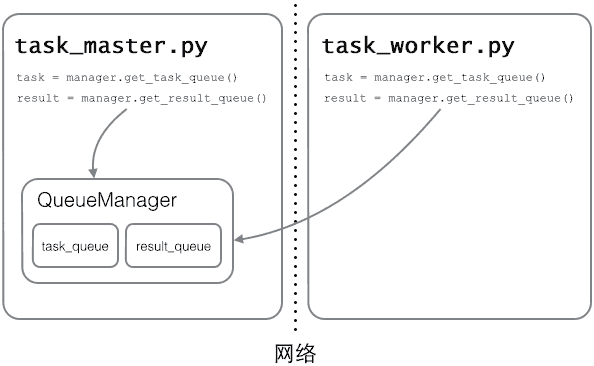`Queue`之所以能通过网络访问，就是通过`QueueManager`实现的。由于`QueueManager`管理的不止一个`Queue`，所以，要给每个`Queue`的网络调用接口起个名字，比如`get_task_queue`

`authkey`有什么用？这是为了保证两台机器正常通信，不被其他机器恶意干扰。如果`task_worker.py``authkey``task_master.py``authkey`不一致，肯定连接不上。

#### 小结

Python的分布式进程接口简单，封装良好，适合需要把繁重任务分布到多台机器的环境下。

# 正则表达式

1. 创建一个匹配Email的正则表达式；

2. 用该正则表达式去匹配用户的输入来判断是否合法。

• `'00\d'`可以匹配`'007'`，但无法匹配`'00A'`

• `'\d\d\d'`可以匹配`'010'`

• `'\w\w\d'`可以匹配`'py3'`

`.`可以匹配任意字符，所以：

• `'py.'`可以匹配`'pyc'``'pyo'``'py!'`等等。

1. `\d{3}`表示匹配3个数字，例如`'010'`

2. `\s`可以匹配一个空格（也包括Tab等空白符），所以`\s+`表示至少有一个空格，例如匹配`' '``' '`等；

3. `\d{3,8}`表示3-8个数字，例如`'1234567'`

#### 进阶

• `[0-9a-zA-Z\_]`可以匹配一个数字、字母或者下划线；

• `[0-9a-zA-Z\_]+`可以匹配至少由一个数字、字母或者下划线组成的字符串，比如`'a100'``'0_Z'``'Py3000'`等等；

• `[a-zA-Z\_][0-9a-zA-Z\_]*`可以匹配由字母或下划线开头，后接任意个由一个数字、字母或者下划线组成的字符串，也就是Python合法的变量；

• `[a-zA-Z\_][0-9a-zA-Z\_]{0, 19}`更精确地限制了变量的长度是1-20个字符（前面1个字符+后面最多19个字符）。

`A|B`可以匹配A或B，所以`[P|p]ython`可以匹配`'Python'`或者`'python'`

`^`表示行的开头，`^\d`表示必须以数字开头。

`\$`表示行的结束，`\d\$`表示必须以数字结束。

#### re模块

``````s = 'ABC\\-001' # Python的字符串
# 对应的正则表达式字符串变成：
# 'ABC\-001'
``````

``````s = r'ABC\-001' # Python的字符串
# 对应的正则表达式字符串不变：
# 'ABC\-001'
``````

``````>>> import re
>>> re.match(r'^\d{3}\-\d{3,8}\$', '010-12345')
<_sre.SRE_Match object; span=(0, 9), match='010-12345'>
>>> re.match(r'^\d{3}\-\d{3,8}\$', '010 12345')
>>>
``````

`match()`方法判断是否匹配，如果匹配成功，返回一个`Match`对象，否则返回`None`。常见的判断方法就是：

``````test = '用户输入的字符串'
if re.match(r'正则表达式', test):
print('ok')
else:
print('failed')
``````

#### 切分字符串

``````>>> 'a b   c'.split(' ')
['a', 'b', '', '', 'c']
``````

``````>>> re.split(r'\s+', 'a b   c')
['a', 'b', 'c']
``````

``````>>> re.split(r'[\s\,]+', 'a,b, c  d')
['a', 'b', 'c', 'd']
``````

``````>>> re.split(r'[\s\,\;]+', 'a,b;; c  d')
['a', 'b', 'c', 'd']
``````

#### 分组

`^(\d{3})-(\d{3,8})\$`分别定义了两个组，可以直接从匹配的字符串中提取出区号和本地号码：

``````>>> m = re.match(r'^(\d{3})-(\d{3,8})\$', '010-12345')
>>> m
<_sre.SRE_Match object; span=(0, 9), match='010-12345'>
>>> m.group(0)
'010-12345'
>>> m.group(1)
'010'
>>> m.group(2)
'12345'
``````

``````>>> t = '19:05:30'
>>> m = re.match(r'^(0[0-9]|1[0-9]|2[0-3]|[0-9])\:(0[0-9]|1[0-9]|2[0-9]|3[0-9]|4[0-9]|5[0-9]|[0-9])\:(0[0-9]|1[0-9]|2[0-9]|3[0-9]|4[0-9]|5[0-9]|[0-9])\$', t)
>>> m.groups()
('19', '05', '30')
``````

``````'^(0[1-9]|1[0-2]|[0-9])-(0[1-9]|1[0-9]|2[0-9]|3[0-1]|[0-9])\$'
``````

#### 贪婪匹配

``````>>> re.match(r'^(\d+)(0*)\$', '102300').groups()
('102300', '')
``````

``````>>> re.match(r'^(\d+?)(0*)\$', '102300').groups()
('1023', '00')
``````

#### 编译

1. 编译正则表达式，如果正则表达式的字符串本身不合法，会报错；

2. 用编译后的正则表达式去匹配字符串。

``````>>> import re
# 编译:
>>> re_telephone = re.compile(r'^(\d{3})-(\d{3,8})\$')
# 使用：
>>> re_telephone.match('010-12345').groups()
('010', '12345')
>>> re_telephone.match('010-8086').groups()
('010', '8086')
``````

#### 练习

``````someone@gmail.com
bill.gates@microsoft.com
``````

``````<Tom Paris> tom@voyager.org
``````

regex.py

# 常用内建模块

Python之所以自称“batteries included”，就是因为内置了许多非常有用的模块，无需额外安装和配置，即可直接使用。

## datetime

datetime是Python处理日期和时间的标准库。

#### 获取当前日期和时间

``````>>> from datetime import datetime
>>> now = datetime.now() # 获取当前datetime
>>> print(now)
2015-05-18 16:28:07.198690
>>> print(type(now))
<class 'datetime.datetime'>
``````

`datetime.now()`返回当前日期和时间，其类型是`datetime`

#### 获取指定日期和时间

``````>>> from datetime import datetime
>>> dt = datetime(2015, 4, 19, 12, 20) # 用指定日期时间创建datetime
>>> print(dt)
2015-04-19 12:20:00
``````

#### datetime转换为timestamp

``````timestamp = 0 = 1970-1-1 00:00:00 UTC+0:00
``````

``````timestamp = 0 = 1970-1-1 08:00:00 UTC+8:00
``````

``````>>> from datetime import datetime
>>> dt = datetime(2015, 4, 19, 12, 20) # 用指定日期时间创建datetime
>>> dt.timestamp() # 把timestamp转换为datetime
1429417200.0
``````

#### timestamp转换为datetime

``````>>> from datetime import datetime
>>> t = 1429417200.0
>>> print(datetime.fromtimestamp(t))
2015-04-19 12:20:00
``````

``````2015-04-19 12:20:00
``````

``````2015-04-19 12:20:00 UTC+8:00
``````

``````2015-04-19 04:20:00 UTC+0:00
``````

timestamp也可以直接被转换到UTC标准时区的时间：

``````>>> from datetime import datetime
>>> t = 1429417200.0
>>> print(datetime.fromtimestamp(t)) # 本地时间
2015-04-19 12:20:00
>>> print(datetime.utcfromtimestamp(t)) # UTC时间
2015-04-19 04:20:00
``````

#### str转换为datetime

``````>>> from datetime import datetime
>>> cday = datetime.strptime('2015-6-1 18:19:59', '%Y-%m-%d %H:%M:%S')
>>> print(cday)
2015-06-01 18:19:59
``````

#### datetime转换为str

``````>>> from datetime import datetime
>>> now = datetime.now()
>>> print(now.strftime('%a, %b %d %H:%M'))
Mon, May 05 16:28
``````

#### datetime加减

``````>>> from datetime import datetime, timedelta
>>> now = datetime.now()
>>> now
datetime.datetime(2015, 5, 18, 16, 57, 3, 540997)
>>> now + timedelta(hours=10)
datetime.datetime(2015, 5, 19, 2, 57, 3, 540997)
>>> now - timedelta(days=1)
datetime.datetime(2015, 5, 17, 16, 57, 3, 540997)
>>> now + timedelta(days=2, hours=12)
datetime.datetime(2015, 5, 21, 4, 57, 3, 540997)
``````

#### 本地时间转换为UTC时间

``````>>> from datetime import datetime, timedelta, timezone
>>> tz_utc_8 = timezone(timedelta(hours=8)) # 创建时区UTC+8:00
>>> now = datetime.now()
>>> now
datetime.datetime(2015, 5, 18, 17, 2, 10, 871012)
>>> dt = now.replace(tzinfo=tz_utc_8) # 强制设置为UTC+8:00
>>> dt
datetime.datetime(2015, 5, 18, 17, 2, 10, 871012, tzinfo=datetime.timezone(datetime.timedelta(0, 28800)))
``````

#### 时区转换

``````# 拿到UTC时间，并强制设置时区为UTC+0:00:
>>> utc_dt = datetime.utcnow().replace(tzinfo=timezone.utc)
>>> print(utc_dt)
2015-05-18 09:05:12.377316+00:00
# astimezone()将转换时区为北京时间:
>>> bj_dt = utc_dt.astimezone(timezone(timedelta(hours=8)))
>>> print(bj_dt)
2015-05-18 17:05:12.377316+08:00
# astimezone()将转换时区为东京时间:
>>> tokyo_dt = utc_dt.astimezone(timezone(timedelta(hours=9)))
>>> print(tokyo_dt)
2015-05-18 18:05:12.377316+09:00
# astimezone()将bj_dt转换时区为东京时间:
>>> tokyo_dt2 = bj_dt.astimezone(timezone(timedelta(hours=9)))
>>> print(tokyo_dt2)
2015-05-18 18:05:12.377316+09:00
``````

#### 小结

`datetime`表示的时间需要时区信息才能确定一个特定的时间，否则只能视为本地时间。

#### 练习

```# -*- coding:utf-8 -*-

import re
from datetime import datetime, timezone, timedelta

def to_timestamp(dt_str, tz_str):
----
pass
----
# 测试:

t1 = to_timestamp('2015-6-1 08:10:30', 'UTC+7:00')
assert t1 == 1433121030.0, t1

t2 = to_timestamp('2015-5-31 16:10:30', 'UTC-09:00')
assert t2 == 1433121030.0, t2

print('Pass')
```

use_datetime.py

## collections

collections是Python内建的一个集合模块，提供了许多有用的集合类。

#### namedtuple

``````>>> p = (1, 2)
``````

``````>>> from collections import namedtuple
>>> Point = namedtuple('Point', ['x', 'y'])
>>> p = Point(1, 2)
>>> p.x
1
>>> p.y
2
``````

`namedtuple`是一个函数，它用来创建一个自定义的`tuple`对象，并且规定了`tuple`元素的个数，并可以用属性而不是索引来引用`tuple`的某个元素。

``````>>> isinstance(p, Point)
True
>>> isinstance(p, tuple)
True
``````

``````# namedtuple('名称', [属性list]):
Circle = namedtuple('Circle', ['x', 'y', 'r'])
``````

#### deque

deque是为了高效实现插入和删除操作的双向列表，适合用于队列和栈：

``````>>> from collections import deque
>>> q = deque(['a', 'b', 'c'])
>>> q.append('x')
>>> q.appendleft('y')
>>> q
deque(['y', 'a', 'b', 'c', 'x'])
``````

`deque`除了实现list的`append()``pop()`外，还支持`appendleft()``popleft()`，这样就可以非常高效地往头部添加或删除元素。

#### defaultdict

``````>>> from collections import defaultdict
>>> dd = defaultdict(lambda: 'N/A')
>>> dd['key1'] = 'abc'
>>> dd['key1'] # key1存在
'abc'
>>> dd['key2'] # key2不存在，返回默认值
'N/A'
``````

#### OrderedDict

``````>>> from collections import OrderedDict
>>> d = dict([('a', 1), ('b', 2), ('c', 3)])
>>> d # dict的Key是无序的
{'a': 1, 'c': 3, 'b': 2}
>>> od = OrderedDict([('a', 1), ('b', 2), ('c', 3)])
>>> od # OrderedDict的Key是有序的
OrderedDict([('a', 1), ('b', 2), ('c', 3)])
``````

``````>>> od = OrderedDict()
>>> od['z'] = 1
>>> od['y'] = 2
>>> od['x'] = 3
>>> list(od.keys()) # 按照插入的Key的顺序返回
['z', 'y', 'x']
``````

`OrderedDict`可以实现一个FIFO（先进先出）的dict，当容量超出限制时，先删除最早添加的Key：

``````from collections import OrderedDict

class LastUpdatedOrderedDict(OrderedDict):

def __init__(self, capacity):
super(LastUpdatedOrderedDict, self).__init__()
self._capacity = capacity

def __setitem__(self, key, value):
containsKey = 1 if key in self else 0
if len(self) - containsKey >= self._capacity:
last = self.popitem(last=False)
print('remove:', last)
if containsKey:
del self[key]
print('set:', (key, value))
else:
OrderedDict.__setitem__(self, key, value)
``````

#### Counter

`Counter`是一个简单的计数器，例如，统计字符出现的个数：

``````>>> from collections import Counter
>>> c = Counter()
>>> for ch in 'programming':
...     c[ch] = c[ch] + 1
...
>>> c
Counter({'g': 2, 'm': 2, 'r': 2, 'a': 1, 'i': 1, 'o': 1, 'n': 1, 'p': 1})
``````

`Counter`实际上也是`dict`的一个子类，上面的结果可以看出，字符`'g'``'m'``'r'`各出现了两次，其他字符各出现了一次。

#### 小结

`collections`模块提供了一些有用的集合类，可以根据需要选用。

#### 参考源码

use_collections.py

## base64

Base64是一种用64个字符来表示任意二进制数据的方法。

Base64的原理很简单，首先，准备一个包含64个字符的数组：

``````['A', 'B', 'C', ... 'a', 'b', 'c', ... '0', '1', ... '+', '/']
``````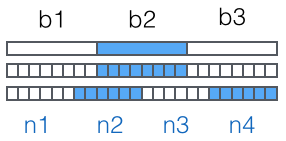Python内置的`base64`可以直接进行base64的编解码：

``````>>> import base64
>>> base64.b64encode(b'binary\x00string')
b'YmluYXJ5AHN0cmluZw=='
>>> base64.b64decode(b'YmluYXJ5AHN0cmluZw==')
b'binary\x00string'
``````

``````>>> base64.b64encode(b'i\xb7\x1d\xfb\xef\xff')
b'abcd++//'
>>> base64.urlsafe_b64encode(b'i\xb7\x1d\xfb\xef\xff')
b'abcd--__'
>>> base64.urlsafe_b64decode('abcd--__')
b'i\xb7\x1d\xfb\xef\xff'
``````

Base64是一种通过查表的编码方法，不能用于加密，即使使用自定义的编码表也不行。

``````# 标准Base64:
'abcd' -> 'YWJjZA=='
# 自动去掉=:
'abcd' -> 'YWJjZA'
``````

#### 练习

```# -*- coding: utf-8 -*-

import base64

def safe_base64_decode(s):
----
pass
----
# 测试:
assert b'abcd' == safe_base64_decode(b'YWJjZA=='), safe_base64_decode('YWJjZA==')
assert b'abcd' == safe_base64_decode(b'YWJjZA'), safe_base64_decode('YWJjZA')
print('Pass')
```

do_base64.py

## struct

``````>>> n = 10240099
>>> b1 = (n & 0xff000000) >> 24
>>> b2 = (n & 0xff0000) >> 16
>>> b3 = (n & 0xff00) >> 8
>>> b4 = n & 0xff
>>> bs = bytes([b1, b2, b3, b4])
>>> bs
b'\x00\x9c@c'
``````

`struct``pack`函数把任意数据类型变成`bytes`

``````>>> import struct
>>> struct.pack('>I', 10240099)
b'\x00\x9c@c'
``````

`pack`的第一个参数是处理指令，`'>I'`的意思是：

`>`表示字节顺序是big-endian，也就是网络序，`I`表示4字节无符号整数。

`unpack``bytes`变成相应的数据类型：

``````>>> struct.unpack('>IH', b'\xf0\xf0\xf0\xf0\x80\x80')
(4042322160, 32896)
``````

`struct`模块定义的数据类型可以参考Python官方文档：

https://docs.python.org/3/library/struct.html#format-characters

Windows的位图文件（.bmp）是一种非常简单的文件格式，我们来用`struct`分析一下。

``````>>> s = b'\x42\x4d\x38\x8c\x0a\x00\x00\x00\x00\x00\x36\x00\x00\x00\x28\x00\x00\x00\x80\x02\x00\x00\x68\x01\x00\x00\x01\x00\x18\x00'
``````

BMP格式采用小端方式存储数据，文件头的结构按顺序如下：

``````>>> struct.unpack('<ccIIIIIIHH', s)
(b'B', b'M', 691256, 0, 54, 40, 640, 360, 1, 24)
``````

check_bmp.py

## hashlib

#### 摘要算法简介

Python的hashlib提供了常见的摘要算法，如MD5，SHA1等等。

``````import hashlib

md5 = hashlib.md5()
md5.update('how to use md5 in python hashlib?'.encode('utf-8'))
print(md5.hexdigest())
``````

``````d26a53750bc40b38b65a520292f69306
``````

``````import hashlib

md5 = hashlib.md5()
md5.update('how to use md5 in '.encode('utf-8'))
md5.update('python hashlib?'.encode('utf-8'))
print(md5.hexdigest())
``````

MD5是最常见的摘要算法，速度很快，生成结果是固定的128 bit字节，通常用一个32位的16进制字符串表示。

``````import hashlib

sha1 = hashlib.sha1()
sha1.update('how to use sha1 in '.encode('utf-8'))
sha1.update('python hashlib?'.encode('utf-8'))
print(sha1.hexdigest())
``````

SHA1的结果是160 bit字节，通常用一个40位的16进制字符串表示。

#### 摘要算法应用

``````name    | password
--------+----------
michael | 123456
bob     | abc999
alice   | alice2008
``````

``````username | password
---------+---------------------------------
alice    | 99b1c2188db85afee403b1536010c2c9
``````

#### 练习

``````def calc_md5(password):
pass
``````

``````db = {
'alice': '99b1c2188db85afee403b1536010c2c9'
}

pass
``````

``````'e10adc3949ba59abbe56e057f20f883e': '123456'
'21218cca77804d2ba1922c33e0151105': '888888'
``````

``````def calc_md5(password):
``````

#### 练习

``````db = {}

``````

``````def login(username, password):
pass
``````

use_hashlib.py

## itertools

Python的内建模块`itertools`提供了非常有用的用于操作迭代对象的函数。

``````>>> import itertools
>>> natuals = itertools.count(1)
>>> for n in natuals:
...     print(n)
...
1
2
3
...
``````

`cycle()`会把传入的一个序列无限重复下去：

``````>>> import itertools
>>> cs = itertools.cycle('ABC') # 注意字符串也是序列的一种
>>> for c in cs:
...     print(c)
...
'A'
'B'
'C'
'A'
'B'
'C'
...
``````

`repeat()`负责把一个元素无限重复下去，不过如果提供第二个参数就可以限定重复次数：

``````>>> ns = itertools.repeat('A', 3)
>>> for n in ns:
...     print(n)
...
A
A
A
``````

``````>>> natuals = itertools.count(1)
>>> ns = itertools.takewhile(lambda x: x <= 10, natuals)
>>> list(ns)
[1, 2, 3, 4, 5, 6, 7, 8, 9, 10]
``````

`itertools`提供的几个迭代器操作函数更加有用：

#### chain()

`chain()`可以把一组迭代对象串联起来，形成一个更大的迭代器：

``````>>> for c in itertools.chain('ABC', 'XYZ'):
...     print(c)
# 迭代效果：'A' 'B' 'C' 'X' 'Y' 'Z'
``````

#### groupby()

`groupby()`把迭代器中相邻的重复元素挑出来放在一起：

``````>>> for key, group in itertools.groupby('AAABBBCCAAA'):
...     print(key, list(group))
...
A ['A', 'A', 'A']
B ['B', 'B', 'B']
C ['C', 'C']
A ['A', 'A', 'A']
``````

``````>>> for key, group in itertools.groupby('AaaBBbcCAAa', lambda c: c.upper()):
...     print(key, list(group))
...
A ['A', 'a', 'a']
B ['B', 'B', 'b']
C ['c', 'C']
A ['A', 'A', 'a']
``````

#### 小结

`itertools`模块提供的全部是处理迭代功能的函数，它们的返回值不是list，而是`Iterator`，只有用`for`循环迭代的时候才真正计算。

use_itertools.py

## XML

XML虽然比JSON复杂，在Web中应用也不如以前多了，不过仍有很多地方在用，所以，有必要了解如何操作XML。

#### DOM vs SAX

``````<a href="/">python</a>
``````

1. start_element事件，在读取`<a href="/">`时；

2. char_data事件，在读取`python`时；

3. end_element事件，在读取`</a>`时。

``````from xml.parsers.expat import ParserCreate

class DefaultSaxHandler(object):
def start_element(self, name, attrs):
print('sax:start_element: %s, attrs: %s' % (name, str(attrs)))

def end_element(self, name):
print('sax:end_element: %s' % name)

def char_data(self, text):
print('sax:char_data: %s' % text)

xml = r'''<?xml version="1.0"?>
<ol>
<li><a href="/python">Python</a></li>
<li><a href="/ruby">Ruby</a></li>
</ol>
'''

handler = DefaultSaxHandler()
parser = ParserCreate()
parser.StartElementHandler = handler.start_element
parser.EndElementHandler = handler.end_element
parser.CharacterDataHandler = handler.char_data
parser.Parse(xml)
``````

``````L = []
L.append(r'<?xml version="1.0"?>')
L.append(r'<root>')
L.append(encode('some & data'))
L.append(r'</root>')
return ''.join(L)
``````

#### 练习

```# -*- coding:utf-8 -*-

from xml.parsers.expat import ParserCreate
----
class WeatherSaxHandler(object):
pass

def parse_weather(xml):
return {
'city': 'Beijing',
'country': 'China',
'today': {
'text': 'Partly Cloudy',
'low': 20,
'high': 33
},
'tomorrow': {
'text': 'Sunny',
'low': 21,
'high': 34
}
}
----
# 测试:
data = r'''<?xml version="1.0" encoding="UTF-8" standalone="yes" ?>
<channel>
<title>Yahoo! Weather - Beijing, CN</title>
<lastBuildDate>Wed, 27 May 2015 11:00 am CST</lastBuildDate>
<yweather:location city="Beijing" region="" country="China"/>
<yweather:units temperature="C" distance="km" pressure="mb" speed="km/h"/>
<yweather:wind chill="28" direction="180" speed="14.48" />
<yweather:atmosphere humidity="53" visibility="2.61" pressure="1006.1" rising="0" />
<yweather:astronomy sunrise="4:51 am" sunset="7:32 pm"/>
<item>
<geo:lat>39.91</geo:lat>
<geo:long>116.39</geo:long>
<pubDate>Wed, 27 May 2015 11:00 am CST</pubDate>
<yweather:condition text="Haze" code="21" temp="28" date="Wed, 27 May 2015 11:00 am CST" />
<yweather:forecast day="Wed" date="27 May 2015" low="20" high="33" text="Partly Cloudy" code="30" />
<yweather:forecast day="Thu" date="28 May 2015" low="21" high="34" text="Sunny" code="32" />
<yweather:forecast day="Fri" date="29 May 2015" low="18" high="25" text="AM Showers" code="39" />
<yweather:forecast day="Sat" date="30 May 2015" low="18" high="32" text="Sunny" code="32" />
<yweather:forecast day="Sun" date="31 May 2015" low="20" high="37" text="Sunny" code="32" />
</item>
</channel>
'''
weather = parse_weather(data)
assert weather['city'] == 'Beijing', weather['city']
assert weather['country'] == 'China', weather['country']
assert weather['today']['text'] == 'Partly Cloudy', weather['today']['text']
assert weather['today']['low'] == 20, weather['today']['low']
assert weather['today']['high'] == 33, weather['today']['high']
assert weather['tomorrow']['text'] == 'Sunny', weather['tomorrow']['text']
assert weather['tomorrow']['low'] == 21, weather['tomorrow']['low']
assert weather['tomorrow']['high'] == 34, weather['tomorrow']['high']
print('Weather:', str(weather))
```

use_sax.py

## HTMLParser

HTML本质上是XML的子集，但是HTML的语法没有XML那么严格，所以不能用标准的DOM或SAX来解析HTML。

``````from html.parser import HTMLParser
from html.entities import name2codepoint

class MyHTMLParser(HTMLParser):

def handle_starttag(self, tag, attrs):
print('<%s>' % tag)

def handle_endtag(self, tag):
print('</%s>' % tag)

def handle_startendtag(self, tag, attrs):
print('<%s/>' % tag)

def handle_data(self, data):
print(data)

def handle_comment(self, data):
print('<!--', data, '-->')

def handle_entityref(self, name):
print('&%s;' % name)

def handle_charref(self, name):
print('&#%s;' % name)

parser = MyHTMLParser()
parser.feed('''<html>
<body>
<!-- test html parser -->
<p>Some <a href=\"#\">html</a> HTML&nbsp;tutorial...<br>END</p>
</body></html>''')
``````

`feed()`方法可以多次调用，也就是不一定一次把整个HTML字符串都塞进去，可以一部分一部分塞进去。

#### 参考源码

use_htmlparser.py

## urllib

urllib提供了一系列用于操作URL的功能。

#### Get

urllib的`request`模块可以非常方便地抓取URL内容，也就是发送一个GET请求到指定的页面，然后返回HTTP的响应：

``````from urllib import request

with request.urlopen('https://api.douban.com/v2/book/2129650') as f:
print('Status:', f.status, f.reason)
print('%s: %s' % (k, v))
print('Data:', data.decode('utf-8'))
``````

``````Status: 200 OK
Server: nginx
Date: Tue, 26 May 2015 10:02:27 GMT
Content-Type: application/json; charset=utf-8
Content-Length: 2049
Connection: close
Expires: Sun, 1 Jan 2006 01:00:00 GMT
Pragma: no-cache
Cache-Control: must-revalidate, no-cache, private
X-DAE-Node: pidl1
Data: {"rating":{"max":10,"numRaters":16,"average":"7.4","min":0},"subtitle":"","author":["廖雪峰编著"],"pubdate":"2007-6","tags":[{"count":20,"name":"spring","title":"spring"}...}
``````

``````from urllib import request

req = request.Request('http://www.douban.com/')
req.add_header('User-Agent', 'Mozilla/6.0 (iPhone; CPU iPhone OS 8_0 like Mac OS X) AppleWebKit/536.26 (KHTML, like Gecko) Version/8.0 Mobile/10A5376e Safari/8536.25')
with request.urlopen(req) as f:
print('Status:', f.status, f.reason)
print('%s: %s' % (k, v))
``````

``````...
<meta name="viewport" content="width=device-width, user-scalable=no, initial-scale=1.0, minimum-scale=1.0, maximum-scale=1.0">
<meta name="format-detection" content="telephone=no">
...
``````

#### Post

``````from urllib import request, parse

email = input('Email: ')
('entry', 'mweibo'),
('client_id', ''),
('savestate', '1'),
('ec', ''),
('pagerefer', 'https://passport.weibo.cn/signin/welcome?entry=mweibo&r=http%3A%2F%2Fm.weibo.cn%2F')
])

req.add_header('User-Agent', 'Mozilla/6.0 (iPhone; CPU iPhone OS 8_0 like Mac OS X) AppleWebKit/536.26 (KHTML, like Gecko) Version/8.0 Mobile/10A5376e Safari/8536.25')

print('Status:', f.status, f.reason)
print('%s: %s' % (k, v))
``````

``````Status: 200 OK
Server: nginx/1.2.0
...
...
Data: {"retcode":20000000,"msg":"","data":{...,"uid":"1658384301"}}
``````

``````...
``````

#### Handler

``````proxy_handler = urllib.request.ProxyHandler({'http': 'http://www.example.com:3128/'})
proxy_auth_handler = urllib.request.ProxyBasicAuthHandler()
opener = urllib.request.build_opener(proxy_handler, proxy_auth_handler)
pass
``````

#### 小结

urllib提供的功能就是利用程序去执行各种HTTP请求。如果要模拟浏览器完成特定功能，需要把请求伪装成浏览器。伪装的方法是先监控浏览器发出的请求，再根据浏览器的请求头来伪装，`User-Agent`头就是用来标识浏览器的。

#### 练习

```from urllib import request, parse

def fetch_xml(url):
----
pass
----
# 测试
```

use_urllib.py

# 常用第三方模块

## PIL

PIL：Python Imaging Library，已经是Python平台事实上的图像处理标准库了。PIL功能非常强大，但API却非常简单易用。

#### 安装Pillow

``````\$ pip install pillow
``````

#### 操作图像

``````from PIL import Image

# 打开一个jpg图像文件，注意是当前路径:
im = Image.open('test.jpg')
# 获得图像尺寸:
w, h = im.size
print('Original image size: %sx%s' % (w, h))
# 缩放到50%:
im.thumbnail((w//2, h//2))
print('Resize image to: %sx%s' % (w//2, h//2))
# 把缩放后的图像用jpeg格式保存:
im.save('thumbnail.jpg', 'jpeg')
``````

``````from PIL import Image, ImageFilter

# 打开一个jpg图像文件，注意是当前路径:
im = Image.open('test.jpg')
# 应用模糊滤镜:
im2 = im.filter(ImageFilter.BLUR)
im2.save('blur.jpg', 'jpeg')
``````PIL的`ImageDraw`提供了一系列绘图方法，让我们可以直接绘图。比如要生成字母验证码图片：

``````from PIL import Image, ImageDraw, ImageFont, ImageFilter

import random

# 随机字母:
def rndChar():
return chr(random.randint(65, 90))

# 随机颜色1:
def rndColor():
return (random.randint(64, 255), random.randint(64, 255), random.randint(64, 255))

# 随机颜色2:
def rndColor2():
return (random.randint(32, 127), random.randint(32, 127), random.randint(32, 127))

# 240 x 60:
width = 60 * 4
height = 60
image = Image.new('RGB', (width, height), (255, 255, 255))
# 创建Font对象:
font = ImageFont.truetype('Arial.ttf', 36)
# 创建Draw对象:
draw = ImageDraw.Draw(image)
# 填充每个像素:
for x in range(width):
for y in range(height):
draw.point((x, y), fill=rndColor())
# 输出文字:
for t in range(4):
draw.text((60 * t + 10, 10), rndChar(), font=font, fill=rndColor2())
# 模糊:
image = image.filter(ImageFilter.BLUR)
image.save('code.jpg', 'jpeg')
``````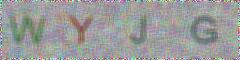``````IOError: cannot open resource
``````

``````'/Library/Fonts/Arial.ttf'
``````

#### 小结

PIL提供了操作图像的强大功能，可以通过简单的代码完成复杂的图像处理。

#### 参考源码

https://github.com/michaelliao/learn-python3/blob/master/samples/packages/pil/use_pil_resize.py

https://github.com/michaelliao/learn-python3/blob/master/samples/packages/pil/use_pil_blur.py

https://github.com/michaelliao/learn-python3/blob/master/samples/packages/pil/use_pil_draw.py

# virtualenv

``````\$ pip3 install virtualenv
``````

``````Mac:~ michael\$ mkdir myproject
Mac:~ michael\$ cd myproject/
Mac:myproject michael\$
``````

``````Mac:myproject michael\$ virtualenv --no-site-packages venv
Using base prefix '/usr/local/.../Python.framework/Versions/3.4'
New python executable in venv/bin/python3.4
Also creating executable in venv/bin/python
Installing setuptools, pip, wheel...done.
``````

``````Mac:myproject michael\$ source venv/bin/activate
(venv)Mac:myproject michael\$
``````

``````(venv)Mac:myproject michael\$ pip install jinja2
...
Successfully installed jinja2-2.7.3 markupsafe-0.23
(venv)Mac:myproject michael\$ python myapp.py
...
``````

`venv`环境下，用`pip`安装的包都被安装到`venv`这个环境下，系统Python环境不受任何影响。也就是说，`venv`环境是专门针对`myproject`这个应用创建的。

``````(venv)Mac:myproject michael\$ deactivate
Mac:myproject michael\$
``````

virtualenv是如何创建“独立”的Python运行环境的呢？原理很简单，就是把系统Python复制一份到virtualenv的环境，用命令`source venv/bin/activate`进入一个virtualenv环境时，virtualenv会修改相关环境变量，让命令`python``pip`均指向当前的virtualenv环境。

#### 小结

virtualenv为应用提供了隔离的Python运行环境，解决了不同应用间多版本的冲突问题。

# 图形界面

Python支持多种图形界面的第三方库，包括：

• Tk

• wxWidgets

• Qt

• GTK

#### Tkinter

Tk是一个图形库，支持多个操作系统，使用Tcl语言开发；

Tk会调用操作系统提供的本地GUI接口，完成最终的GUI。

#### 第一个GUI程序

``````from tkinter import *
``````

``````class Application(Frame):
def __init__(self, master=None):
Frame.__init__(self, master)
self.pack()
self.createWidgets()

def createWidgets(self):
self.helloLabel = Label(self, text='Hello, world!')
self.helloLabel.pack()
self.quitButton = Button(self, text='Quit', command=self.quit)
self.quitButton.pack()
``````

`pack()`方法把Widget加入到父容器中，并实现布局。`pack()`是最简单的布局，`grid()`可以实现更复杂的布局。

`createWidgets()`方法中，我们创建一个`Label`和一个`Button`，当Button被点击时，触发`self.quit()`使程序退出。

``````app = Application()
# 设置窗口标题:
app.master.title('Hello World')
# 主消息循环:
app.mainloop()
``````

GUI程序的主线程负责监听来自操作系统的消息，并依次处理每一条消息。因此，如果消息处理非常耗时，就需要在新线程中处理。#### 输入文本

``````from tkinter import *
import tkinter.messagebox as messagebox

class Application(Frame):
def __init__(self, master=None):
Frame.__init__(self, master)
self.pack()
self.createWidgets()

def createWidgets(self):
self.nameInput = Entry(self)
self.nameInput.pack()

def hello(self):
name = self.nameInput.get() or 'world'
messagebox.showinfo('Message', 'Hello, %s' % name)

app = Application()
# 设置窗口标题:
app.master.title('Hello World')
# 主消息循环:
app.mainloop()
``````#### 小结

Python内置的Tkinter可以满足基本的GUI程序的要求，如果是非常复杂的GUI程序，建议用操作系统原生支持的语言和库来编写。

hello_gui.py

# 网络编程## TCP/IP简介

IP协议负责把数据从一台计算机通过网络发送到另一台计算机。数据被分割成一小块一小块，然后通过IP包发送出去。由于互联网链路复杂，两台计算机之间经常有多条线路，因此，路由器就负责决定如何把一个IP包转发出去。IP包的特点是按块发送，途径多个路由，但不保证能到达，也不保证顺序到达。IP地址实际上是一个32位整数（称为IPv4），以字符串表示的IP地址如`192.168.0.1`实际上是把32位整数按8位分组后的数字表示，目的是便于阅读。

IPv6地址实际上是一个128位整数，它是目前使用的IPv4的升级版，以字符串表示类似于`2001:0db8:85a3:0042:1000:8a2e:0370:7334`

TCP协议则是建立在IP协议之上的。TCP协议负责在两台计算机之间建立可靠连接，保证数据包按顺序到达。TCP协议会通过握手建立连接，然后，对每个IP包编号，确保对方按顺序收到，如果包丢掉了，就自动重发。

## TCP编程

Socket是网络编程的一个抽象概念。通常我们用一个Socket表示“打开了一个网络链接”，而打开一个Socket需要知道目标计算机的IP地址和端口号，再指定协议类型即可。

#### 客户端

``````# 导入socket库:
import socket

# 创建一个socket:
s = socket.socket(socket.AF_INET, socket.SOCK_STREAM)
# 建立连接:
s.connect(('www.sina.com.cn', 80))
``````

``````s.connect(('www.sina.com.cn', 80))
``````

``````# 发送数据:
s.send(b'GET / HTTP/1.1\r\nHost: www.sina.com.cn\r\nConnection: close\r\n\r\n')
``````

TCP连接创建的是双向通道，双方都可以同时给对方发数据。但是谁先发谁后发，怎么协调，要根据具体的协议来决定。例如，HTTP协议规定客户端必须先发请求给服务器，服务器收到后才发数据给客户端。

``````# 接收数据:
buffer = []
while True:
# 每次最多接收1k字节:
d = s.recv(1024)
if d:
buffer.append(d)
else:
break
data = b''.join(buffer)
``````

``````# 关闭连接:
s.close()
``````

``````header, html = data.split(b'\r\n\r\n', 1)
# 把接收的数据写入文件:
with open('sina.html', 'wb') as f:
f.write(html)
``````

#### 服务器

``````s = socket.socket(socket.AF_INET, socket.SOCK_STREAM)
``````

``````# 监听端口:
s.bind(('127.0.0.1', 9999))
``````

``````s.listen(5)
print('Waiting for connection...')
``````

``````while True:
# 接受一个新连接:
# 创建新线程来处理TCP连接:
t.start()
``````

``````def tcplink(sock, addr):
print('Accept new connection from %s:%s...' % addr)
sock.send(b'Welcome!')
while True:
data = sock.recv(1024)
time.sleep(1)
if not data or data.decode('utf-8') == 'exit':
break
sock.send(('Hello, %s!' % data.decode('utf-8')).encode('utf-8'))
sock.close()
print('Connection from %s:%s closed.' % addr)
``````

``````s = socket.socket(socket.AF_INET, socket.SOCK_STREAM)
# 建立连接:
s.connect(('127.0.0.1', 9999))
# 接收欢迎消息:
print(s.recv(1024).decode('utf-8'))
for data in [b'Michael', b'Tracy', b'Sarah']:
# 发送数据:
s.send(data)
print(s.recv(1024).decode('utf-8'))
s.send(b'exit')
s.close()
``````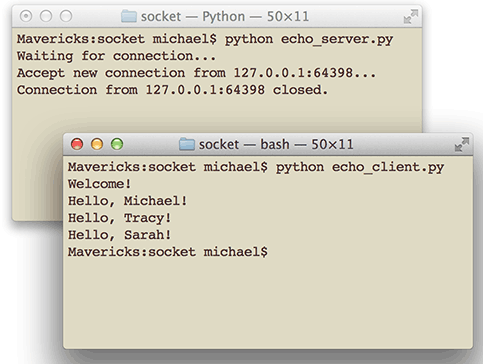do_tcp.py

## UDP编程

TCP是建立可靠连接，并且通信双方都可以以流的形式发送数据。相对TCP，UDP则是面向无连接的协议。

``````s = socket.socket(socket.AF_INET, socket.SOCK_DGRAM)
# 绑定端口:
s.bind(('127.0.0.1', 9999))
``````

``````print('Bind UDP on 9999...')
while True:
# 接收数据:
``````

`recvfrom()`方法返回数据和客户端的地址与端口，这样，服务器收到数据后，直接调用`sendto()`就可以把数据用UDP发给客户端。

``````s = socket.socket(socket.AF_INET, socket.SOCK_DGRAM)
for data in [b'Michael', b'Tracy', b'Sarah']:
# 发送数据:
s.sendto(data, ('127.0.0.1', 9999))
# 接收数据:
print(s.recv(1024).decode('utf-8'))
s.close()
``````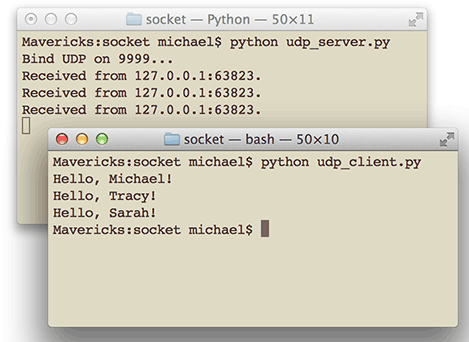#### 小结

UDP的使用与TCP类似，但是不需要建立连接。此外，服务器绑定UDP端口和TCP端口互不冲突，也就是说，UDP的9999端口与TCP的9999端口可以各自绑定。

udp_server.py

udp_client.py

# 电子邮件

Email的历史比Web还要久远，直到现在，Email也是互联网上应用非常广泛的服务。

Email从MUA发出去，不是直接到达对方电脑，而是发到MTA：Mail Transfer Agent——邮件传输代理，就是那些Email服务提供商，比如网易、新浪等等。由于我们自己的电子邮件是`163.com`，所以，Email首先被投递到网易提供的MTA，再由网易的MTA发到对方服务商，也就是新浪的MTA。这个过程中间可能还会经过别的MTA，但是我们不关心具体路线，我们只关心速度。

Email到达新浪的MTA后，由于对方使用的是`@sina.com`的邮箱，因此，新浪的MTA会把Email投递到邮件的最终目的地MDA：Mail Delivery Agent——邮件投递代理。Email到达MDA后，就静静地躺在新浪的某个服务器上，存放在某个文件或特殊的数据库里，我们将这个长期保存邮件的地方称之为电子邮箱。

``````发件人 -> MUA -> MTA -> MTA -> 若干个MTA -> MDA <- MUA <- 收件人
``````

1. 编写MUA把邮件发到MTA；

2. 编写MUA从MDA上收邮件。## SMTP发送邮件

SMTP是发送邮件的协议，Python内置对SMTP的支持，可以发送纯文本邮件、HTML邮件以及带附件的邮件。

Python对SMTP支持有`smtplib``email`两个模块，`email`负责构造邮件，`smtplib`负责发送邮件。

``````from email.mime.text import MIMEText
msg = MIMEText('hello, send by Python...', 'plain', 'utf-8')
``````

``````# 输入Email地址和口令:
# 输入收件人地址:
# 输入SMTP服务器地址:
smtp_server = input('SMTP server: ')

import smtplib
server = smtplib.SMTP(smtp_server, 25) # SMTP协议默认端口是25
server.set_debuglevel(1)
server.quit()
``````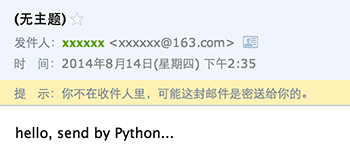1. 邮件没有主题；
2. 收件人的名字没有显示为友好的名字，比如`Mr Green <green@example.com>`
3. 明明收到了邮件，却提示不在收件人中。

``````from email import encoders
from email.mime.text import MIMEText

import smtplib

smtp_server = input('SMTP server: ')

msg = MIMEText('hello, send by Python...', 'plain', 'utf-8')

server = smtplib.SMTP(smtp_server, 25)
server.set_debuglevel(1)
server.quit()
``````

`msg['To']`接收的是字符串而不是list，如果有多个邮件地址，用`,`分隔即可。``````From: =?utf-8?b?UHl0aG9u54ix5aW96ICF?= <xxxxxx@163.com>
To: =?utf-8?b?566h55CG5ZGY?= <xxxxxx@qq.com>
Subject: =?utf-8?b?5p2l6IeqU01UUOeahOmXruWAmeKApuKApg==?=
``````

#### 发送HTML邮件

``````msg = MIMEText('<html><body><h1>Hello</h1>' +
'<p>send by <a href="http://www.python.org">Python</a>...</p>' +
'</body></html>', 'html', 'utf-8')
``````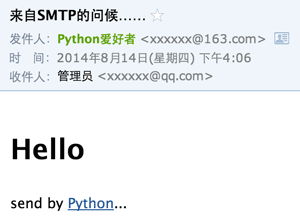#### 发送附件

``````# 邮件对象:
msg = MIMEMultipart()

# 邮件正文是MIMEText:
msg.attach(MIMEText('send with file...', 'plain', 'utf-8'))

# 添加附件就是加上一个MIMEBase，从本地读取一个图片:
# 设置附件的MIME和文件名，这里是png类型:
mime = MIMEBase('image', 'png', filename='test.png')
# 加上必要的头信息:
# 把附件的内容读进来:
# 用Base64编码:
encoders.encode_base64(mime)
# 添加到MIMEMultipart:
msg.attach(mime)
``````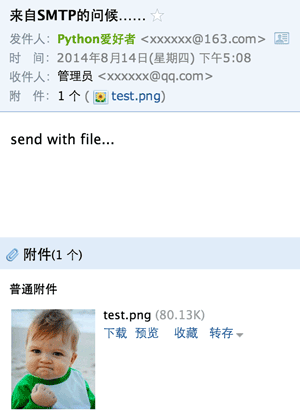#### 发送图片

``````msg.attach(MIMEText('<html><body><h1>Hello</h1>' +
'<p><img src="cid:0"></p>' +
'</body></html>', 'html', 'utf-8'))
``````#### 同时支持HTML和Plain格式

``````msg = MIMEMultipart('alternative')
msg['From'] = ...
msg['To'] = ...
msg['Subject'] = ...

msg.attach(MIMEText('hello', 'plain', 'utf-8'))
msg.attach(MIMEText('<html><body><h1>Hello</h1></body></html>', 'html', 'utf-8'))
# 正常发送msg对象...
``````

#### 加密SMTP

``````smtp_server = 'smtp.gmail.com'
smtp_port = 587
server = smtplib.SMTP(smtp_server, smtp_port)
server.starttls()
# 剩下的代码和前面的一模一样:
server.set_debuglevel(1)
...
``````

#### 小结

``````Message
+- MIMEBase
+- MIMEMultipart
+- MIMENonMultipart
+- MIMEMessage
+- MIMEText
+- MIMEImage
``````

send_mail.py

## POP3收取邮件

SMTP用于发送邮件，如果要收取邮件呢？

Python内置一个`poplib`模块，实现了POP3协议，可以直接用来收邮件。

#### 通过POP3下载邮件

POP3协议本身很简单，以下面的代码为例，我们来获取最新的一封邮件内容：

``````import poplib

# 输入邮件地址, 口令和POP3服务器地址:
email = input('Email: ')
pop3_server = input('POP3 server: ')

# 连接到POP3服务器:
server = poplib.POP3(pop3_server)
# 可以打开或关闭调试信息:
server.set_debuglevel(1)
# 可选:打印POP3服务器的欢迎文字:
print(server.getwelcome().decode('utf-8'))

# 身份认证:
server.user(email)

# stat()返回邮件数量和占用空间:
print('Messages: %s. Size: %s' % server.stat())
# list()返回所有邮件的编号:
resp, mails, octets = server.list()
# 可以查看返回的列表类似[b'1 82923', b'2 2184', ...]
print(mails)

# 获取最新一封邮件, 注意索引号从1开始:
index = len(mails)
resp, lines, octets = server.retr(index)

# lines存储了邮件的原始文本的每一行,
# 可以获得整个邮件的原始文本:
msg_content = b'\r\n'.join(lines).decode('utf-8')
# 稍后解析出邮件:
msg = Parser().parsestr(msg_content)

# 可以根据邮件索引号直接从服务器删除邮件:
# server.dele(index)
# 关闭连接:
server.quit()
``````

#### 解析邮件

``````from email.parser import Parser

import poplib
``````

``````msg = Parser().parsestr(msg_content)
``````

``````# indent用于缩进显示:
def print_info(msg, indent=0):
if indent == 0:
for header in ['From', 'To', 'Subject']:
if value:
value = decode_str(value)
else:
name = decode_str(hdr)
value = u'%s <%s>' % (name, addr)
print('%s%s: %s' % ('  ' * indent, header, value))
if (msg.is_multipart()):
for n, part in enumerate(parts):
print('%spart %s' % ('  ' * indent, n))
print('%s--------------------' % ('  ' * indent))
print_info(part, indent + 1)
else:
content_type = msg.get_content_type()
if content_type=='text/plain' or content_type=='text/html':
charset = guess_charset(msg)
if charset:
content = content.decode(charset)
print('%sText: %s' % ('  ' * indent, content + '...'))
else:
print('%sAttachment: %s' % ('  ' * indent, content_type))
``````

``````def decode_str(s):
if charset:
value = value.decode(charset)
return value
``````

`decode_header()`返回一个list，因为像`Cc``Bcc`这样的字段可能包含多个邮件地址，所以解析出来的会有多个元素。上面的代码我们偷了个懒，只取了第一个元素。

``````def guess_charset(msg):
charset = msg.get_charset()
if charset is None:
content_type = msg.get('Content-Type', '').lower()
pos = content_type.find('charset=')
if pos >= 0:
charset = content_type[pos + 8:].strip()
return charset
``````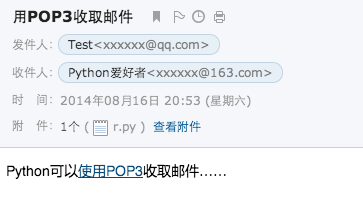``````+OK Welcome to coremail Mail Pop3 Server (163coms[...])
Messages: 126. Size: 27228317

From: Test <xxxxxx@qq.com>
To: Python爱好者 <xxxxxx@163.com>
Subject: 用POP3收取邮件
part 0
--------------------
part 0
--------------------
Text: Python可以使用POP3收取邮件……...
part 1
--------------------
Text: Python可以<a href="...">使用POP3</a>收取邮件……...
part 1
--------------------
Attachment: application/octet-stream
``````

fetch_mail.py

# 访问数据库

Michael99
Bob85
Bart59
Lisa87

``````Michael,99
Bob,85
Bart,59
Lisa,87
``````

``````[
{"name":"Michael","score":99},
{"name":"Bob","score":85},
{"name":"Bart","score":59},
{"name":"Lisa","score":87}
]
``````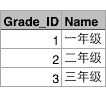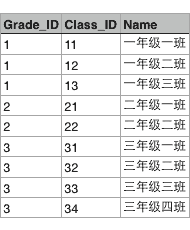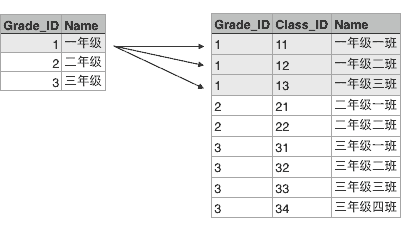``````SELECT * FROM classes WHERE grade_id = '1';
``````

``````---------+----------+----------
---------+----------+----------
1        | 11       | 一年级一班
---------+----------+----------
1        | 12       | 一年级二班
---------+----------+----------
1        | 13       | 一年级三班
---------+----------+----------
``````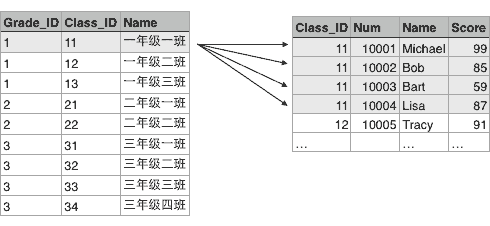#### 数据库类别

• Oracle，典型的高富帅；

• SQL Server，微软自家产品，Windows定制专款；

• DB2，IBM的产品，听起来挺高端；

• Sybase，曾经跟微软是好基友，后来关系破裂，现在家境惨淡。

• MySQL，大家都在用，一般错不了；

• PostgreSQL，学术气息有点重，其实挺不错，但知名度没有MySQL高；

• sqlite，嵌入式数据库，适合桌面和移动应用。

## 使用SQLite

SQLite是一种嵌入式数据库，它的数据库就是一个文件。由于SQLite本身是C写的，而且体积很小，所以，经常被集成到各种应用程序中，甚至在iOS和Android的App中都可以集成。

Python就内置了SQLite3，所以，在Python中使用SQLite，不需要安装任何东西，直接使用。

Python定义了一套操作数据库的API接口，任何数据库要连接到Python，只需要提供符合Python标准的数据库驱动即可。

``````# 导入SQLite驱动:
>>> import sqlite3
# 连接到SQLite数据库
# 数据库文件是test.db
# 如果文件不存在，会自动在当前目录创建:
>>> conn = sqlite3.connect('test.db')
# 创建一个Cursor:
>>> cursor = conn.cursor()
# 执行一条SQL语句，创建user表:
>>> cursor.execute('create table user (id varchar(20) primary key, name varchar(20))')
<sqlite3.Cursor object at 0x10f8aa260>
# 继续执行一条SQL语句，插入一条记录:
>>> cursor.execute('insert into user (id, name) values (\'1\', \'Michael\')')
<sqlite3.Cursor object at 0x10f8aa260>
# 通过rowcount获得插入的行数:
>>> cursor.rowcount
1
# 关闭Cursor:
>>> cursor.close()
# 提交事务:
>>> conn.commit()
# 关闭Connection:
>>> conn.close()
``````

``````>>> conn = sqlite3.connect('test.db')
>>> cursor = conn.cursor()
# 执行查询语句:
>>> cursor.execute('select * from user where id=?', '1')
<sqlite3.Cursor object at 0x10f8aa340>
# 获得查询结果集:
>>> values = cursor.fetchall()
>>> values
[('1', 'Michael')]
>>> cursor.close()
>>> conn.close()
``````

``````cursor.execute('select * from user where id=?', '1')
``````

SQLite支持常见的标准SQL语句以及几种常见的数据类型。具体文档请参阅SQLite官方网站。

#### 练习

```# -*- coding: utf-8 -*-

import os, sqlite3

db_file = os.path.join(os.path.dirname(__file__), 'test.db')
if os.path.isfile(db_file):
os.remove(db_file)

# 初始数据:
conn = sqlite3.connect(db_file)
cursor = conn.cursor()
cursor.execute('create table user(id varchar(20) primary key, name varchar(20), score int)')
cursor.execute(r"insert into user values ('A-001', 'Adam', 95)")
cursor.execute(r"insert into user values ('A-002', 'Bart', 62)")
cursor.execute(r"insert into user values ('A-003', 'Lisa', 78)")
cursor.close()
conn.commit()
conn.close()

def get_score_in(low, high):
' 返回指定分数区间的名字，按分数从低到高排序 '
----
pass
----
# 测试:
assert get_score_in(80, 95) == ['Adam'], get_score_in(80, 95)
assert get_score_in(60, 80) == ['Bart', 'Lisa'], get_score_in(60, 80)
assert get_score_in(60, 100) == ['Bart', 'Lisa', 'Adam'], get_score_in(60, 100)

print('Pass')
```

do_sqlite.py

## 使用MySQL

MySQL是Web世界中使用最广泛的数据库服务器。SQLite的特点是轻量级、可嵌入，但不能承受高并发访问，适合桌面和移动应用。而MySQL是为服务器端设计的数据库，能承受高并发访问，同时占用的内存也远远大于SQLite。

#### 安装MySQL

``````[client]
default-character-set = utf8

[mysqld]
default-storage-engine = INNODB
character-set-server = utf8
collation-server = utf8_general_ci
``````

``````\$ mysql -u root -p
Welcome to the MySQL monitor...
...

mysql> show variables like '%char%';
+--------------------------+--------------------------------------------------------+
| Variable_name            | Value                                                  |
+--------------------------+--------------------------------------------------------+
| character_set_client     | utf8                                                   |
| character_set_connection | utf8                                                   |
| character_set_database   | utf8                                                   |
| character_set_filesystem | binary                                                 |
| character_set_results    | utf8                                                   |
| character_set_server     | utf8                                                   |
| character_set_system     | utf8                                                   |
| character_sets_dir       | /usr/local/mysql-5.1.65-osx10.6-x86_64/share/charsets/ |
+--------------------------+--------------------------------------------------------+
8 rows in set (0.00 sec)
``````

#### 安装MySQL驱动

``````\$ pip install mysql-connector-python --allow-external mysql-connector-python
``````

``````# 导入MySQL驱动:
>>> import mysql.connector
>>> cursor = conn.cursor()
# 创建user表:
>>> cursor.execute('create table user (id varchar(20) primary key, name varchar(20))')
# 插入一行记录，注意MySQL的占位符是%s:
>>> cursor.execute('insert into user (id, name) values (%s, %s)', ['1', 'Michael'])
>>> cursor.rowcount
1
# 提交事务:
>>> conn.commit()
>>> cursor.close()
# 运行查询:
>>> cursor = conn.cursor()
>>> cursor.execute('select * from user where id = %s', ['1'])
>>> values = cursor.fetchall()
>>> values
[('1', 'Michael')]
# 关闭Cursor和Connection:
>>> cursor.close()
True
>>> conn.close()
``````

#### 小结

• 执行INSERT等操作后要调用`commit()`提交事务；

• MySQL的SQL占位符是`%s`

do_mysql.py

## 使用SQLAlchemy

``````[
('1', 'Michael'),
('2', 'Bob'),
]
``````

Python的DB-API返回的数据结构就是像上面这样表示的。

``````class User(object):
def __init__(self, id, name):
self.id = id
self.name = name

[
User('1', 'Michael'),
User('2', 'Bob'),
]
``````

``````\$ pip install sqlalchemy
``````

``````# 导入:
from sqlalchemy import Column, String, create_engine
from sqlalchemy.orm import sessionmaker
from sqlalchemy.ext.declarative import declarative_base

# 创建对象的基类:
Base = declarative_base()

# 定义User对象:
class User(Base):
# 表的名字:
__tablename__ = 'user'

# 表的结构:
id = Column(String(20), primary_key=True)
name = Column(String(20))

# 初始化数据库连接:
# 创建DBSession类型:
DBSession = sessionmaker(bind=engine)
``````

``````class School(Base):
__tablename__ = 'school'
id = ...
name = ...
``````

`create_engine()`用来初始化数据库连接。SQLAlchemy用一个字符串表示连接信息：

``````'数据库类型+数据库驱动名称://用户名:口令@机器地址:端口号/数据库名'
``````

``````# 创建session对象:
session = DBSession()
# 创建新User对象:
new_user = User(id='5', name='Bob')
# 添加到session:
# 提交即保存到数据库:
session.commit()
# 关闭session:
session.close()
``````

``````# 创建Session:
session = DBSession()
# 创建Query查询，filter是where条件，最后调用one()返回唯一行，如果调用all()则返回所有行:
user = session.query(User).filter(User.id=='5').one()
# 打印类型和对象的name属性:
print('type:', type(user))
print('name:', user.name)
# 关闭Session:
session.close()
``````

``````type: <class '__main__.User'>
name: Bob
``````

``````class User(Base):
__tablename__ = 'user'

id = Column(String(20), primary_key=True)
name = Column(String(20))
# 一对多:
books = relationship('Book')

class Book(Base):
__tablename__ = 'book'

id = Column(String(20), primary_key=True)
name = Column(String(20))
# “多”的一方的book表是通过外键关联到user表的:
user_id = Column(String(20), ForeignKey('user.id'))
``````

#### 小结

ORM框架的作用就是把数据库表的一行记录与一个对象互相做自动转换。

do_sqlalchemy.py

# Web开发

Web应用开发可以说是目前软件开发中最重要的部分。Web开发也经历了好几个阶段：

1. 静态Web页面：由文本编辑器直接编辑并生成静态的HTML页面，如果要修改Web页面的内容，就需要再次编辑HTML源文件，早期的互联网Web页面就是静态的；

2. CGI：由于静态Web页面无法与用户交互，比如用户填写了一个注册表单，静态Web页面就无法处理。要处理用户发送的动态数据，出现了Common Gateway Interface，简称CGI，用C/C++编写。

3. ASP/JSP/PHP：由于Web应用特点是修改频繁，用C/C++这样的低级语言非常不适合Web开发，而脚本语言由于开发效率高，与HTML结合紧密，因此，迅速取代了CGI模式。ASP是微软推出的用VBScript脚本编程的Web开发技术，而JSP用Java来编写脚本，PHP本身则是开源的脚本语言。

4. MVC：为了解决直接用脚本语言嵌入HTML导致的可维护性差的问题，Web应用也引入了Model-View-Controller的模式，来简化Web开发。ASP发展为ASP.Net，JSP和PHP也有一大堆MVC框架。

Python的诞生历史比Web还要早，由于Python是一种解释型的脚本语言，开发效率高，所以非常适合用来做Web开发。

Python有上百种Web开发框架，有很多成熟的模板技术，选择Python开发Web应用，不但开发效率高，而且运行速度快。

## HTTP协议简介

• HTML是一种用来定义网页的文本，会HTML，就可以编写网页；

• HTTP是在网络上传输HTML的协议，用于浏览器和服务器的通信。`Elements`显示网页的结构，`Network`显示浏览器和服务器的通信。我们点`Network`，确保第一个小红灯亮着，Chrome就会记录所有浏览器和服务器之间的通信：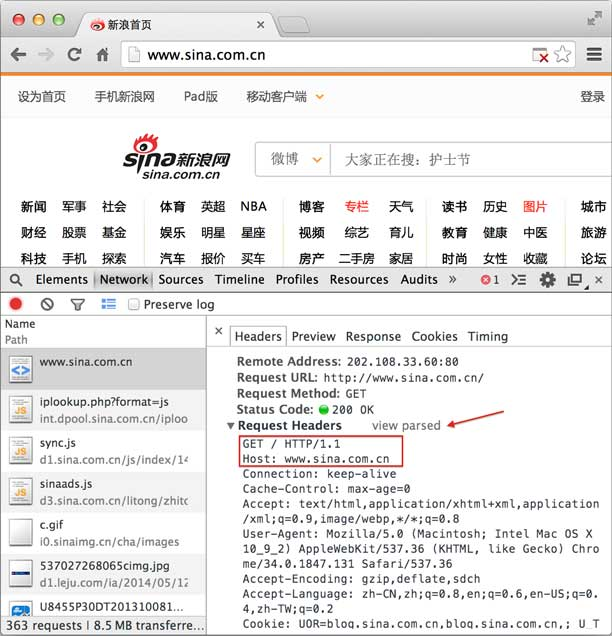``````GET / HTTP/1.1
``````

`GET`表示一个读取请求，将从服务器获得网页数据，`/`表示URL的路径，URL总是以`/`开头，`/`就表示首页，最后的`HTTP/1.1`指示采用的HTTP协议版本是1.1。目前HTTP协议的版本就是1.1，但是大部分服务器也支持1.0版本，主要区别在于1.1版本允许多个HTTP请求复用一个TCP连接，以加快传输速度。

``````Host: www.sina.com.cn
``````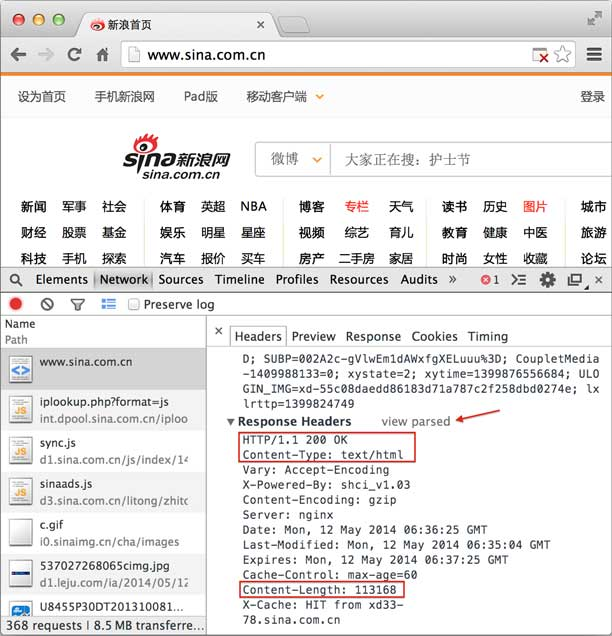HTTP响应分为Header和Body两部分（Body是可选项），我们在`Network`中看到的Header最重要的几行如下：

``````200 OK
``````

`200`表示一个成功的响应，后面的`OK`是说明。失败的响应有`404 Not Found`：网页不存在，`500 Internal Server Error`：服务器内部出错，等等。

``````Content-Type: text/html
``````

`Content-Type`指示响应的内容，这里是`text/html`表示HTML网页。请注意，浏览器就是依靠`Content-Type`来判断响应的内容是网页还是图片，是视频还是音乐。浏览器并不靠URL来判断响应的内容，所以，即使URL是`http://example.com/abc.jpg`，它也不一定就是图片。

HTTP响应的Body就是HTML源码，我们在菜单栏选择“视图”，“开发者”，“查看网页源码”就可以在浏览器中直接查看HTML源码：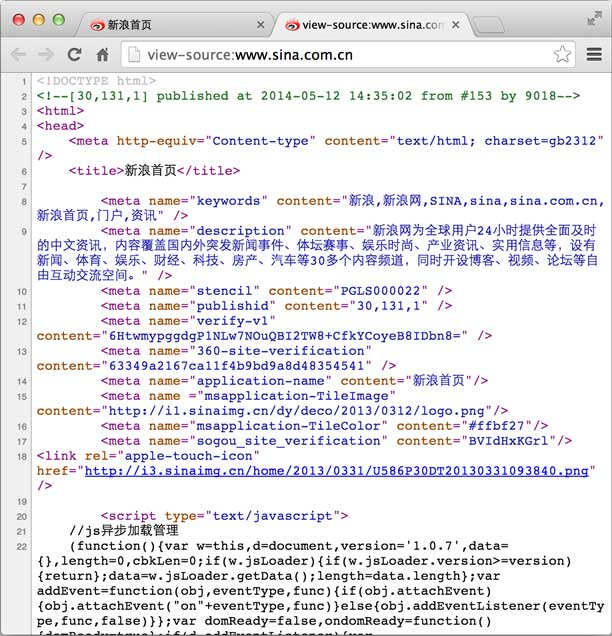#### HTTP请求

Web采用的HTTP协议采用了非常简单的请求-响应模式，从而大大简化了开发。当我们编写一个页面时，我们只需要在HTTP请求中把HTML发送出去，不需要考虑如何附带图片、视频等，浏览器如果需要请求图片和视频，它会发送另一个HTTP请求，因此，一个HTTP请求只处理一个资源。

HTTP协议同时具备极强的扩展性，虽然浏览器请求的是`http://www.sina.com.cn/`的首页，但是新浪在HTML中可以链入其他服务器的资源，比如`<img src="http://i1.sinaimg.cn/home/2013/1008/U8455P30DT20131008135420.png">`，从而将请求压力分散到各个服务器上，并且，一个站点可以链接到其他站点，无数个站点互相链接起来，就形成了World Wide Web，简称WWW。

#### HTTP格式

HTTP协议是一种文本协议，所以，它的格式也非常简单。HTTP GET请求的格式：

``````GET /path HTTP/1.1
``````

HTTP POST请求的格式：

``````POST /path HTTP/1.1

body data goes here...
``````

HTTP响应的格式：

``````200 OK

body data goes here...
``````

HTTP响应如果包含body，也是通过`\r\n\r\n`来分隔的。请再次注意，Body的数据类型由`Content-Type`头来确定，如果是网页，Body就是文本，如果是图片，Body就是图片的二进制数据。

HTTP权威指南

## HTML简介

HTML长什么样？上次我们看了新浪首页的HTML源码，如果仔细数数，竟然有6000多行！

``````<html>
<title>Hello</title>
<body>
<h1>Hello, world!</h1>
</body>
</html>
``````HTML文档就是一系列的Tag组成，最外层的Tag是`<html>`。规范的HTML也包含`<head>...</head>``<body>...</body>`（注意不要和HTTP的Header、Body搞混了），由于HTML是富文档模型，所以，还有一系列的Tag用来表示链接、图片、表格、表单等等。

#### CSS简介

CSS是Cascading Style Sheets（层叠样式表）的简称，CSS用来控制HTML里的所有元素如何展现，比如，给标题元素`<h1>`加一个样式，变成48号字体，灰色，带阴影：

``````<html>
<title>Hello</title>
<style>
h1 {
color: #333333;
font-size: 48px;
}
</style>
<body>
<h1>Hello, world!</h1>
</body>
</html>
``````#### JavaScript简介

JavaScript虽然名称有个Java，但它和Java真的一点关系没有。JavaScript是为了让HTML具有交互性而作为脚本语言添加的，JavaScript既可以内嵌到HTML中，也可以从外部链接到HTML中。如果我们希望当用户点击标题时把标题变成红色，就必须通过JavaScript来实现：

``````<html>
<title>Hello</title>
<style>
h1 {
color: #333333;
font-size: 48px;
}
</style>
<script>
function change() {
document.getElementsByTagName('h1').style.color = '#ff0000';
}
</script>
<body>
<h1 onclick="change()">Hello, world!</h1>
</body>
</html>
``````#### 小结

http://www.w3schools.com/

http://www.w3school.com.cn/

## WSGI接口

1. 浏览器发送一个HTTP请求；

2. 服务器收到请求，生成一个HTML文档；

3. 服务器把HTML文档作为HTTP响应的Body发送给浏览器；

4. 浏览器收到HTTP响应，从HTTP Body取出HTML文档并显示。

WSGI接口定义非常简单，它只要求Web开发者实现一个函数，就可以响应HTTP请求。我们来看一个最简单的Web版本的“Hello, web!”：

``````def application(environ, start_response):
start_response('200 OK', [('Content-Type', 'text/html')])
return [b'<h1>Hello, web!</h1>']
``````

• environ：一个包含所有HTTP请求信息的`dict`对象；

• start_response：一个发送HTTP响应的函数。

`application()`函数中，调用：

``````start_response('200 OK', [('Content-Type', 'text/html')])
``````

#### 运行WSGI服务

``````# hello.py

def application(environ, start_response):
start_response('200 OK', [('Content-Type', 'text/html')])
return [b'<h1>Hello, web!</h1>']
``````

``````# server.py
# 从wsgiref模块导入:
from wsgiref.simple_server import make_server
# 导入我们自己编写的application函数:
from hello import application

# 创建一个服务器，IP地址为空，端口是8000，处理函数是application:
httpd = make_server('', 8000, application)
print('Serving HTTP on port 8000...')
# 开始监听HTTP请求:
httpd.serve_forever()
``````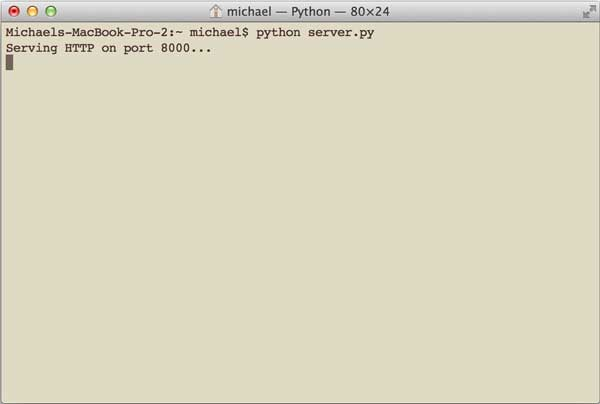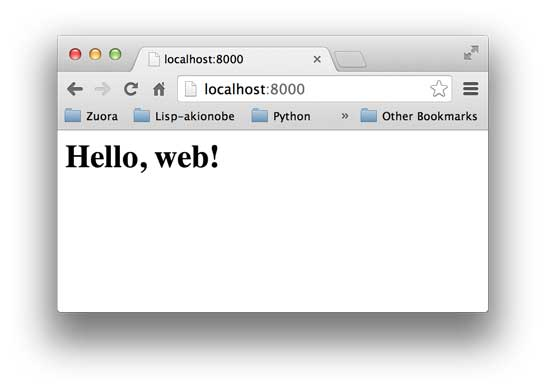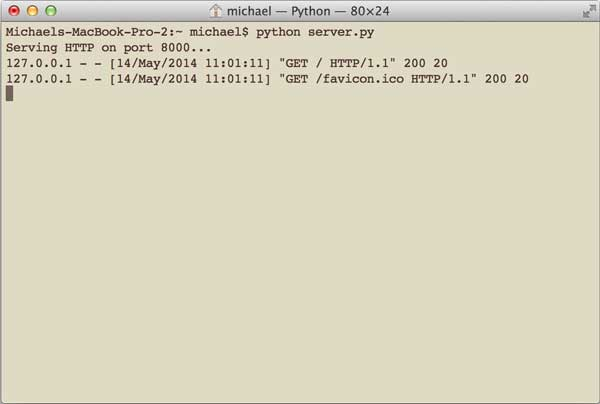`Ctrl+C`终止服务器。

``````# hello.py

def application(environ, start_response):
start_response('200 OK', [('Content-Type', 'text/html')])
body = '<h1>Hello, %s!</h1>' % (environ['PATH_INFO'][1:] or 'web')
return [body.encode('utf-8')]
``````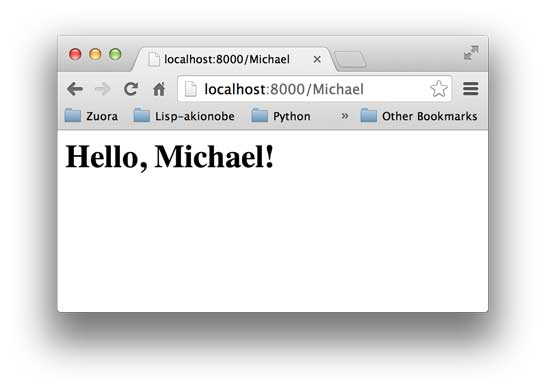hello.py

do_wsgi.py

## 使用Web框架

``````def application(environ, start_response):
method = environ['REQUEST_METHOD']
path = environ['PATH_INFO']
if method=='GET' and path=='/':
return handle_home(environ, start_response)
if method=='POST' and path='/signin':
return handle_signin(environ, start_response)
...
``````

``````\$ pip install flask
``````

• `GET /`：首页，返回`Home`

• `GET /signin`：登录页，显示登录表单；

• `POST /signin`：处理登录表单，显示登录结果。

``````from flask import Flask

@app.route('/', methods=['GET', 'POST'])
def home():
return '<h1>Home</h1>'

@app.route('/signin', methods=['GET'])
def signin_form():
return '''<form action="/signin" method="post">
</form>'''

@app.route('/signin', methods=['POST'])
def signin():
# 需要从request对象读取表单内容：

if __name__ == '__main__':
app.run()
``````

``````\$ python app.py
* Running on http://127.0.0.1:5000/
``````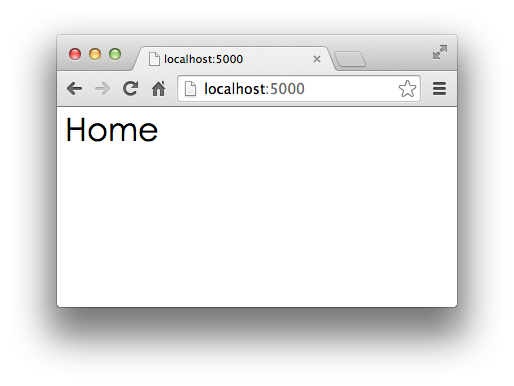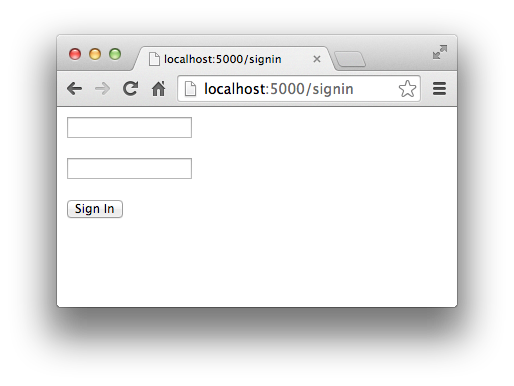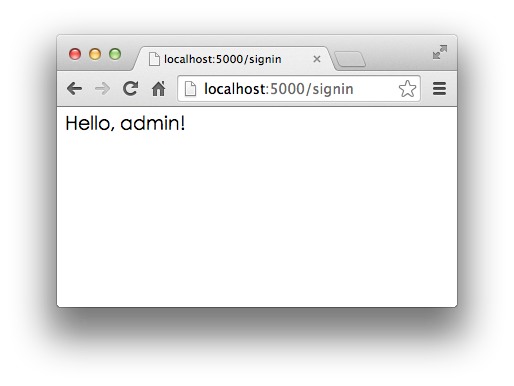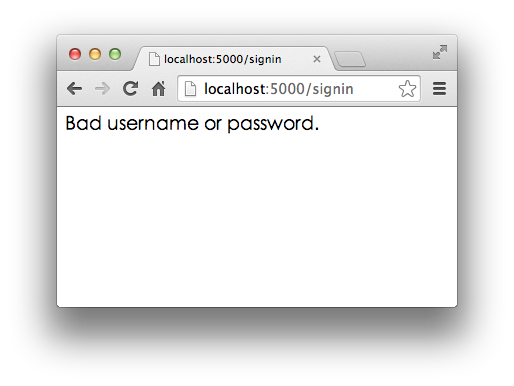• Django：全能型Web框架；

• web.py：一个小巧的Web框架；

## 使用模板

Web框架把我们从WSGI中拯救出来了。现在，我们只需要不断地编写函数，带上URL，就可以继续Web App的开发了。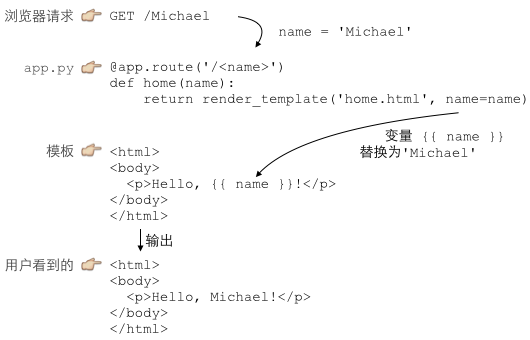Python处理URL的函数就是C：Controller，Controller负责业务逻辑，比如检查用户名是否存在，取出用户信息等等；

MVC中的Model在哪？Model是用来传给View的，这样View在替换变量的时候，就可以从Model中取出相应的数据。

``````{ 'name': 'Michael' }
``````

``````from flask import Flask, request, render_template

@app.route('/', methods=['GET', 'POST'])
def home():
return render_template('home.html')

@app.route('/signin', methods=['GET'])
def signin_form():
return render_template('form.html')

@app.route('/signin', methods=['POST'])
def signin():

if __name__ == '__main__':
app.run()
``````

Flask通过`render_template()`函数来实现模板的渲染。和Web框架类似，Python的模板也有很多种。Flask默认支持的模板是jinja2，所以我们先直接安装jinja2：

``````\$ pip install jinja2
``````

#### home.html

``````<html>
<title>Home</title>
<body>
<h1 style="font-style:italic">Home</h1>
</body>
</html>
``````

#### form.html

``````<html>
<body>
{% if message %}
<p style="color:red">{{ message }}</p>
{% endif %}
<form action="/signin" method="post">
</form>
</body>
</html>
``````

#### signin-ok.html

``````<html>
<body>
</body>
</html>
``````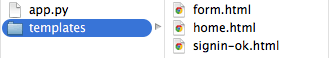``````{% for i in page_list %}
<a href="/page/{{ i }}">{{ i }}</a>
{% endfor %}
``````

• Mako：用`<% ... %>``\${xxx}`的一个模板；

• Cheetah：也是用`<% ... %>``\${xxx}`的一个模板；

• Django：Django是一站式框架，内置一个用`{% ... %}``{{ xxx }}`的模板。

app.py

# 异步IO

``````do_some_code()
f = open('/path/to/file', 'r')
r = f.read() # <== 线程停在此处等待IO操作结果
# IO操作完成后线程才能继续执行:
do_some_code(r)
``````

``````loop = get_event_loop()
while True:
event = loop.get_event()
process_event(event)
``````

## 协程

``````def A():
print('1')
print('2')
print('3')

def B():
print('x')
print('y')
print('z')
``````

``````1
2
x
y
3
z
``````

Python对协程的支持是通过generator实现的。

``````def consumer():
r = ''
while True:
n = yield r
if not n:
return
print('[CONSUMER] Consuming %s...' % n)
r = '200 OK'

def produce(c):
c.send(None)
n = 0
while n < 5:
n = n + 1
print('[PRODUCER] Producing %s...' % n)
r = c.send(n)
print('[PRODUCER] Consumer return: %s' % r)
c.close()

c = consumer()
produce(c)
``````

``````[PRODUCER] Producing 1...
[CONSUMER] Consuming 1...
[PRODUCER] Consumer return: 200 OK
[PRODUCER] Producing 2...
[CONSUMER] Consuming 2...
[PRODUCER] Consumer return: 200 OK
[PRODUCER] Producing 3...
[CONSUMER] Consuming 3...
[PRODUCER] Consumer return: 200 OK
[PRODUCER] Producing 4...
[CONSUMER] Consuming 4...
[PRODUCER] Consumer return: 200 OK
[PRODUCER] Producing 5...
[CONSUMER] Consuming 5...
[PRODUCER] Consumer return: 200 OK
``````

1. 首先调用`c.send(None)`启动生成器；

2. 然后，一旦生产了东西，通过`c.send(n)`切换到`consumer`执行；

3. `consumer`通过`yield`拿到消息，处理，又通过`yield`把结果传回；

4. `produce`拿到`consumer`处理的结果，继续生产下一条消息；

5. `produce`决定不生产了，通过`c.close()`关闭`consumer`，整个过程结束。

“子程序就是协程的一种特例。”

coroutine.py

## asyncio

`asyncio`是Python 3.4版本引入的标准库，直接内置了对异步IO的支持。

`asyncio`的编程模型就是一个消息循环。我们从`asyncio`模块中直接获取一个`EventLoop`的引用，然后把需要执行的协程扔到`EventLoop`中执行，就实现了异步IO。

`asyncio`实现`Hello world`代码如下：

``````import asyncio

@asyncio.coroutine
def hello():
print("Hello world!")
# 异步调用asyncio.sleep(1):
r = yield from asyncio.sleep(1)
print("Hello again!")

# 获取EventLoop:
loop = asyncio.get_event_loop()
# 执行coroutine
loop.run_until_complete(hello())
loop.close()
``````

`@asyncio.coroutine`把一个generator标记为coroutine类型，然后，我们就把这个`coroutine`扔到`EventLoop`中执行。

`hello()`会首先打印出`Hello world!`，然后，`yield from`语法可以让我们方便地调用另一个`generator`。由于`asyncio.sleep()`也是一个`coroutine`，所以线程不会等待`asyncio.sleep()`，而是直接中断并执行下一个消息循环。当`asyncio.sleep()`返回时，线程就可以从`yield from`拿到返回值（此处是`None`），然后接着执行下一行语句。

`asyncio.sleep(1)`看成是一个耗时1秒的IO操作，在此期间，主线程并未等待，而是去执行`EventLoop`中其他可以执行的`coroutine`了，因此可以实现并发执行。

``````import threading
import asyncio

@asyncio.coroutine
def hello():
yield from asyncio.sleep(1)

loop = asyncio.get_event_loop()
loop.close()
``````

``````Hello world! (<_MainThread(MainThread, started 140735195337472)>)
(暂停约1秒)
``````

``````import asyncio

@asyncio.coroutine
def wget(host):
print('wget %s...' % host)
connect = asyncio.open_connection(host, 80)
reader, writer = yield from connect
header = 'GET / HTTP/1.0\r\nHost: %s\r\n\r\n' % host
yield from writer.drain()
while True:
if line == b'\r\n':
break
print('%s header > %s' % (host, line.decode('utf-8').rstrip()))
# Ignore the body, close the socket
writer.close()

loop = asyncio.get_event_loop()
tasks = [wget(host) for host in ['www.sina.com.cn', 'www.sohu.com', 'www.163.com']]
loop.close()
``````

``````wget www.sohu.com...
wget www.sina.com.cn...
wget www.163.com...
(等待一段时间)
www.sohu.com header > HTTP/1.1 200 OK
...
www.sina.com.cn header > HTTP/1.1 200 OK
www.sina.com.cn header > Date: Wed, 20 May 2015 04:56:33 GMT
...
www.163.com header > HTTP/1.0 302 Moved Temporarily
www.163.com header > Server: Cdn Cache Server V2.0
...
``````

#### 小结

`asyncio`提供了完善的异步IO支持；

async_hello.py

async_wget.py

## async/await

`asyncio`提供的`@asyncio.coroutine`可以把一个generator标记为coroutine类型，然后在coroutine内部用`yield from`调用另一个coroutine实现异步操作。

1. `@asyncio.coroutine`替换为`async`
2. `yield from`替换为`await`

``````@asyncio.coroutine
def hello():
print("Hello world!")
r = yield from asyncio.sleep(1)
print("Hello again!")
``````

``````async def hello():
print("Hello world!")
r = await asyncio.sleep(1)
print("Hello again!")
``````

#### 小结

Python从3.5版本开始为`asyncio`提供了`async``await`的新语法；

async_hello2.py

async_wget2.py

## aiohttp

`asyncio`可以实现单线程并发IO操作。如果仅用在客户端，发挥的威力不大。如果把`asyncio`用在服务器端，例如Web服务器，由于HTTP连接就是IO操作，因此可以用单线程+`coroutine`实现多用户的高并发支持。

`asyncio`实现了TCP、UDP、SSL等协议，`aiohttp`则是基于`asyncio`实现的HTTP框架。

``````pip install aiohttp
``````

• `/` - 首页返回`b'<h1>Index</h1>'`

• `/hello/{name}` - 根据URL参数返回文本`hello, %s!`

``````import asyncio

from aiohttp import web

async def index(request):
await asyncio.sleep(0.5)
return web.Response(body=b'<h1>Index</h1>')

async def hello(request):
await asyncio.sleep(0.5)
text = '<h1>hello, %s!</h1>' % request.match_info['name']
return web.Response(body=text.encode('utf-8'))

async def init(loop):
app = web.Application(loop=loop)
srv = await loop.create_server(app.make_handler(), '127.0.0.1', 8000)
print('Server started at http://127.0.0.1:8000...')
return srv

loop = asyncio.get_event_loop()
loop.run_until_complete(init(loop))
loop.run_forever()
``````

aio_web.py

# 实战

#### 目标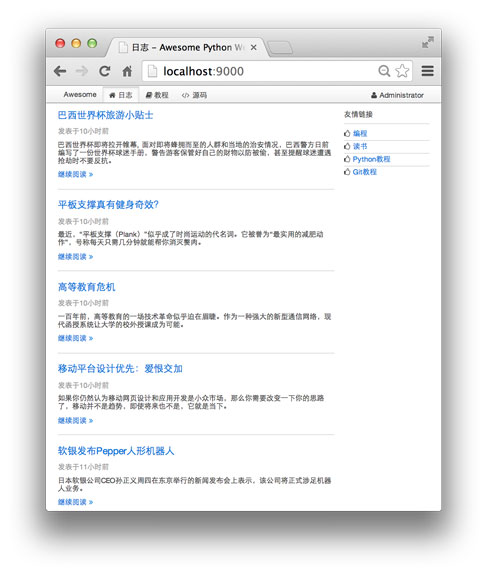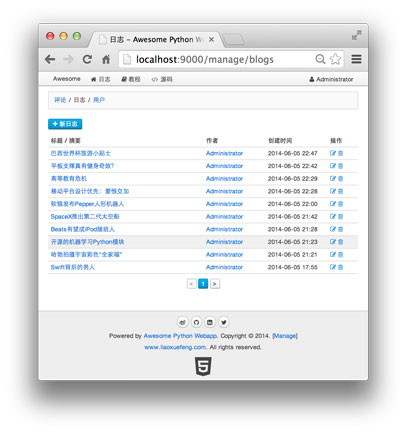#### 项目计划

https://github.com/michaelliao/awesome-python3-webapp/tree/day-01

## Day 1 - 搭建开发环境

#### 搭建开发环境

``````\$ python3 --version
Python 3.4.3
``````

``````\$pip3 install aiohttp
``````

``````\$ pip3 install jinja2
``````

MySQL 5.x数据库，从官方网站下载并安装，安装完毕后，请务必牢记root口令。为避免遗忘口令，建议直接把root口令设置为`password`

MySQL的Python异步驱动程序aiomysql：

``````\$ pip3 install aiomysql
``````

#### 项目结构

``````awesome-python3-webapp/  <-- 根目录
|
+- backup/               <-- 备份目录
|
+- conf/                 <-- 配置文件
|
+- dist/                 <-- 打包目录
|
+- www/                  <-- Web目录，存放.py文件
|  |
|  +- static/            <-- 存放静态文件
|  |
|  +- templates/         <-- 存放模板文件
|
+- ios/                  <-- 存放iOS App工程
|
``````

day-01

## Day 2 - 编写Web App骨架

``````import logging; logging.basicConfig(level=logging.INFO)

import asyncio, os, json, time
from datetime import datetime

from aiohttp import web

def index(request):
return web.Response(body=b'<h1>Awesome</h1>')

@asyncio.coroutine
def init(loop):
app = web.Application(loop=loop)
srv = yield from loop.create_server(app.make_handler(), '127.0.0.1', 9000)
logging.info('server started at http://127.0.0.1:9000...')
return srv

loop = asyncio.get_event_loop()
loop.run_until_complete(init(loop))
loop.run_forever()
``````

``````\$ python3 app.py
INFO:root:server started at http://127.0.0.1:9000...
``````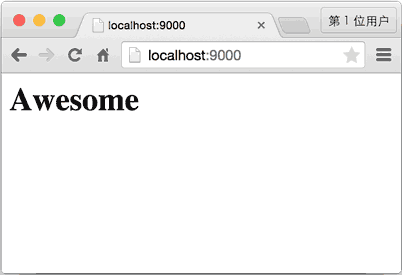day-02

## Day 3 - 编写ORM

Web App里面有很多地方都要访问数据库。访问数据库需要创建数据库连接、游标对象，然后执行SQL语句，最后处理异常，清理资源。这些访问数据库的代码如果分散到各个函数中，势必无法维护，也不利于代码复用。

#### 创建连接池

``````@asyncio.coroutine
def create_pool(loop, **kw):
logging.info('create database connection pool...')
global __pool
__pool = yield from aiomysql.create_pool(
host=kw.get('host', 'localhost'),
port=kw.get('port', 3306),
user=kw['user'],
db=kw['db'],
charset=kw.get('charset', 'utf8'),
autocommit=kw.get('autocommit', True),
maxsize=kw.get('maxsize', 10),
minsize=kw.get('minsize', 1),
loop=loop
)
``````

#### Select

``````@asyncio.coroutine
def select(sql, args, size=None):
log(sql, args)
global __pool
with (yield from __pool) as conn:
cur = yield from conn.cursor(aiomysql.DictCursor)
yield from cur.execute(sql.replace('?', '%s'), args or ())
if size:
rs = yield from cur.fetchmany(size)
else:
rs = yield from cur.fetchall()
yield from cur.close()
logging.info('rows returned: %s' % len(rs))
return rs
``````

SQL语句的占位符是`?`，而MySQL的占位符是`%s``select()`函数在内部自动替换。注意要始终坚持使用带参数的SQL，而不是自己拼接SQL字符串，这样可以防止SQL注入攻击。

#### Insert, Update, Delete

``````@asyncio.coroutine
def execute(sql, args):
log(sql)
with (yield from __pool) as conn:
try:
cur = yield from conn.cursor()
yield from cur.execute(sql.replace('?', '%s'), args)
affected = cur.rowcount
yield from cur.close()
except BaseException as e:
raise
return affected
``````

`execute()`函数和`select()`函数所不同的是，cursor对象不返回结果集，而是通过`rowcount`返回结果数。

#### ORM

``````from orm import Model, StringField, IntegerField

class User(Model):
__table__ = 'users'

id = IntegerField(primary_key=True)
name = StringField()
``````

``````# 创建实例:
user = User(id=123, name='Michael')
# 存入数据库:
user.insert()
# 查询所有User对象:
users = User.findAll()
``````

#### 定义Model

``````class Model(dict, metaclass=ModelMetaclass):

def __init__(self, **kw):
super(Model, self).__init__(**kw)

def __getattr__(self, key):
try:
return self[key]
except KeyError:
raise AttributeError(r"'Model' object has no attribute '%s'" % key)

def __setattr__(self, key, value):
self[key] = value

def getValue(self, key):
return getattr(self, key, None)

def getValueOrDefault(self, key):
value = getattr(self, key, None)
if value is None:
field = self.__mappings__[key]
if field.default is not None:
value = field.default() if callable(field.default) else field.default
logging.debug('using default value for %s: %s' % (key, str(value)))
setattr(self, key, value)
return value
``````

`Model``dict`继承，所以具备所有`dict`的功能，同时又实现了特殊方法`__getattr__()``__setattr__()`，因此又可以像引用普通字段那样写：

``````>>> user['id']
123
>>> user.id
123
``````

``````class Field(object):

def __init__(self, name, column_type, primary_key, default):
self.name = name
self.column_type = column_type
self.primary_key = primary_key
self.default = default

def __str__(self):
return '<%s, %s:%s>' % (self.__class__.__name__, self.column_type, self.name)
``````

``````class StringField(Field):

def __init__(self, name=None, primary_key=False, default=None, ddl='varchar(100)'):
super().__init__(name, ddl, primary_key, default)
``````

``````class ModelMetaclass(type):

def __new__(cls, name, bases, attrs):
# 排除Model类本身:
if name=='Model':
return type.__new__(cls, name, bases, attrs)
# 获取table名称:
tableName = attrs.get('__table__', None) or name
logging.info('found model: %s (table: %s)' % (name, tableName))
# 获取所有的Field和主键名:
mappings = dict()
fields = []
primaryKey = None
for k, v in attrs.items():
if isinstance(v, Field):
logging.info('  found mapping: %s ==> %s' % (k, v))
mappings[k] = v
if v.primary_key:
# 找到主键:
if primaryKey:
raise RuntimeError('Duplicate primary key for field: %s' % k)
primaryKey = k
else:
fields.append(k)
if not primaryKey:
for k in mappings.keys():
attrs.pop(k)
escaped_fields = list(map(lambda f: '`%s`' % f, fields))
attrs['__mappings__'] = mappings # 保存属性和列的映射关系
attrs['__table__'] = tableName
attrs['__primary_key__'] = primaryKey # 主键属性名
attrs['__fields__'] = fields # 除主键外的属性名
# 构造默认的SELECT, INSERT, UPDATE和DELETE语句:
attrs['__select__'] = 'select `%s`, %s from `%s`' % (primaryKey, ', '.join(escaped_fields), tableName)
attrs['__insert__'] = 'insert into `%s` (%s, `%s`) values (%s)' % (tableName, ', '.join(escaped_fields), primaryKey, create_args_string(len(escaped_fields) + 1))
attrs['__update__'] = 'update `%s` set %s where `%s`=?' % (tableName, ', '.join(map(lambda f: '`%s`=?' % (mappings.get(f).name or f), fields)), primaryKey)
attrs['__delete__'] = 'delete from `%s` where `%s`=?' % (tableName, primaryKey)
return type.__new__(cls, name, bases, attrs)
``````

``````class Model(dict):

...

@classmethod
@asyncio.coroutine
def find(cls, pk):
' find object by primary key. '
rs = yield from select('%s where `%s`=?' % (cls.__select__, cls.__primary_key__), [pk], 1)
if len(rs) == 0:
return None
return cls(**rs)
``````

User类现在就可以通过类方法实现主键查找：

``````user = yield from User.find('123')
``````

``````class Model(dict):

...

@asyncio.coroutine
def save(self):
args = list(map(self.getValueOrDefault, self.__fields__))
args.append(self.getValueOrDefault(self.__primary_key__))
rows = yield from execute(self.__insert__, args)
if rows != 1:
logging.warn('failed to insert record: affected rows: %s' % rows)
``````

``````user = User(id=123, name='Michael')
yield from user.save()
``````

• findAll() - 根据WHERE条件查找；

• findNumber() - 根据WHERE条件查找，但返回的是整数，适用于`select count(*)`类型的SQL。

``````user.save()
``````

``````yield from user.save()
``````

day-03

## Day 4 - 编写Model

``````import time, uuid

from orm import Model, StringField, BooleanField, FloatField, TextField

def next_id():
return '%015d%s000' % (int(time.time() * 1000), uuid.uuid4().hex)

class User(Model):
__table__ = 'users'

id = StringField(primary_key=True, default=next_id, ddl='varchar(50)')
email = StringField(ddl='varchar(50)')
passwd = StringField(ddl='varchar(50)')
name = StringField(ddl='varchar(50)')
image = StringField(ddl='varchar(500)')
created_at = FloatField(default=time.time)

class Blog(Model):
__table__ = 'blogs'

id = StringField(primary_key=True, default=next_id, ddl='varchar(50)')
user_id = StringField(ddl='varchar(50)')
user_name = StringField(ddl='varchar(50)')
user_image = StringField(ddl='varchar(500)')
name = StringField(ddl='varchar(50)')
summary = StringField(ddl='varchar(200)')
content = TextField()
created_at = FloatField(default=time.time)

class Comment(Model):

id = StringField(primary_key=True, default=next_id, ddl='varchar(50)')
blog_id = StringField(ddl='varchar(50)')
user_id = StringField(ddl='varchar(50)')
user_name = StringField(ddl='varchar(50)')
user_image = StringField(ddl='varchar(500)')
content = TextField()
created_at = FloatField(default=time.time)
``````

#### 初始化数据库表

``````-- schema.sql

drop database if exists awesome;

create database awesome;

use awesome;

grant select, insert, update, delete on awesome.* to 'www-data'@'localhost' identified by 'www-data';

create table users (
`id` varchar(50) not null,
`email` varchar(50) not null,
`passwd` varchar(50) not null,
`name` varchar(50) not null,
`image` varchar(500) not null,
`created_at` real not null,
unique key `idx_email` (`email`),
key `idx_created_at` (`created_at`),
primary key (`id`)
) engine=innodb default charset=utf8;

create table blogs (
`id` varchar(50) not null,
`user_id` varchar(50) not null,
`user_name` varchar(50) not null,
`user_image` varchar(500) not null,
`name` varchar(50) not null,
`summary` varchar(200) not null,
`content` mediumtext not null,
`created_at` real not null,
key `idx_created_at` (`created_at`),
primary key (`id`)
) engine=innodb default charset=utf8;

`id` varchar(50) not null,
`blog_id` varchar(50) not null,
`user_id` varchar(50) not null,
`user_name` varchar(50) not null,
`user_image` varchar(500) not null,
`content` mediumtext not null,
`created_at` real not null,
key `idx_created_at` (`created_at`),
primary key (`id`)
) engine=innodb default charset=utf8;
``````

``````\$ mysql -u root -p < schema.sql
``````

#### 编写数据访问代码

``````import orm
from models import User, Blog, Comment

def test():

u = User(name='Test', email='test@example.com', passwd='1234567890', image='about:blank')

yield from u.save()

for x in test():
pass
``````

day-04

## Day 5 - 编写Web框架

`aiohttp`已经是一个Web框架了，为什么我们还需要自己封装一个？

``````@asyncio.coroutine
def handle_url_xxx(request):
pass
``````

``````url_param = request.match_info['key']
query_params = parse_qs(request.query_string)
``````

``````text = render('template', data)
return web.Response(text.encode('utf-8'))
``````

``````@get('/blog/{id}')
def get_blog(id):
pass
``````

``````@get('/api/comments')
pass
``````

``````return {
'__template__': 'index.html',
'data': '...'
}
``````

#### @get和@post

``````def get(path):
'''
Define decorator @get('/path')
'''
def decorator(func):
@functools.wraps(func)
def wrapper(*args, **kw):
return func(*args, **kw)
wrapper.__method__ = 'GET'
wrapper.__route__ = path
return wrapper
return decorator
``````

`@post``@get`定义类似。

#### 定义RequestHandler

URL处理函数不一定是一个`coroutine`，因此我们用`RequestHandler()`来封装一个URL处理函数。

`RequestHandler`是一个类，由于定义了`__call__()`方法，因此可以将其实例视为函数。

`RequestHandler`目的就是从URL函数中分析其需要接收的参数，从`request`中获取必要的参数，调用URL函数，然后把结果转换为`web.Response`对象，这样，就完全符合`aiohttp`框架的要求：

``````class RequestHandler(object):

def __init__(self, app, fn):
self._app = app
self._func = fn
...

@asyncio.coroutine
def __call__(self, request):
kw = ... 获取参数
r = yield from self._func(**kw)
return r
``````

``````def add_route(app, fn):
method = getattr(fn, '__method__', None)
path = getattr(fn, '__route__', None)
if path is None or method is None:
raise ValueError('@get or @post not defined in %s.' % str(fn))
if not asyncio.iscoroutinefunction(fn) and not inspect.isgeneratorfunction(fn):
fn = asyncio.coroutine(fn)
logging.info('add route %s %s => %s(%s)' % (method, path, fn.__name__, ', '.join(inspect.signature(fn).parameters.keys())))
``````

``````add_route(app, handles.index)
...
``````

``````# 自动把handler模块的所有符合条件的函数注册了:
``````

`add_routes()`定义如下：

``````def add_routes(app, module_name):
n = module_name.rfind('.')
if n == (-1):
mod = __import__(module_name, globals(), locals())
else:
name = module_name[n+1:]
mod = getattr(__import__(module_name[:n], globals(), locals(), [name]), name)
for attr in dir(mod):
if attr.startswith('_'):
continue
fn = getattr(mod, attr)
if callable(fn):
method = getattr(fn, '__method__', None)
path = getattr(fn, '__route__', None)
if method and path:
``````

``````app = web.Application(loop=loop, middlewares=[
logger_factory, response_factory
])
init_jinja2(app, filters=dict(datetime=datetime_filter))
``````

#### middleware

`middleware`是一种拦截器，一个URL在被某个函数处理前，可以经过一系列的`middleware`的处理。

``````@asyncio.coroutine
def logger_factory(app, handler):
@asyncio.coroutine
def logger(request):
# 记录日志:
logging.info('Request: %s %s' % (request.method, request.path))
# 继续处理请求:
return (yield from handler(request))
return logger
``````

`response`这个`middleware`把返回值转换为`web.Response`对象再返回，以保证满足`aiohttp`的要求：

``````@asyncio.coroutine
def response_factory(app, handler):
@asyncio.coroutine
def response(request):
# 结果:
r = yield from handler(request)
if isinstance(r, web.StreamResponse):
return r
if isinstance(r, bytes):
resp = web.Response(body=r)
resp.content_type = 'application/octet-stream'
return resp
if isinstance(r, str):
resp = web.Response(body=r.encode('utf-8'))
resp.content_type = 'text/html;charset=utf-8'
return resp
if isinstance(r, dict):
...
``````

day-05

## Day 6 - 编写配置文件

``````# config_default.py

configs = {
'db': {
'host': '127.0.0.1',
'port': 3306,
'user': 'www-data',
'database': 'awesome'
},
'session': {
'secret': 'AwEsOmE'
}
}
``````

``````# config_override.py

configs = {
'db': {
'host': '192.168.0.100'
}
}
``````

`config_default.py`作为开发环境的标准配置，把`config_override.py`作为生产环境的标准配置，我们就可以既方便地在本地开发，又可以随时把应用部署到服务器上。

``````# config.py
configs = config_default.configs

try:
import config_override
configs = merge(configs, config_override.configs)
except ImportError:
pass
``````

day-06

## Day 7 - 编写MVC

``````@get('/')
def index(request):
users = yield from User.findAll()
return {
'__template__': 'test.html',
'users': users
}
``````

`'__template__'`指定的模板文件是`test.html`，其他参数是传递给模板的数据，所以我们在模板的根目录`templates`下创建`test.html`

``````<!DOCTYPE html>
<html>
<meta charset="utf-8" />
<title>Test users - Awesome Python Webapp</title>
<body>
<h1>All users</h1>
{% for u in users %}
<p>{{ u.name }} / {{ u.email }}</p>
{% endfor %}
</body>
</html>
``````

``````\$ python3 app.py
``````day-07

## Day 8 - 构建前端

``````static/
+- css/
|  +- awesome.css
|  +- uikit.min.css
+- fonts/
|  +- fontawesome-webfont.eot
|  +- fontawesome-webfont.ttf
|  +- fontawesome-webfont.woff
|  +- FontAwesome.otf
+- js/
+- awesome.js
+- html5.js
+- jquery.min.js
+- uikit.min.js
``````

``````<html>
<% include file="index_body.html" %>
<% include file="inc_footer.html" %>
</html>
``````

“继承”模板的方式是通过编写一个“父模板”，在父模板中定义一些可替换的block（块）。然后，编写多个“子模板”，每个子模板都可以只替换父模板定义的block。比如，定义一个最简单的父模板：

``````<!-- base.html -->
<html>
<title>{% block title%} 这里定义了一个名为title的block {% endblock %}</title>
<body>
{% block content %} 这里定义了一个名为content的block {% endblock %}
</body>
</html>
``````

``````{% extends 'base.html' %}

{% block title %} A {% endblock %}

{% block content %}
<h1>Chapter A</h1>
<p>blablabla...</p>
{% endblock %}
``````

``````{% extends 'base.html' %}

{% block title %} B {% endblock %}

{% block content %}
<h1>Chapter B</h1>
<ul>
<li>list 1</li>
<li>list 2</li>
</ul>
{% endblock %}
``````

``````<!DOCTYPE html>
<html>
<meta charset="utf-8" />
{% block meta %}<!-- block meta  -->{% endblock %}
<title>{% block title %} ? {% endblock %} - Awesome Python Webapp</title>
<script src="/static/js/jquery.min.js"></script>
<script src="/static/js/md5.js"></script>
<script src="/static/js/uikit.min.js"></script>
<script src="/static/js/awesome.js"></script>
<body>
<nav class="uk-navbar uk-navbar-attached uk-margin-bottom">
<div class="uk-container uk-container-center">
<a href="/" class="uk-navbar-brand">Awesome</a>
<ul class="uk-navbar-nav">
<li data-url="blogs"><a href="/"><i class="uk-icon-home"></i> 日志</a></li>
<li><a target="_blank" href="#"><i class="uk-icon-book"></i> 教程</a></li>
<li><a target="_blank" href="#"><i class="uk-icon-code"></i> 源码</a></li>
</ul>
<div class="uk-navbar-flip">
<ul class="uk-navbar-nav">
{% if user %}
<li class="uk-parent" data-uk-dropdown>
<a href="#0"><i class="uk-icon-user"></i> {{ user.name }}</a>
<div class="uk-dropdown uk-dropdown-navbar">
<ul class="uk-nav uk-nav-navbar">
<li><a href="/signout"><i class="uk-icon-sign-out"></i> 登出</a></li>
</ul>
</div>
</li>
{% else %}
<li><a href="/signin"><i class="uk-icon-sign-in"></i> 登陆</a></li>
<li><a href="/register"><i class="uk-icon-edit"></i> 注册</a></li>
{% endif %}
</ul>
</div>
</div>
</nav>

<div class="uk-container uk-container-center">
<div class="uk-grid">
<!-- content -->
{% block content %}
{% endblock %}
<!-- // content -->
</div>
</div>

<div class="uk-margin-large-top" style="background-color:#eee; border-top:1px solid #ccc;">
<div class="uk-container uk-container-center uk-text-center">
<div class="uk-panel uk-margin-top uk-margin-bottom">
<p>
<a target="_blank" href="#" class="uk-icon-button uk-icon-weibo"></a>
<a target="_blank" href="#" class="uk-icon-button uk-icon-github"></a>
</p>
<a target="_blank" href="#"><i class="uk-icon-html5" style="font-size:64px; color: #444;"></i></a>
</div>
</div>
</div>
</body>
</html>
``````

`__base__.html`定义的几个block作用如下：

``````{% block meta %} ... {% endblock %}
``````

``````{% block title %} ... {% endblock %}
``````

``````{% block beforehead %} ... {% endblock %}
``````

``````{% block content %}
...
{% endblock %}
``````

``````{% extends '__base__.html' %}

{% block title %}日志{% endblock %}

{% block content %}

<div class="uk-width-medium-3-4">
<article class="uk-article">
<h2><a href="/blog/{{ blog.id }}">{{ blog.name }}</a></h2>
<p class="uk-article-meta">发表于{{ blog.created_at}}</p>
<p>{{ blog.summary }}</p>
<p><a href="/blog/{{ blog.id }}">继续阅读 <i class="uk-icon-angle-double-right"></i></a></p>
</article>
<hr class="uk-article-divider">
{% endfor %}
</div>

<div class="uk-width-medium-1-4">
<h3 class="uk-panel-title">友情链接</h3>
<ul class="uk-list uk-list-line">
<li><i class="uk-icon-thumbs-o-up"></i> <a target="_blank" href="#">编程</a></li>
<li><i class="uk-icon-thumbs-o-up"></i> <a target="_blank" href="#">读书</a></li>
<li><i class="uk-icon-thumbs-o-up"></i> <a target="_blank" href="#">Python教程</a></li>
<li><i class="uk-icon-thumbs-o-up"></i> <a target="_blank" href="#">Git教程</a></li>
</ul>
</div>
</div>

{% endblock %}
``````

``````@get('/')
def index(request):
summary = 'Lorem ipsum dolor sit amet, consectetur adipisicing elit, sed do eiusmod tempor incididunt ut labore et dolore magna aliqua.'
blogs = [
Blog(id='1', name='Test Blog', summary=summary, created_at=time.time()-120),
Blog(id='2', name='Something New', summary=summary, created_at=time.time()-3600),
Blog(id='3', name='Learn Swift', summary=summary, created_at=time.time()-7200)
]
return {
'__template__': 'blogs.html',
'blogs': blogs
}
``````

Blog的创建日期显示的是一个浮点数，因为它是由这段模板渲染出来的：

``````<p class="uk-article-meta">发表于{{ blog.created_at }}</p>
``````

``````<p class="uk-article-meta">发表于{{ blog.created_at|datetime }}</p>
``````

filter需要在初始化jinja2时设置。相关代码如下：

``````def datetime_filter(t):
delta = int(time.time() - t)
if delta < 60:
return '1分钟前'
if delta < 3600:
return '%s分钟前' % (delta // 60)
if delta < 86400:
return '%s小时前' % (delta // 3600)
if delta < 604800:
return '%s天前' % (delta // 86400)
dt = datetime.fromtimestamp(t)
return '%s年%s月%s日' % (dt.year, dt.month, dt.day)

...
init_jinja2(app, filters=dict(datetime=datetime_filter))
...
``````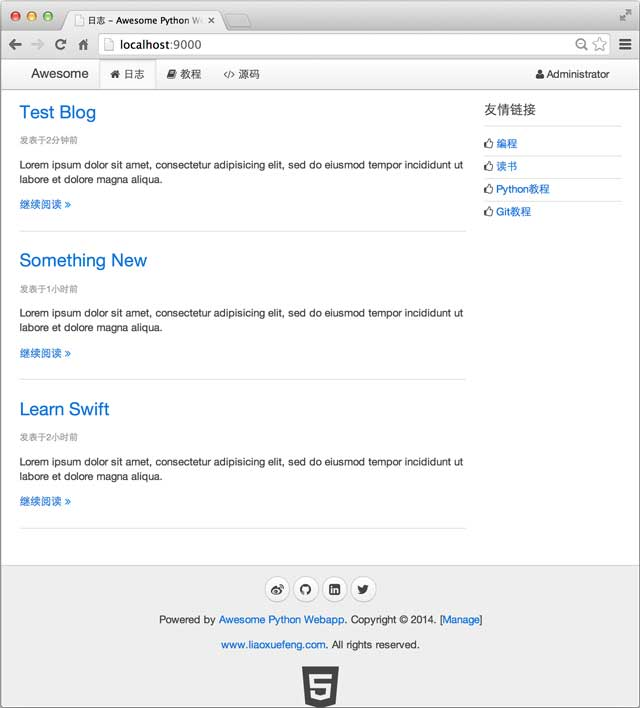day-08

## Day 9 - 编写API

REST就是一种设计API的模式。最常用的数据格式是JSON。由于JSON能直接被JavaScript读取，所以，以JSON格式编写的REST风格的API具有简单、易读、易用的特点。

``````@get('/api/users')
def api_get_users(*, page='1'):
page_index = get_page_index(page)
num = yield from User.findNumber('count(id)')
p = Page(num, page_index)
if num == 0:
return dict(page=p, users=())
users = yield from User.findAll(orderBy='created_at desc', limit=(p.offset, p.limit))
for u in users:
u.passwd = '******'
return dict(page=p, users=users)
``````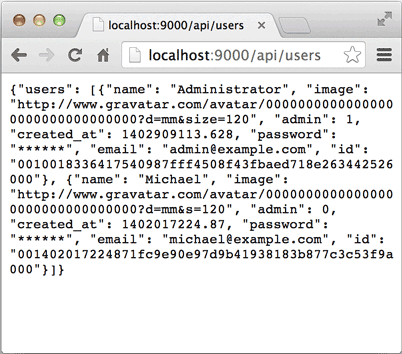day-09

## Day 10 - 用户注册和登录

``````_RE_EMAIL = re.compile(r'^[a-z0-9\.\-\_]+\@[a-z0-9\-\_]+(\.[a-z0-9\-\_]+){1,4}\$')
_RE_SHA1 = re.compile(r'^[0-9a-f]{40}\$')

@post('/api/users')
def api_register_user(*, email, name, passwd):
if not name or not name.strip():
raise APIValueError('name')
if not email or not _RE_EMAIL.match(email):
raise APIValueError('email')
if not passwd or not _RE_SHA1.match(passwd):
raise APIValueError('passwd')
users = yield from User.findAll('email=?', [email])
if len(users) > 0:
raise APIError('register:failed', 'email', 'Email is already in use.')
uid = next_id()
sha1_passwd = '%s:%s' % (uid, passwd)
user = User(id=uid, name=name.strip(), email=email, passwd=hashlib.sha1(sha1_passwd.encode('utf-8')).hexdigest(), image='http://www.gravatar.com/avatar/%s?d=mm&s=120' % hashlib.md5(email.encode('utf-8')).hexdigest())
yield from user.save()
r = web.Response()
user.passwd = '******'
r.content_type = 'application/json'
r.body = json.dumps(user, ensure_ascii=False).encode('utf-8')
return r
``````

``````{% extends '__base__.html' %}

{% block title %}注册{% endblock %}

<script>
function validateEmail(email) {
var re = /^[a-z0-9\.\-\_]+\@[a-z0-9\-\_]+(\.[a-z0-9\-\_]+){1,4}\$/;
return re.test(email.toLowerCase());
}
\$(function () {
var vm = new Vue({
el: '#vm',
data: {
name: '',
email: '',
},
methods: {
submit: function (event) {
event.preventDefault();
var \$form = \$('#vm');
if (! this.name.trim()) {
return \$form.showFormError('请输入名字');
}
if (! validateEmail(this.email.trim().toLowerCase())) {
return \$form.showFormError('请输入正确的Email地址');
}
return \$form.showFormError('口令长度至少为6个字符');
}
return \$form.showFormError('两次输入的口令不一致');
}
var email = this.email.trim().toLowerCase();
\$form.postJSON('/api/users', {
name: this.name.trim(),
email: email,
passwd: CryptoJS.SHA1(email + ':' + this.password1).toString()
}, function (err, r) {
if (err) {
return \$form.showFormError(err);
}
return location.assign('/');
});
}
}
});
\$('#vm').show();
});
</script>

{% endblock %}

{% block content %}

<div class="uk-width-2-3">
<h1>欢迎注册！</h1>
<form id="vm" v-on="submit: submit" class="uk-form uk-form-stacked">
<div class="uk-form-row">
<label class="uk-form-label">名字:</label>
<div class="uk-form-controls">
<input v-model="name" type="text" maxlength="50" placeholder="名字" class="uk-width-1-1">
</div>
</div>
<div class="uk-form-row">
<label class="uk-form-label">电子邮件:</label>
<div class="uk-form-controls">
<input v-model="email" type="text" maxlength="50" placeholder="your-name@example.com" class="uk-width-1-1">
</div>
</div>
<div class="uk-form-row">
<label class="uk-form-label">输入口令:</label>
<div class="uk-form-controls">
</div>
</div>
<div class="uk-form-row">
<label class="uk-form-label">重复口令:</label>
<div class="uk-form-controls">
</div>
</div>
<div class="uk-form-row">
<button type="submit" class="uk-button uk-button-primary"><i class="uk-icon-user"></i> 注册</button>
</div>
</form>
</div>

{% endblock %}
``````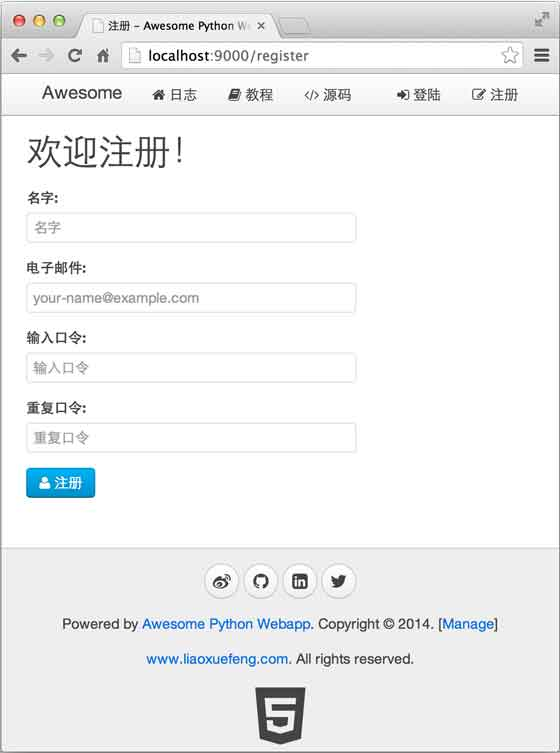Session的优点是简单易用，可以直接从Session中取出用户登录信息。

Session的缺点是服务器需要在内存中维护一个映射表来存储用户登录信息，如果有两台以上服务器，就需要对Session做集群，因此，使用Session的Web App很难扩展。

``````"用户id" + "过期时间" + SHA1("用户id" + "用户口令" + "过期时间" + "SecretKey")
``````

• 用户id

• 过期时间

• SHA1值

``````SHA1("用户id" + "用户口令" + "过期时间" + "SecretKey")
``````

``````@post('/api/authenticate')
def authenticate(*, email, passwd):
if not email:
raise APIValueError('email', 'Invalid email.')
if not passwd:
users = yield from User.findAll('email=?', [email])
if len(users) == 0:
raise APIValueError('email', 'Email not exist.')
user = users
# check passwd:
sha1 = hashlib.sha1()
sha1.update(user.id.encode('utf-8'))
sha1.update(b':')
sha1.update(passwd.encode('utf-8'))
if user.passwd != sha1.hexdigest():
r = web.Response()
user.passwd = '******'
r.content_type = 'application/json'
r.body = json.dumps(user, ensure_ascii=False).encode('utf-8')
return r

# build cookie string by: id-expires-sha1
expires = str(int(time.time() + max_age))
s = '%s-%s-%s-%s' % (user.id, user.passwd, expires, _COOKIE_KEY)
L = [user.id, expires, hashlib.sha1(s.encode('utf-8')).hexdigest()]
return '-'.join(L)
``````

``````@asyncio.coroutine
def auth_factory(app, handler):
@asyncio.coroutine
def auth(request):
logging.info('check user: %s %s' % (request.method, request.path))
request.__user__ = None
if user:
logging.info('set current user: %s' % user.email)
request.__user__ = user
return (yield from handler(request))
return auth

@asyncio.coroutine
'''
'''
return None
try:
if len(L) != 3:
return None
uid, expires, sha1 = L
if int(expires) < time.time():
return None
user = yield from User.find(uid)
if user is None:
return None
s = '%s-%s-%s-%s' % (uid, user.passwd, expires, _COOKIE_KEY)
if sha1 != hashlib.sha1(s.encode('utf-8')).hexdigest():
logging.info('invalid sha1')
return None
user.passwd = '******'
return user
except Exception as e:
logging.exception(e)
return None
``````

day-10

## Day 11 - 编写日志创建页

``````@post('/api/blogs')
def api_create_blog(request, *, name, summary, content):
if not name or not name.strip():
raise APIValueError('name', 'name cannot be empty.')
if not summary or not summary.strip():
raise APIValueError('summary', 'summary cannot be empty.')
if not content or not content.strip():
raise APIValueError('content', 'content cannot be empty.')
blog = Blog(user_id=request.__user__.id, user_name=request.__user__.name, user_image=request.__user__.image, name=name.strip(), summary=summary.strip(), content=content.strip())
yield from blog.save()
return blog
``````

Web开发真正困难的地方在于编写前端页面。前端页面需要混合HTML、CSS和JavaScript，如果对这三者没有深入地掌握，编写的前端页面将很快难以维护。

``````s = '<html><head><title>'
+ title
+ body
+ '</body></html>'
``````

``````<html>
<title>{{ title }}</title>
<body>
{{ body }}
</body>
</html>
``````

ASP、JSP、PHP等都是用这种模板方式生成前端页面。

MVVM最早由微软提出来，它借鉴了桌面应用程序的MVC思想，在前端页面中，把Model用纯JavaScript对象表示：

``````<script>
var blog = {
name: 'hello',
summary: 'this is summary',
content: 'this is content...'
};
</script>
``````

View是纯HTML：

``````<form action="/api/blogs" method="post">
<input name="name">
<input name="summary">
<textarea name="content"></textarea>
<button type="submit">OK</button>
</form>
``````

ViewModel如何编写？需要用JavaScript编写一个通用的ViewModel，这样，就可以复用整个MVVM模型了。

``````{% extends '__base__.html' %}

{% block title %}编辑日志{% endblock %}

<script>
var
ID = '{{ id }}',
action = '{{ action }}';
function initVM(blog) {
var vm = new Vue({
el: '#vm',
data: blog,
methods: {
submit: function (event) {
event.preventDefault();
var \$form = \$('#vm').find('form');
\$form.postJSON(action, this.\$data, function (err, r) {
if (err) {
\$form.showFormError(err);
}
else {
return location.assign('/api/blogs/' + r.id);
}
});
}
}
});
\$('#vm').show();
}
\$(function () {
if (ID) {
getJSON('/api/blogs/' + ID, function (err, blog) {
if (err) {
return fatal(err);
}
initVM(blog);
});
}
else {
initVM({
name: '',
summary: '',
content: ''
});
}
});
</script>

{% endblock %}

{% block content %}

<div class="uk-width-1-1 uk-margin-bottom">
<div class="uk-panel uk-panel-box">
<li><a href="/manage/blogs">日志</a></li>
<li><a href="/manage/users">用户</a></li>
</ul>
</div>
</div>

<div id="error" class="uk-width-1-1">
</div>

<span><i class="uk-icon-spinner uk-icon-medium uk-icon-spin"></i> 正在加载...</span>
</div>

<div id="vm" class="uk-width-2-3">
<form v-on="submit: submit" class="uk-form uk-form-stacked">
<div class="uk-form-row">
<label class="uk-form-label">标题:</label>
<div class="uk-form-controls">
<input v-model="name" name="name" type="text" placeholder="标题" class="uk-width-1-1">
</div>
</div>
<div class="uk-form-row">
<label class="uk-form-label">摘要:</label>
<div class="uk-form-controls">
<textarea v-model="summary" rows="4" name="summary" placeholder="摘要" class="uk-width-1-1" style="resize:none;"></textarea>
</div>
</div>
<div class="uk-form-row">
<label class="uk-form-label">内容:</label>
<div class="uk-form-controls">
<textarea v-model="content" rows="16" name="content" placeholder="内容" class="uk-width-1-1" style="resize:none;"></textarea>
</div>
</div>
<div class="uk-form-row">
<button type="submit" class="uk-button uk-button-primary"><i class="uk-icon-save"></i> 保存</button>
<a href="/manage/blogs" class="uk-button"><i class="uk-icon-times"></i> 取消</a>
</div>
</form>
</div>

{% endblock %}
``````

el：根据选择器查找绑定的View，这里是`#vm`，就是id为`vm`的DOM，对应的是一个`<div>`标签；

data：JavaScript对象表示的Model，我们初始化为`{ name: '', summary: '', content: ''}`

methods：View可以触发的JavaScript函数，`submit`就是提交表单时触发的函数。

``````<!-- input的value和Model的name关联起来了 -->
<input v-model="name" class="uk-width-1-1">
``````

Form表单通过`<form v-on="submit: submit">`把提交表单的事件关联到`submit`方法。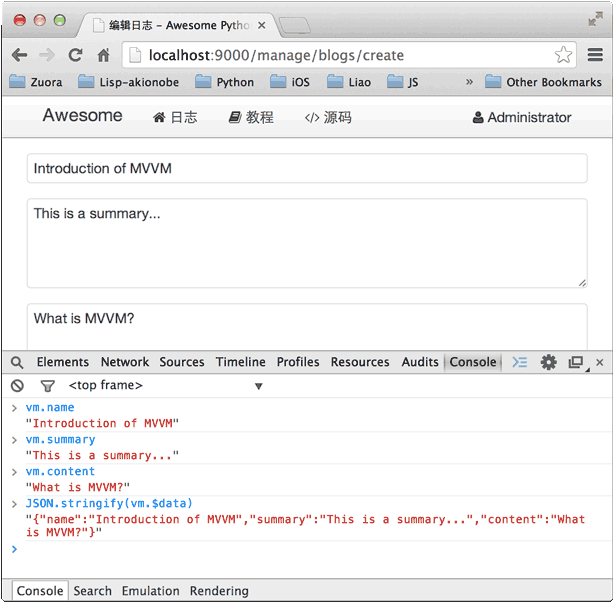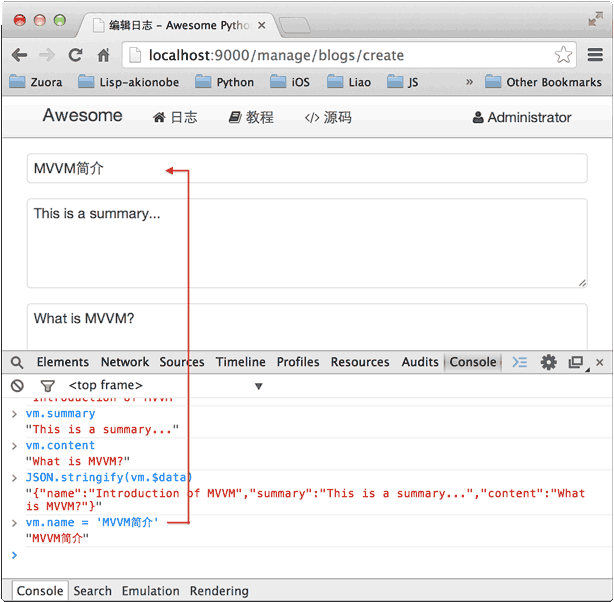day-11

## Day 12 - 编写日志列表页

MVVM模式不但可用于Form表单，在复杂的管理页面中也能大显身手。例如，分页显示Blog的功能，我们先把后端代码写出来：

`apis.py`中定义一个`Page`类用于存储分页信息：

``````class Page(object):

def __init__(self, item_count, page_index=1, page_size=10):
self.item_count = item_count
self.page_size = page_size
self.page_count = item_count // page_size + (1 if item_count % page_size > 0 else 0)
if (item_count == 0) or (page_index > self.page_count):
self.offset = 0
self.limit = 0
self.page_index = 1
else:
self.page_index = page_index
self.offset = self.page_size * (page_index - 1)
self.limit = self.page_size
self.has_next = self.page_index < self.page_count
self.has_previous = self.page_index > 1

def __str__(self):
return 'item_count: %s, page_count: %s, page_index: %s, page_size: %s, offset: %s, limit: %s' % (self.item_count, self.page_count, self.page_index, self.page_size, self.offset, self.limit)

__repr__ = __str__
``````

`handlers.py`中实现API：

``````@get('/api/blogs')
def api_blogs(*, page='1'):
page_index = get_page_index(page)
num = yield from Blog.findNumber('count(id)')
p = Page(num, page_index)
if num == 0:
return dict(page=p, blogs=())
blogs = yield from Blog.findAll(orderBy='created_at desc', limit=(p.offset, p.limit))
return dict(page=p, blogs=blogs)
``````

``````@get('/manage/blogs')
def manage_blogs(*, page='1'):
return {
'__template__': 'manage_blogs.html',
'page_index': get_page_index(page)
}
``````

``````{
"page": {
"has_next": true,
"page_index": 1,
"page_count": 2,
"has_previous": false,
"item_count": 12
},
"blogs": [...]
}
``````

``````<script>
function initVM(data) {
var vm = new Vue({
el: '#vm',
data: {
blogs: data.blogs,
page: data.page
},
methods: {
edit_blog: function (blog) {
location.assign('/manage/blogs/edit?id=' + blog.id);
},
delete_blog: function (blog) {
if (confirm('确认要删除“' + blog.name + '”？删除后不可恢复！')) {
postJSON('/api/blogs/' + blog.id + '/delete', function (err, r) {
if (err) {
return alert(err.message || err.error || err);
}
refresh();
});
}
}
}
});
\$('#vm').show();
}
\$(function() {
getJSON('/api/blogs', {
page: {{ page_index }}
}, function (err, results) {
if (err) {
return fatal(err);
}
initVM(results);
});
});
</script>
``````

View的容器是`#vm`，包含一个table，我们用`v-repeat`可以把Model的数组`blogs`直接变成多行的`<tr>`

``````<div id="vm" class="uk-width-1-1">
<a href="/manage/blogs/create" class="uk-button uk-button-primary"><i class="uk-icon-plus"></i> 新日志</a>

<table class="uk-table uk-table-hover">
<tr>
<th class="uk-width-5-10">标题 / 摘要</th>
<th class="uk-width-2-10">作者</th>
<th class="uk-width-2-10">创建时间</th>
<th class="uk-width-1-10">操作</th>
</tr>
<tbody>
<tr v-repeat="blog: blogs" >
<td>
<a target="_blank" v-attr="href: '/blog/'+blog.id" v-text="blog.name"></a>
</td>
<td>
<a target="_blank" v-attr="href: '/user/'+blog.user_id" v-text="blog.user_name"></a>
</td>
<td>
<span v-text="blog.created_at.toDateTime()"></span>
</td>
<td>
<a href="#0" v-on="click: edit_blog(blog)"><i class="uk-icon-edit"></i>
<a href="#0" v-on="click: delete_blog(blog)"><i class="uk-icon-trash-o"></i>
</td>
</tr>
</tbody>
</table>

<div v-component="pagination" v-with="page"></div>
</div>
``````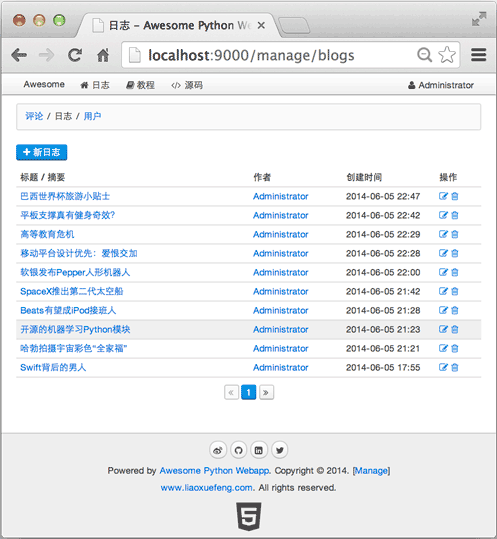day-12

## Day 13 - 提升开发效率

Django的开发环境在Debug模式下就可以做到自动重新加载，如果我们编写的服务器也能实现这个功能，就能大大提升开发效率。

``````\$ pip3 install watchdog
``````

``````#!/usr/bin/env python3
# -*- coding: utf-8 -*-

__author__ = 'Michael Liao'

import os, sys, time, subprocess

from watchdog.observers import Observer
from watchdog.events import FileSystemEventHandler

def log(s):
print('[Monitor] %s' % s)

class MyFileSystemEventHander(FileSystemEventHandler):

def __init__(self, fn):
super(MyFileSystemEventHander, self).__init__()
self.restart = fn

def on_any_event(self, event):
if event.src_path.endswith('.py'):
log('Python source file changed: %s' % event.src_path)
self.restart()

command = ['echo', 'ok']
process = None

def kill_process():
global process
if process:
log('Kill process [%s]...' % process.pid)
process.kill()
process.wait()
log('Process ended with code %s.' % process.returncode)
process = None

def start_process():
global process, command
log('Start process %s...' % ' '.join(command))
process = subprocess.Popen(command, stdin=sys.stdin, stdout=sys.stdout, stderr=sys.stderr)

def restart_process():
kill_process()
start_process()

def start_watch(path, callback):
observer = Observer()
observer.schedule(MyFileSystemEventHander(restart_process), path, recursive=True)
observer.start()
log('Watching directory %s...' % path)
start_process()
try:
while True:
time.sleep(0.5)
except KeyboardInterrupt:
observer.stop()
observer.join()

if __name__ == '__main__':
argv = sys.argv[1:]
if not argv:
print('Usage: ./pymonitor your-script.py')
exit(0)
if argv != 'python3':
argv.insert(0, 'python3')
command = argv
path = os.path.abspath('.')
start_watch(path, None)
``````

``````\$ python3 pymonitor.py wsgiapp.py
``````

``````\$ ./pymonitor.py app.py
``````

``````\$ ./pymonitor.py app.py
[Monitor] Watching directory /Users/michael/Github/awesome-python3-webapp/www...
[Monitor] Start process python app.py...
...
INFO:root:application (/Users/michael/Github/awesome-python3-webapp/www) will start at 0.0.0.0:9000...
[Monitor] Python source file changed: /Users/michael/Github/awesome-python-webapp/www/handlers.py
[Monitor] Kill process ...
[Monitor] Process ended with code -9.
[Monitor] Start process python app.py...
...
INFO:root:application (/Users/michael/Github/awesome-python3-webapp/www) will start at 0.0.0.0:9000...
``````

## Day 14 - 完成Web App

``````@asyncio.coroutine
def auth_factory(app, handler):
@asyncio.coroutine
def auth(request):
logging.info('check user: %s %s' % (request.method, request.path))
request.__user__ = None
if user:
logging.info('set current user: %s' % user.email)
request.__user__ = user
if request.path.startswith('/manage/') and (request.__user__ is None or not request.__user__.admin):
return web.HTTPFound('/signin')
return (yield from handler(request))
return auth
``````

• 获取日志：GET /api/blogs

• 创建日志：POST /api/blogs

• 修改日志：POST /api/blogs/:blog_id

• 删除日志：POST /api/blogs/:blog_id/delete

• 创建新用户：POST /api/users

• 获取用户：GET /api/users

• 日志列表页：GET /manage/blogs

• 创建日志页：GET /manage/blogs/create

• 修改日志页：GET /manage/blogs/

• 用户列表页：GET /manage/users

• 注册页：GET /register

• 登录页：GET /signin

• 注销页：GET /signout

• 首页：GET /

• 日志详情页：GET /blog/:blog_id

day-14

## Day 15 - 部署Web App

#### 搭建Linux服务器

Linux安装完成后，请确保ssh服务正在运行，否则，需要通过apt安装：

``````\$ sudo apt-get install openssh-server
``````

#### 部署方式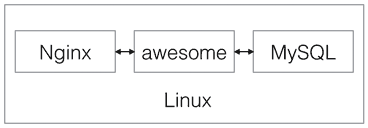Nginx负责分发请求：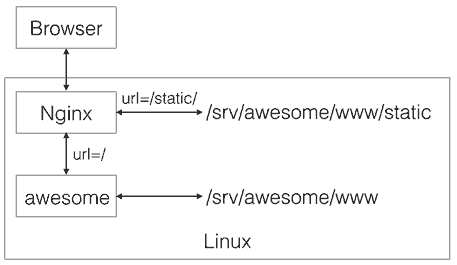``````/
+- srv/
+- awesome/       <-- Web App根目录
+- www/        <-- 存放Python源码
|  +- static/  <-- 存放静态资源文件
+- log/        <-- 存放log
``````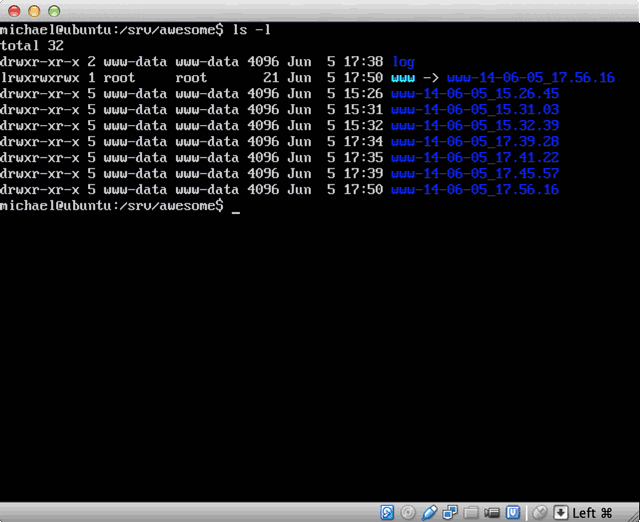Nginx可以作为服务进程直接启动，但gunicorn还不行，所以，Supervisor登场！Supervisor是一个管理进程的工具，可以随系统启动而启动服务，它还时刻监控服务进程，如果服务进程意外退出，Supervisor可以自动重启服务。

• Nginx：高性能Web服务器+负责反向代理；

• Supervisor：监控服务进程的工具；

• MySQL：数据库服务。

``````\$ sudo apt-get install nginx supervisor python3 mysql-server
``````

``````\$ sudo pip3 install jinja2 aiomysql aiohttp
``````

``````\$ mysql -u root -p < schema.sql
``````

#### 部署

``````\$ easy_install fabric
``````

Linux服务器上不需要安装Fabric，Fabric使用SSH直接登录服务器并执行部署命令。

``````awesome-python-webapp/
+- fabfile.py
+- www/
+- ...
``````

Fabric的脚本编写很简单，首先导入Fabric的API，设置部署时的变量：

``````# fabfile.py
import os, re
from datetime import datetime

# 导入Fabric API:
from fabric.api import *

# 服务器登录用户名:
env.user = 'michael'
# sudo用户为root:
env.sudo_user = 'root'
# 服务器地址，可以有多个，依次部署:
env.hosts = ['192.168.0.3']

# 服务器MySQL用户名和口令:
db_user = 'www-data'
``````

``````_TAR_FILE = 'dist-awesome.tar.gz'

def build():
includes = ['static', 'templates', 'transwarp', 'favicon.ico', '*.py']
excludes = ['test', '.*', '*.pyc', '*.pyo']
local('rm -f dist/%s' % _TAR_FILE)
with lcd(os.path.join(os.path.abspath('.'), 'www')):
cmd = ['tar', '--dereference', '-czvf', '../dist/%s' % _TAR_FILE]
cmd.extend(['--exclude=\'%s\'' % ex for ex in excludes])
cmd.extend(includes)
local(' '.join(cmd))
``````

Fabric提供`local('...')`来运行本地命令，`with lcd(path)`可以把当前命令的目录设定为`lcd()`指定的目录，注意Fabric只能运行命令行命令，Windows下可能需要Cgywin环境。

`awesome-python-webapp`目录下运行：

``````\$ fab build
``````

``````_REMOTE_TMP_TAR = '/tmp/%s' % _TAR_FILE
_REMOTE_BASE_DIR = '/srv/awesome'

def deploy():
newdir = 'www-%s' % datetime.now().strftime('%y-%m-%d_%H.%M.%S')
# 删除已有的tar文件:
run('rm -f %s' % _REMOTE_TMP_TAR)
# 上传新的tar文件:
put('dist/%s' % _TAR_FILE, _REMOTE_TMP_TAR)
# 创建新目录:
with cd(_REMOTE_BASE_DIR):
sudo('mkdir %s' % newdir)
# 解压到新目录:
with cd('%s/%s' % (_REMOTE_BASE_DIR, newdir)):
sudo('tar -xzvf %s' % _REMOTE_TMP_TAR)
# 重置软链接:
with cd(_REMOTE_BASE_DIR):
sudo('rm -f www')
sudo('ln -s %s www' % newdir)
sudo('chown www-data:www-data www')
sudo('chown -R www-data:www-data %s' % newdir)
# 重启Python服务和nginx服务器:
with settings(warn_only=True):
sudo('supervisorctl stop awesome')
sudo('supervisorctl start awesome')
``````

#### 配置Supervisor

``````[program:awesome]

command     = /srv/awesome/www/app.py
directory   = /srv/awesome/www
user        = www-data
startsecs   = 3

redirect_stderr         = true
stdout_logfile_maxbytes = 50MB
stdout_logfile_backups  = 10
stdout_logfile          = /srv/awesome/log/app.log
``````

``````\$ sudo supervisorctl reload
\$ sudo supervisorctl start awesome
\$ sudo supervisorctl status
awesome                RUNNING    pid 1401, uptime 5:01:34
``````

#### 配置Nginx

Supervisor只负责运行gunicorn，我们还需要配置Nginx。把配置文件`awesome`放到`/etc/nginx/sites-available/`目录下：

``````server {
listen      80; # 监听80端口

root       /srv/awesome/www;
access_log /srv/awesome/log/access_log;
error_log  /srv/awesome/log/error_log;

# server_name awesome.liaoxuefeng.com; # 配置域名

# 处理静态文件/favicon.ico:
location /favicon.ico {
root /srv/awesome/www;
}

# 处理静态资源:
location ~ ^\/static\/.*\$ {
root /srv/awesome/www;
}

# 动态请求转发到9000端口:
location / {
proxy_pass       http://127.0.0.1:9000;
}
}
``````

``````\$ pwd
/etc/nginx/sites-enabled
\$ sudo ln -s /etc/nginx/sites-available/awesome .
``````

``````\$ sudo /etc/init.d/nginx reload
``````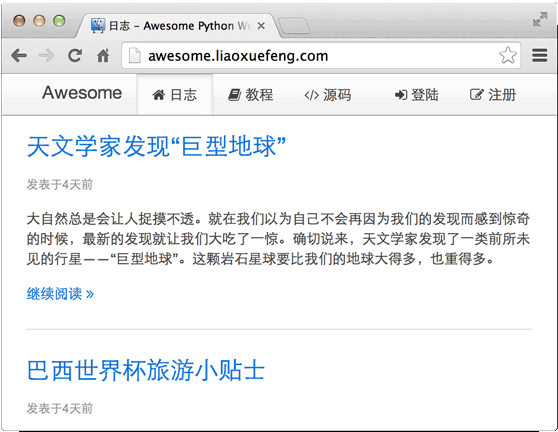``````\$ fab build
\$ fab deploy
``````

#### 友情链接

http://mirrors.163.com/

http://mirrors.sohu.com/

day-15

## Day 16 - 编写移动App

#### 开发iPhone版本day-16

# FAQ

#### 如何获取当前路径

``````# -*- coding:utf-8 -*-
# test.py

import os

print(os.path.abspath('.'))
``````

``````\$ python3 test.py
/Users/michael/workspace/testing
``````

#### 如何获取当前模块的文件名

``````# -*- coding:utf-8 -*-
# test.py

print(__file__)
``````

``````\$ python3 test.py
test.py
``````

#### 如何获取命令行参数

``````# -*- coding:utf-8 -*-
# test.py

import sys

print(sys.argv)
``````

``````\$ python3 test.py -a -s "Hello world"
['test.py', '-a', '-s', 'Hello world']
``````

`argv`的第一个元素永远是命令行执行的`.py`文件名。

#### 如何获取当前Python命令的可执行文件路径

`sys`模块的`executable`变量就是Python命令可执行文件的路径：

``````# -*- coding:utf-8 -*-
# test.py

import sys

print(sys.executable)
``````

``````\$ python3 test.py
/usr/local/opt/python3/bin/python3.4
``````

# 期末总结

Python非常适合初学者用来进入计算机编程领域。Python属于非常高级的语言，掌握了这门高级语言，就对计算机编程的核心思想——抽象有了初步理解。如果希望继续深入学习计算机编程，可以学习C、JavaScript、Lisp等不同类型的语言，只有多掌握不同领域的语言，有比较才更有收获。